# Four-Vectors (4-Vectors) & Lorentz Invariants of Special Relativity: A Study of Elegant Physics The Ultimate Four-Vector & Lorentz Invariant Reference

The Four-Vectors (4-Vectors) and Lorentz Invariants of Special Relativistic (SR) theory are fundamental entities that accurately, precisely, and beautifully describe the physical properties of the world around us. While it is known that SR is not the "deepest" theory, it is valid for the majority of the known universe. It is believed to apply to all forms of interaction, including that of fundamental particles and quantum effects, with the only exception being that of large-scale gravitational phenomena, where spacetime itself is significantly curved, for which General Relativity (GR) is required. The SR 4-vector notation is one of the most powerful tools in understanding the physics of the universe, as it simplifies a great many of the physical relations.SiteMap of SciRealm | About John | John's Resume/C.V./Bio | Send email to John John's Science, Math, Philosophy Stuff: The Science Realm: John's Virtual Sci-Tech Universe | 4-Vectors | Ambigrams | Antipodes | Covert Ops Fragments | Cyrillic Projector | Forced Induction (Sums Of Powers Of Integers) | Fractal Tree | Frontiers | IFS Fractals (Assembly) | IFS Fractals (Javascript) | JavaScript Graphics | Kid Science | Kryptos | Philosophy | Photography | Physics-Planck Constant Measurement Via LEDs | Prime Sieve | QM from SR | QM from SR-Simple RoadMap | QM from SR-Simple RoadMap (PDF) | SR 4-Vector & Tensor Calculator |Quantum Phase | Quotes | RuneQuest Cipher Challenge | Secret Codes & Ciphers | Scientific Calculator (complex capable) | Science+Math | Sci-Pan Pantheist Poems | Stereograms | SuperMagicSqr4x4 |Turkish Grammar |Melike Wilson Art Site last modified: 2021-Aug Welcome to Relativistic Quantum Reality: Virtual worlds of imaginary particles: The dreams stuff is made of: Life, the eternal ghost in the machine... This site is dedicated to the quest for knowledge and wisdom, through science, mathematics, philosophy, invention, and technology.  May the treasures buried herein spark the fires of imagination like the twinkling jewels of celestial light crowning the midnight sky... Quantum Mechanics is derivable from Special Relativity See SRQM - QM from SR - Simple RoadMap (.html) See SRQM - QM from SR - Simple RoadMap (.pdf) See SRQM - QM from SR - Summary (.pdf) See SRQM - Fundamental Constants (.pdf) See SRQM - Online SR 4-Vector & Tensor Calculator See Online Complex-capable RPN Scientific Calculator ***Learn, Discover, Explore***#### Introduction

This remains a work in progress.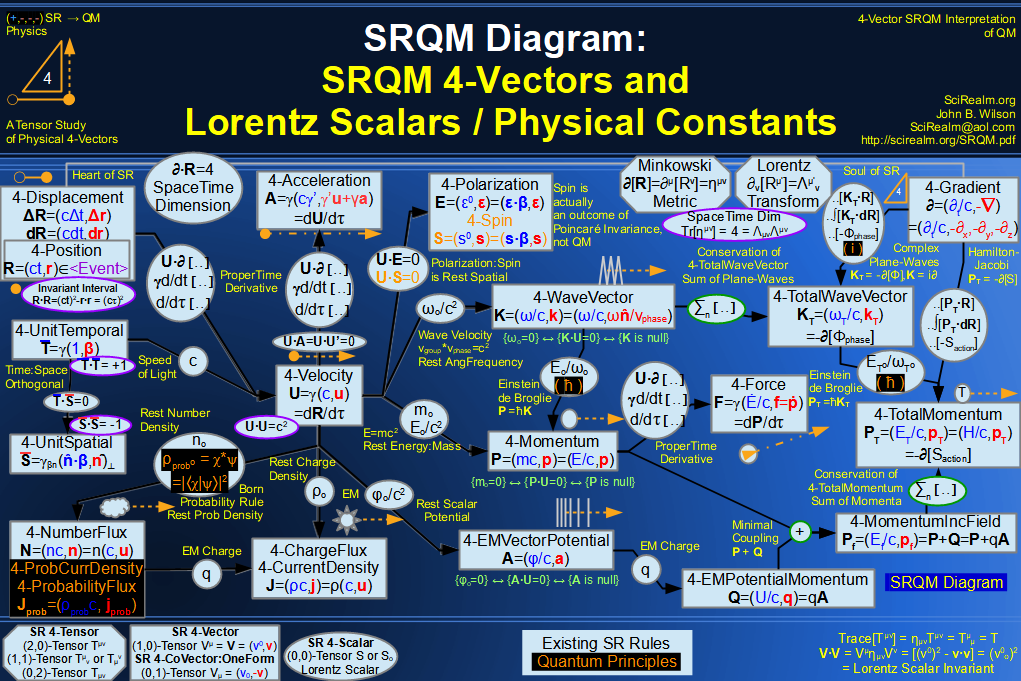A vector is a mathematical object which has both magnitude and direction. It is a powerful tool for describing physical phenomena. A common 3-vector is the velocity vector (vx, vy, vz), which tells you in what direction and how fast something is moving.  One might use the (x, y, z) coordinates to write down the velocity vector of some object in the laboratory. That would be an example of a rectilinear coordinate system. Another person might use a coordinate system that is rotated wrt. the first observer, with components (y', x', z). The same vector might also be described by the (r, θ, φ) spherical coordinate system. Within a given coordinate system, each component is typically orthogonal to each other component. While these different coordinate systems will usually have different numbers in the vector 3-tuple, they nevertheless describe the same vector and the same physics. Hence, the vector can be considered the "primary" element, which is then described by any number of different coordinate systems, which simply represent one point-of-view of the given vector.

The extension of 3-vectors to that of 4-vectors is a simple idea. Let's imagine some <event> in spacetime. The location of the <event> in the Newtonian world would be it's 3-position (x,y,z), and the time (t) at which it occurs. In the Newtonian world these are totally separate ideas. SR unites them into a single object. The location of the <event> in the SR world would be it's 4-position (ct,x,y,z). All that we have done is to insert the time (t) into the vector as another component. The factor of (c) is put with it to make the dimensional units work out right. ( [m/s]*[s] = [m]). So, each component now has overall units of [m] for this 4-vector. This rather simple idea, combined with the postulates of SR, lead to some amazing results and elegant simplifications of physical concepts...

There are two postulates which lead to all of SR-Special Relativity:
(1) The laws of physics are the same for all inertial reference frames. This means the form of the physical laws should not change for different inertial observers. This can be also restated as "All inertial observers measure the same interval magnitude between two <event>'s". I say it this way because all of experimental physics ultimately boils down to taking a measurement.  Yet another way to say it is that the result of any Poincaré Transformation (includes Lorentz Transformations) leaves the Invariant Interval unchanged.
(2) The speed of light (c) in vacuum is the same for all inertial reference frames. This is the result of millions of independent measurements, all confirming the same observation. This differentiates SR from classical Galilean invariance, which also obeys the first postulate.

4-vectors are tensorial entities which display Poincaré Invariance, meaning they leave invariant the differential squared interval (ds)2 = (cdt)2-dx2-dy2-dz2. A consequence of this invariant measurement is that any physical equation which is written in Poincaré Invariant form is automatically valid for any inertial reference frame, regardless of how coordinate systems are arranged. Transformations which leave these vectors unchanged include fixed translations through space and/or time, rotations through space, and boosts (coordinate systems moving with constant velocity) through spacetime. Since 4-vectors are tensors, and Poincaré Invariant, they can be used to describe and explain the physical properties that are observed in nature. Although the vector components may change from one reference frame to another, the 4-vector itself is an invariant, meaning that it gives valid physical information for all inertial observers. Likewise, the scalar products of Lorentz Invariant 4-vectors are themselves invariant quantities, known as Lorentz Scalars. Lorentz Invariance is a subset of the more general Poincaré Invariance.

The reason that I really like 4-vectors and their notation is that they beautifully and elegantly display the relations between lots of different physical properties. They also devolve very nicely into the limiting/approximate Newtonian cases of {|v|<<c} by letting {γ →1 and dγ/dt →0}. SR tells us that several different physical properties are actually dual aspects of the same thing, with the only real difference being one's point of view, or reference frame. Examples include: (Time , Space), (Energy , Momentum), (Power , Force), (Frequency , WaveNumber), (ChargeDensity , CurrentDensity), (EM-ScalarPotential , EM-VectorPotential), (Time Differential, Spatial Gradient), etc. Also, things are even more related than that. The 4-Momentum is just a constant times 4-Velocity. The 4-WaveVector is just a constant times 4-Momentum. In addition, the very important conservation/continuity equations seem to just fall out of the notation. The universe apparently has some simple laws which can be easy to write down by using a little math and a super notation.

#### Abbreviations

QM = Quantum Mechanics   SR = Special Relativity
SM = Statistical Mechanics   GR = General Relativity

#### Units of Measure - (SI variant, mksC)

 length/time [m] meter <*> [s] second Count of the quantity of separation or distance; Location of 's in spacetime mass [kg] kilogram Count of the quantity of matter; (the "stuff" at an ) EMcharge [C] Coulomb Count of the quantity of electric charge; the Coulomb is more fundamental than the Ampere Amperes are just moving Coulombs temperature [ºK] Kelvin Count of the quantity of heat (statistical)

#### Useful SR Quantities

Velocity
vgroup or v or u: v = cβ = c2/vp = group velocity = <event> velocity= [0..c], {u is historically used in SR notation}
vphase or vp:       vp = c/β = c2/v = phase velocity = celerity = [c..Infinity]

Minkowski Flat (Pseudo-Euclidian) Spacetime Metric:
ημν = ημν = SR gμν = Diag[+1,-1,-1,-1]

Dimensionless SR Factors:
β = (v/c) = (vgroup/c) = (c/vphase)= [0..1]: Relativistic Beta factor, the fraction of the speed of light c
β = (u/c): Vector form of Beta factor, u is the velocity
γ[u] = dt/dτ: Lorentz Gamma Scaling Factor (Relativistic Gamma factor)
γ = (1 / √[1-(v/c)2] ) = (1 / √[1-(u·u/c2)] ): Lorentz Gamma Scaling Factor (~1 for v<<c), (>>1 for v~c)
γ = (1 / √[1-β2] ) = (1 / √[1-β·β] ): Lorentz Gamma Scaling Factor (~1 for β<<1), (>>1 for β~1)
γ = (1 / √[1-β2] ) = 1/√[(1+|β|)/(1-|β|)] : Useful for Doppler Shift Eqns
φ = Ln[γ(1+ β)] ~ Atanh[β]: BoostParameter/Rapidity (which remains additive in SR, unlike v)
eφ = γ(1+β) = √[(1+β)/(1-β)]
β = Tanh[φ], γ = Cosh[φ], γβ = Sinh[φ], φ = Rapidity (which remains strictly additive in SR, unlike v)
D = 1 / [γ(1 - β Cos[θ] )] = 1 / [γ(1 - β·n )]: Relativistic Doppler Factor (sometimes called a relativistic beaming factor)
D+ = γ(1 + β Cos[θ] ): Forward jet Doppler shift
D- = γ(1 - β Cos[θ] ): Counter-jet Doppler shift

Temporal Factors:
τ = t / γ : Proper Time = Rest Time (time as measured in a frame at rest)
dτ = dt / γ : Differential of Proper Time
d/dτ = γ d/dt = U·∂ : Differential wrt Proper Time

Useful SR Formulas:
V·V = Vo·Vo : Invariant interval is often easier to calculate in rest frame coordinates
√[1+x] ~ (1+x/2) for x ~ 0 : Math relation often used to simplify Relativistic eqns. to Newtonian eqns.
1/√[1+x] ~ (1-x/2) for x ~ 0 : Math relation sometimes used to simplify Relativistic eqns. to Newtonian eqns.
δuv = Delta function = (1 if u = v, 0 if u ≠ v)
γ = (1 / √[1-(u·u/c2)]) = c/√[c2-v2] = c/√[c2-u·u]
γ2 = c2/(c2-v2) = c2/(c2-u·u) = 1/(1- β2)
c22 = (c2-v2)
v γ = c √[γ2-1]
β γ = √[γ2-1]
(1-β22 = 1
(1-β2) = 1/γ2
β2γ2 = γ2-1
β2γ2 +1 = γ2
β2γ = (γ-1/γ)
c2 dγ = γ3 v dv
d(γ v) = c2 dγ / v  = γ3 dv
dγ = γ3 v dv / c2 = γ3 β dβ
dβ = dv / c
dγ/dv = γ3 v / c2
d(γ-1)/dv = - γ v / c2
γ' = dγ/dt = (γ3 v dv/dt)/c2 = (γ3 u·a)/c2 = (u·ar)/c2
γ'' = dγ'/dt = d2γ/dt2 = (γ3/c2)*[(3γ2/c2)(u·a)2 + (u'·a) + (u·a')]
u2 = u2
u·u' = uu' = ua
(|u x a|)2 + (u·a)2 = u2a2
sin2     +   cos2 = 1
(∇·∇)[1/r] = Δ[1/r] = -4πδ3(r)
(∇·∇)[1/|r-r'|] = Δ[1/|r-r'|] = -4πδ3(r-r') Green's function for Poisson's Eqn

#### SR Notation Used

**NOTE**
All results below use the time-positive SR Minkowski Metric ημν = Diag[+1,-1,-1,-1].
If you wish to do GR, with other metrics gμν, then some results below may need GR modification, such as the GR √[-g] for whichever metric you are using...
You have been warned.

There are several different SR notations available that are, mathematically speaking, equivalent.
However, some are easier to employ than others. I have used that one which seems the most practical and least error-prone.
If you mix notations, you will get errors! Always check notation conventions in SR & 4-Vector references, they are all relative ;-)

Minkowski SR Metric (time 0-component positive), for which {in Cartesian coordinates} ημν = ημν = SR gμν = SR gμν = Diag[+1,-1] = Diag[+1,-1,-1,-1]
Signature[ημν] = -2

Generic 4-Vector Definition:
A = Au = (a0,a) = (a0,a1,a2,a3)    time (a0) in the 0th coord. ( some alternate notations use time as a4 )

Specific coordinate system representations:
A = Au = (a0,a) = (a0,a1,a2,a3) => (at,ax,ay,az) {for rectangular/Cartesian coords}
A = Au = (a0,a) = (a0,a1,a2,a3) => (at,ar,aθ,az) {for cylindrical coords}
A = Au = (a0,a) = (a0,a1,a2,a3) => (at,ar,aθ,aφ) {for spherical coords}

Note that the superscripted variables are not exponents, they are upper tensor indices

Intervals:
TimeLike/Temporal (+ interval) = 0 coordinate  ( some alternate notations use time as - interval and space as the + interval)
LightLike/Null         (0 interval)
SpaceLike/Spatial    (- interval) = 1,2,3 coordinates

Temporal Components: Future(+), Now(0), Past(-)

4-Vector Name: always references the "Spatial" 3-vector component (basically trying to extend the Newtonian 3-vector to SR 4-vector)
4-Vector Magnitude: usually references the "temporal" scalar component (because many vectors in the rest frame only have a temporal component)
4-Vector Tensor Indices: I use the convention of [Greek symbols dim{0..3} = time+space], [Latin symbols dim{1..3} = only space]
4-Vector Symbols: A = Aμ = (a0,a) = (a0,ai) = (a0,a1+a2+a3) = (a0,a1,a2,a3), where the raised index indicates dimension, not exponent
4-Vector Definition: A = Aμ , always references the upper tensor index unless otherwise noted
4-Vector c-Factor: almost always applied to "Temporal" scalar component, as necessary to give consistent dimensional units for all vector components (a0,a1,a2,a3) <==> (ct,x,y,z) = (ct,x)
*Note* c-Factor can be on the top, as ( ct , x , y , z ) = [m], or on bottom, as ( E/c , px , py , pz ) = [kg m s-1].  It all depends on the particular 4-Vector and its components.
*Note* P = (E/c,p) = (mc,p); the 4-Momentum is a good case showing top or bottom, with E = mc2
4-Vector Computer HTML Representation:
SR 4-vector = {BOLD UPPERCASE} = A
time scalar component = {regular lowercase} = a0
space 3-vector component = {bold lowercase} = a = ai = (a1,a2,a3)

Contraction & Dilation Relativistic Component: v --> vo in a rest-frame, typically v = γ vo (dilation) or v = (1/γ) vo (contraction)
eg.
t = γ to (time dilation) - pertains to temporal separation between two <event>'s
L = (1/γ) Lo (length contraction) - pertains to the spatial separation between two parallel world lines

Generally, time-like quantities get dilated, space-like quantities get contracted by motion
Also, I typically denote "at-rest" invariant quantities with a "naught", or "o", i.e.:
Lo (invariant rest length = proper length), relativistic length L = (1/γ) Lo
Vo (invariant rest volume), relativistic volume V = (1/γ) Vo
mo (invariant rest mass), relativistic mass m = γ mo
Eo (invariant rest energy), relativistic energy E = γ Eo
ωo (invariant rest ang-frequency), relativistic ang-frequency ω = γ ωo
ρo (invariant rest charge-density), relativistic charge-density ρ = γ ρo
no (invariant rest number-density), relativistic number-density n = γ no
to (invariant rest time = proper time), relativistic time t = γ to = γ τ
etc.
This avoids the confusion of some texts which use just "m" as invariant mass, or just "ρ" as invariant charge-density.
It also helps to avoid confusion such as:
If the mass m of an object increases with velocity, wouldn't it have be a black hole in some reference frames (near c), since the mass increases with velocity.
Answer - no.  The rest mass mo does not change.  The relativistic mass is simply an "apparent" mass, how the object is velocity-related to an observer, not how much "stuff" is in it...
The apparent increase is fully due to the gamma factor( γ ), which is simply an indication of the amount of relative motion.

Imaginary unit: ( i ) used only for QM phenomena, not for SR frame transformations or metric.  To follow up on a quote from MTW " ict was put to the sword ".
This allows all the purely SR stuff to use only real numbers.  Imaginary/complex stuff apparently only enters the scene via QM.
( some alternate notations use the imaginary unit ( i ) in the components/frame transformations/metric )

So, in summary, this notation allows:
easy recovery of Newtonian cases by allowing (γ→1, dγ→0) when (|v|<<c)
easy separation of SR vs Newtonian concepts, with the Newtonian 3-vector (a) extending naturally into the SR 4-vector (A)
easy separation of SR vs QM concepts, no ict's -- ( i ) only enters into QM concepts, such as Photon Polarization, Quantum Probability Current, etc.
easy separation of relativistic quantities vs. invariant quantities, E = γ Eo
reduction in number of minus signs (-), eg. U·U = c2, P·P = (moc)2: the square magnitudes of velocity, momentum, wavevector, and other velocity-based vectors are positive

#### Minkowski SR Spacetime Metric

The main assumption of SR, or GR for that matter, is that the structure of spacetime is described by a metric gμν. A metric tells how the spacetime is put together, or how distances are measured within the spacetime. These distances are known as intervals. In GR, the metric may take a number of different values, depending on various circumstances which determine its curvature. We are interested in the flat/pseudo-Euclidean spacetime of SR, also known as the Minkowski Metric, for which gμν => ημν = ημν = Diag[+1,-1,-1,-1].

 "Flat" SpaceTime ημν = gμν{SR} t x y z t 1 0 0 0 x 0 -1 0 0 y 0 0 -1 0 z 0 0 0 -1

ημα ημβ = δαβ = (4 if α = β for Minkowski)
g = - Det[gμν] = -1 (for Minkowski) not a scalar invariant
Sqrt[-g]ρ: Scalar density

There are other ways of defining the metrics and 4-vectors available in SR which lead to the same physical results, but this particular notation has some nice qualities which place it above the others. First, it shows the difference between time and space in the metric. We perceive time differently than space, despite there being only spacetime. Also, this metric gives all of the SR relations (frame transformations) without using the imaginary unit ( i ) in the transforms. This is important, as ( i ) is absolutely essential for the complex wave functions once we get to QM. It is not needed, and would only complicate and confuse matters in SR. This metric will allow us to separate the "real" SR stuff from the "complex/imaginary" QM stuff easily. It also allows for the possibility of complex components in SR 4-vectors.  The choice of +1 for the time component simplifies the derived equations later on, as it allows rest-frame square-magnitudes to be positive for most quantities of interest.

#### SR 4-Vector Basics

ημν = SR gμν = SR gμν = DiagonalMatrix[1,-1,-1,-1]: Minkowski Spacetime Metric-the "flat" spacetime of SR {in Cartesion coordinates}

A = Aμ = (a0,ai) = (a0,a1,a2,a3) => (at,ax,ay,az) : Typical SR 4-vector (using all upper indices)
Aμ = (a0,ai) = (a0,a1,a2,a3) => (at,ax,ay,az) : Typical SR 4-covector (using all lower indices)

We can always get the alternate form by applying the Minkowski Metric Tensor: Aμ = ημνAν and Aμ = ημν Aν
Basically, this has the effect of putting a minus sign on the space component(s)

A = Aμ = (a0,ai) = (a0,a1,a2,a3) = (a0, a1, a2, a3)  = (a0,a) :Typical SR 4-vector (all upper indices)
Aμ = (a0,ai) = (a0,a1,a2,a3) = (a0,-a1,-a2,-a3) = (a0,-a): Typical SR 4-covector (converted using Metric Tensor)

It is occasionally convenient to choose a particular basis to simplify component calculations
Typical bases are rectangular, cylindrical, spherical

A = Aμ = (a0,ai) = (a0,a1,a2,a3) = (a0,a)  = (a0,a1,a2,a3)      = (a0,a) => (at,ax,ay,az) :Typical SR 4-vector (choosing the rectangular basis)
Aμ = (a0,ai) = (a0,a1,a2,a3) = (a0,-a) = (a0,-a1,-a2,-a3) = (a0,-a) => (at,ax,ay,az) =  (at,-ax,-ay,-az) : Typical SR 4-covector (choosing the rectangular basis)

B = Bμ  = (b0,b1,b2,b3) = (b0,b) = (bt,bx,by,bz) : Another typical SR 4-vector

A·B = ημν Aμ Bν = Aν Bν = Aμ Bμ = +a0b0-a1b1-a2b2-a3b3 = (+a0b0-a·b): The Scalar Product or Invariant Product relation, used to make SR invariants

c(A + B) = (cA + cB) scalar multiplication
A·A = A2 = (+a02 - a12 - a22 - a32) = (+a02 - a·a) magnitude squared, which can be { - , 0 , + }
A = |A| = √|A2| >= 0 absolute magnitude or length, which can be { 0 , + }
A·B = B·A commutative, with the exception of the () operator, since it only acts to the right
A·(B + C) = A·B + A·C distributive
d(A·B) = d(AB + A·d(B) differentiation
B = d(A)/dθ, where θ is a Lorentz Scalar Invariant

Aproj = (A·B)/(B·B) B = Projection of A along B

A|| = (A·B)/(B·B) B = Component of A parallel to B
A = A - A||
A =A - (A·B)/(B·B) B = Component of A perpendicular to B

Let A = (a0,a) be a general 4-Vector and T = U/c = γ(c, u)/c = γ(1, β) = (γ, γβ) be the unit-temporal 4-Vector
Then...
(A·T) = (a0,a)·γ(1, β) = γ(a0-a·β) = 1(a0o-a·0) = a0o
which is a Lorentz Invariant way to get (a0o), the rest temporal component of A

Let A = (a0,a) be a general 4-Vector and S  = γβn(β·n, n) be the unit-spatial 4-Vector
Then...
-(A·S) = -(a0,a)·γβn(β·n, n) = -γβn(a0β·n, a·n) = -1(a0a·0 - a·n) = a·n
which is a Lorentz Invariant way to get (a·n), the rest spatial component of A along the n direction

If A is a time-like vector, then you can also do the following:
(A·T)2 - (A·A) = (a0o)2 - (a0a0-a·a) = (a0o)2 - (a0)2 + (a·a) = (a·a)
Sqrt[(A·T)2 - (A·A)] = Sqrt[(a·a)] = |a|
which is the Lorentz Invariant way to get the magnitude |a|, the spatial component magnitude of a time-like A

Also:
(A·A) = (a0)2 - (a·a) = invariant = (a0o)2 ,where (a0o) is the Lorentz Scalar Invariant "temporal rest value" for those vectors that can be at rest, and just the invariant for others
(a0)2 = (a0o)2 + (a·a)

Consider the f ollowing identity:
γ = 1/Sqrt[1-β2]    :    γ2 = 1 + γ2β2
Multiply by the square of a Lorentz Scalar Invariant (a0o):
γ2(a0o)2 = (a0o)2 + γ2(a0o)2β2

Compare Terms:
(a0)2 = (a0o)2 + (a·a)
γ2(a0o)2 = (a0o)2 + γ2(a0o)2β2

Notice the following correspondences:
a0 = γa0o, The temporal component is the Gamma Factor times the rest value
a = γa0oβ =  a0β, The spatial component is temporal component times t he Beta Factor

Try it with the 4-Momentum P = (E/c,p)
(E/c) = γ(Eo/c) or E = γEo
p = γ(Eo/c)β = γ(Eo/c)(v/c) = γ(Eov/c2) = γ(mov) = γmov
p = (E/c)β = (mc)β = (mv) = γmov

If β=1 then |p| = E/c or E = |p|c, which is correct for photons
It also shows that as β → 1: γ → Infinity, (a0o) → 0, a0 = (γa0o) → some finite value

Special Relativity is interesting in that it is one area of physics where {Infinity*Zero = Finite Value} for certain variables.
eg. E = γEo
For photons, the rest energy Eo = 0,  the gamma factor γ = Infinity, but the overall energy of photon E = finite value for a given obs erver.
One can do this with any SR rest value variable.  Always pair γ={1..Infinity} with ao={large..0} to get a finite value a = γao={something finite}

These correspondences can also be generated by letting A = LorentzScalar (a0o) * TemporalUnit 4-Vector T
A = (a0o)T = (a0o)γ(1,β) = γ(a0o)(1,β) = (γa0o)(1,β) = (a0)(1,β) = (a0,a0β) = (a0,a)
If β0 , then A --> (a0,a0β) → (a0o,0), which has (A·A) = (a0o)2 as expected
If β1 , then A --> (a0,a0n) → a0(1,n), which has (A·A) = (a0)2 (12 - n·n) = 0 as expected

==========
if A·A = const
then
dA1dA2dA3 / A0
dA0dA2dA3 / A1
dA0dA1dA3 / A2
dA0dA1dA2 / A3
are all scalar invariants
from Jacobian derivation
============

if Aμ dXμ = invariant for any dXμ, then Aμ is a 4-vector

ημν Λμα Λνβ = ηαβ  This is basically the reason why the Scalar Product relation gives invariants
ημν (A'μ)(B'ν) = ημνμαAα)(ΛνβBβ) = ημνμαΛνβ)(Aα)(Bβ) = ηαβ (Aα)(Bβ) = ηαβ (AαBβ)
Thus, A'·B' = A·B, where the primed 4-vectors are just Lorentz Transformed versions of the unprimed ones

Λαμ Λμβ = dαβ

A'μ = Λμν Aν: Lorentz Transform (Transformation tensor which gives relations between alternate boosted inertial reference frames)

Λμ'ν = (∂Xμ'/∂Xν)

Λμν = (for x-boost)

 γ -(vx/c)γ 0 0 -(vx/c)γ γ 0 0 0 0 1 0 0 0 0 1

or

 γ -βxγ 0 0 -βxγ γ 0 0 0 0 1 0 0 0 0 1

General Lorentz Transformation
Λμν = (for n-boost)

 γ -βxγ -βyγ -βzγ -βxγ 1+(γ-1)(βx/β)2 ( γ-1)(βxβy)/(β)2 ( γ-1)(βxβz)/(β)2 -βyγ ( γ-1)(βyβx)/(β)2 1+( γ-1)(βy/β)2 ( γ-1)(βyβz)/(β)2 -βzγ ( γ-1)(βzβx)/(β)2 ( γ-1)(βzβy)/(β)2 1+( γ-1)(βz/β)2
General Lorentz Boost Transform using just vectors & components-Thank you Jackson, Master of Vectors! Chap. 11
β = v/c, β = |β|, γ = 1/√[1-β2]
a0' = γ(a0-β·a)
a' = a+(β·a)β(γ-1)/β2β a0

a0' = γ(a0-β·a) Temporal component
a||' = γ(a||-βa0) Spatial parallel component
a
' = a Spatial perpendicular components

Contraction & Dilation Relativistic Component: v --> vo in a rest-frame, typically v = γ vo (dilation) or v = (1/γ) vo (contraction)
eg.
t = γ to (time dilation) - pertains to temporal separation between two <event>'s
L = (1/γ) Lo (length contraction) - pertains to the spatial separation between two parallel world lines

Since the transformations are symmetric in the temporal and spatial parts of the 4-vector, it is somewhat confusing how the gamma factor is inversely related for times compared to lengths.  The time dilation compares the separation in proper time between <event>'s on the worldline of a single particle.  The length contraction is comparing separations between differing <event>'s however.  The length must be measured along lines of simultaneity, and the <event>'s of the endpoints while simultaneous n the rest frame, are not simultaneous in the moving frame.

We are also able to use the Rapidity
φ = Ln[γ(1+ β)] = Rapidity (which remains strictly additive in SR, unlike v)
φ = aTanh[|p|c/E] = (1/2) Ln[(E+|p|c)/(E-|p|c)]
eφ = γ(1+β) = √[(1+β)/(1-β)]
β = Tanh[φ], γ = Cosh[φ], γβ = Sinh[φ]

φAC = φAB + φBC
Rapidity of C wrt. A = Rapidity of B wrt. A + Rapidity of C wrt. B, provided that A,B,C are co-linear
i.e. Rapidity is strictly additive only for co-linear points

Λuv = (for x-boost, y & z unchanged)

 Cosh[φ] -Sinh[φ] 0 0 -Sinh[φ] Cosh[φ] 0 0 0 0 1 0 0 0 0 1

Formally, this is like a rotation in 3-space, but becomes a hyperbolic rotation through spacetime for a Lorentz boost

Rz = (for x-y rotation about z-axis, t & z unchanged)

 1 0 0 0 0 Cos[φ] -Sin[φ] 0 0 Sin[φ] Cos[φ] 0 0 0 0 1

Time t = γ to --> Time Dilation (e.g. decay times of unstable particles increase in a cyclotron)
Length L = Lo/γ --> Length Contraction

#### Complex SR 4-Vectors

A few 4-vectors are known to have complex components. The Polarization 4-vector and ProbabilityCurrent 4-vector are a couple of these.
It will be assumed that all physical 4-vectors may potentially be complex, although, as far as I know, these only come into play via QM...

i = √[-1] :Imaginary Unit
e0: Unit vector in the temporal direction (typically not used since the temporal unit is always considered a scalar)
e1, e2, e3 :Unit Vectors in the spatial x, y, z directions (used instead of i, j, k so that there is no confusion with the imaginary unit i)

Note that for the following 4-vectors, the superscript is the tensor index, not exponentiation.

A = (a0c + a1c e1+ a2c e2+ a3c e3): Complex 4-vector has complex components, 1 along time and 3 along space
Scalar[A] = a0c: Just the time component
Vector[A] = a1c e1 + a2c e2 + a3c e3: Just the spatial components
A = Scalar[A] + Vector[A]

A = ( (a0r + a0i ) + (a1r + a1i ) e1 + (a2r + a2i ) e2 + (a3r + a3i ) e3 ): Complex 4-vector has real + imaginary components, 1 each along time and 3 each along space
Re[A] = ( (a0r ) + (a1r ) e1 + (a2r ) e2 + (a3r ) e3 ): Only the real components
Im[A] = ( (a0i ) + (a1i ) e1 + (a2i ) e2 + (a3i ) e3 ): Only the imaginary components
A = Re[A] + i Im[A]

A = (a0r + i a0i,ar + i ai) : Standard 4-vector
A* = (a0r - i a0i,ar - i ai): Complex conjugate 4-vector, just changes the sign of the imaginary component

A = (a0r + i a0i,ar + i ai) : A* = (a0r - i a0i,ar - i ai)
B = (b0r + i b0i,br + i bi) : B* = (b0r - i b0i,br - i bi)

A·B = [( a0r b0r - ar·br ) - ( a0i b0i - ai·bi )] + i [( a0r b0i - ar·bi ) + ( a0i b0r - ai·br )] : General scalar product
A·A = [( a0r2 - ar·ar ) - ( a0i2 - ai·ai )] + 2i [( a0r a0i - ar·ai )] = |A|2 : Scalar product of 4-vector with itself gives the magnitude squared
A·A* = [( a0r2 + a0i2 ) - ( ar·ar + ai·ai )] = Re[A·A*]: Scalar product of 4-vector with its complex conjugate is Real, thus Im[A·A*] = 0

∂·B = [( ∂/c∂tr b0r + r·br ) - ( ∂/c∂ti b0i + i·bi )] + i [( ∂/c∂tr b0i + r·bi ) + ( ∂/c∂ti b0r + i·br )]
= [( ∂/c∂tr b0r + r·br ) - ( ∂/c∂ti b0i + i·bi )]
= Re[∂·B]
The 4-Divergence of a Complex 4-Vector is Real, assuming that:
The real gradient acts only on real spaces & the imaginary gradient acts only on imaginary spaces, thus Im[∂·B] = 0
I believe this is due to the physical functions being complex analytic functions.

#### Fundamental/Universal Mathematical Constants

i = √[-1] :Imaginary Unit
π = 3.14159265358979... :Circular Const

#### Fundamental/Universal Physical Constants (Lorentz Scalars)

c = Speed of Light Const = 1/√[εoμo] ~ 2.99729x108 [m/s]
h = Planck's Constant - relates particle to wave - Action constant
ћ = (h/2π) = Planck's Reduced Const , aka. Dirac's Const - same idea as transforming between cycles and radians for angles
In essence, the reduced Planck constant is a conversion factor between phase (in radians) and action (in joule-seconds)
kB = Boltzmann's Const ~ 1.3806504(24)×10−23 [J/ ºK] relates temperature to energy
mo = Rest Mass Const (varies with particle type)
q = Electric Charge Const (varies with particle type)

Note:
I do not set various fundamental physical constants to dimensionless unity, (i.e. c = h = G = kB = 1).
While doing so may make the mathematics/geometry a bit easier, it ultimately obscures the physics.
While pure 4-Vectors may be Math, SR 4-Vectors is Physics. I prefer to keep the dimensional units.
Also, it is much easier to set them to unity in a final formula than to figure out where they go later if you need them.#### Fundamental/Universal Physical SR 4-Vectors (Lorentz Vectors)

4-Vector Name

4-Vector Components
(temporal, spatial)

Magnitude & Relation to other 4-vectors

Units (mksC) - Description

***Calculus***

4-Displacement
= 4-Delta
= 4-Difference

ΔR = (cΔt, Δr)
ΔX = (cΔt, Δx)

[m], Δt = Temporal Displacement, Δr or Δx = Spatial Displacement, (Finite Differences)

The 4-vector prototype, the "arrow" linking two <event>'s

4-Differential

dR = (cdt, dr)
dX = (cdt, dx)

[m], dt = Temporal Differential, dr or dx = Spatial Differential, (Infinitesimals)

= 4-Del or 4-Partial or 4-
(a vector operator)

The tensor gradient (one-form) is technically defined as
μ = ,μ = ∂/∂xμ = (∂t/c,) = (∂t/c,del)
=> (∂t/c, ∂x, ∂y, ∂z)
using the lower index

But the convention with 4-Vectors is to use upper indices
The 4-Gradient is the rare example with the upper tensor index having a negative spatial component

= ∂μ = ∂/∂xμ = (∂t/c, -) = (∂t/c, -del)
= (∂0/c, -) = (∂t/c, -)
=> (∂t/c, -∂x, -∂y, -∂z)
=> (∂/c∂t, -∂/∂x, -∂/∂y, -∂/∂z)

Since (∂t = ∂t), we typically write as ∂t

∂·∂ = (∂/c∂t,-)·(∂/c∂t,-) = (∂t/c,-)·(∂t/c,-)
= ∂2/c2∂t2-∇·∇ = ∂t2/c2-∇·∇
= (imoc / ћ)2
= -(moc / ћ)2

[V·R] = V, where V is not a function of 4-position R

from QM connection:
= (∂t/c, -)
=?= (∂t/c, βt/c)
=?= (vphase|-|/c, -)
The unit-temporal maybe/maybe not applies here

= X, as opposed to the 4-MomentumGradient P

[m-1], ∂ is the partial derivative, => (∂/∂x i + ∂/∂y j + ∂/∂z k) is the gradient operator

This is a very important 4-vector operator, often used to generate continuity equations
Used to obtain field-kinematics
It is technically a one-form, a dual of a regular 4-vector
μ = ∂/∂xu = (∂/c∂t, -) and ∂μ = ∂/∂xu = (∂/c∂t, )
∂·∂ is also known as the D'alembertian (Wave Operator Δ)

I sometimes write out (del) because the nabla/del symbol (∇)
is quite often displayed incorrectly in various browsers.
It should look like an inverted triangle when displayed correctly.

Let gμ = ∂μ f = ∂f / ∂xμ
Using the chain rule, one can show:
g'ν = ∂f '/∂x'ν = Σ ( ∂f / ∂xμ )( ∂xμ / ∂x'ν ) = ∂'ν f ' = ( ∂μ f )( ∂'ν xμ ) = (gμ)( ∂'ν xμ )
However, this appears to be a standard Lorentz transform
∂'μ = Λμνν[ function argument ] =  ∂ν[ function argument ] Λμν

importantly:
U·∂ = γ(∂/∂t + u·∇) = γ d/dt = d/dτ
The total derivative wrt. proper time is a Scalar Invariant

∂·X = (∂t/c,-)·(ct,x) = ((∂t/c)ct - (-·x)) = (∂tt) + (·x) = (1) + (3) = 4
The dimensionality of spacetime is 4.

∂[X] = ∂μ[Xν] = (∂t/c,-)[(ct,x)] =

[(∂t/c)ct, -∂xct, -∂yct, -∂zct,]
[(∂t/c)x,  -∂xx,  -∂yx,  -∂zx,]
[(∂t/c)y,  -∂xy,  -∂yy,  -∂zy,]
[(∂t/c)z,  -∂xz,  -∂yz,  -∂zz,]=

= Diag[(∂t/c)ct, -∂xx, -∂yy, -∂zz,]  = Diag[1,-1,-1,-1] = ημ ν
∂[X] = ημν
The 4-Gradient acting on the 4-Position gives the SR Metric

∂·J = (∂t/c,-)·(cρ, j) = ((∂t/c)cρ)- (-·j) = ∂tρ + ·j = 0 for a conserved ElectricCurrent

P = (E/c,p) = iћ = iћ (∂t/c, -)
Taking components:
Temporal: E = iћ ∂t
Spatial: p = -iћ
The Schrödinger QM relations

(∂·∂)G(X|X') = δ4(X-X')
The d'Alembertian on the 4D Green's function

The 4-Gradient of a Lorentz scalar is a 4-Vector
[V·R] = V, where V is not a function of R

K = (ω/c, k)
R = (ct, r)
K·R = -φEM = (ωt-k·r)
= (∂t/c, -)
[K·R] = (∂t/c, -)[ωt-k·r] = (ω/c, k) = K

used in QM as momentum-space
vector operator

P = ∂/∂Pμ = (c∂E, -p)
=> (c∂E, -∂px, -∂py, -∂pz)
=> (c∂/∂E, -∂/∂px, -∂/∂py, -∂/∂pz)

[kg-1 m-1 s], ∂E is the momentum-space partial derivative, p =  momentum-space gradient

X[P·X] = P
P[P·X] = X

used in QM as wave-space
vector operator

K = ∂/∂Kμ = (c∂ω, -k)
=> (c∂ω, -∂kx, -∂ky, -∂kz)
=> (c∂/∂ω, -∂/∂kx, -∂/∂ky, -∂/∂kz)

[m], ∂ω is the wave-space partial derivative, k = wave-space gradient

X[K·X] = K
K[K·X] = X

from the de Broglie relations, we get the Fourier transforms
X = -i K
K = i X

Example of crystal momentum velocity definition:
U = (1/ћ)K[P·U]
First, prove that this is true:

(1/ћ)K[P·U]
= K[K·U]
= K[(ω/c,k)·γ(c,u)]
= K[γ(ω - k·u)]
= γK[(ω - k·u)]
= γ(c∂ω, -k)[(ω - k·u)]
= γ(c∂ω[(ω - k·u)], -k[(ω - k·u)])
= γ(c∂ω[ω], -k [- k·u])
= γ(c∂ω[ω], +k[k·u])
= γ(c,u)
= U

Next, the part in the function
P1·U2 = γ[u2](E-p1·u2) = Erel

U = (1/ћ)K[P·U] = (1/ћ)K[Erel]
U = γ(c,u) = (1/ћ)(c∂ω, -k)[Erel]

Take the spatial part:
γ(c,u) = (1/ћ)(c∂ω, -k)[Erel]
γu = (1/ћ)(-k)[Erel]

Take the Newtonian limit:
u = (1/ћ)(-k)[Erel]

***Particle/<Event> Dynamics***

4-Position
4-<Event>

R = Rμ = (ct, r), eg. radial coords
=> (ct,r,θ,z)

X = Xμ = (ct, x), eg. Cartesian coords
=> (ct,x,y,z)

Q = Qμ = (ct, q), eg. Hamiltonian coords
=> (ct,q1,q2,q3)

*Note*
These coordinate choices do not affect the spacetime, they are simply choices of convenience, where mathematical expressions may take on a simpler looking form.

R·R = (Δs)2 = (ct)2-r·r = (ct)2-|r|2

{R·R = 0 for photonic path/null path}
{ct = |r| for photonic}

dR/dτ = U

[m], t = Time (temporal), r or x = 3-Position (spatial)

Location of an <Event>, the most basic 4-vector (when, where)
This is just a 4-Displacement with one of the <event>'s at the origin (0,0,0,0) of the chosen coordinate system
c = Speed-of-Light
often seen as X when choosing a Cartesian representation
often seen as R when choosing a radial/cylindrical/spherical representation

interesting derivation:
U = dR/dτ
dR = dτU
R = ∫dτU
let U be a constant, then R = τU = toU = toγ(c, u) = t(c, u) = (ct, ut) = (ct, x)
where τ = to and t = γto
time dilation τ = t / γ
Position is essentially the ProperTime * 4-Velocity, like some of the other flux 4-vectors

4-Velocity

U = dR/dτ
= γ(c, u) = dR/dτ = γdR/dt
= γ(c, ur) = γ(c, u)
= γ(c, cβ) = γc(1, β)
=> γ(c,ux,uy,uz)

{U = γcc(1,n), for light-like/photonic}
c is infinite, so basically U is not well-defined for photonic}

U
o = (c,0) in rest frame

U·U = c2
U1·U2 = γ[u1]γ[u2](c2-u1·u2
= γ[u12]c2 = γ[urel]c2

dU/dτ = A

4-Velocity is c*UnitTemporal 4-Vector
U = cT

[m s-1], γ = relativistic factor,  ur = Relativistic 3-Velocity, u = dr/dt = Newtonian 3-Velocity

ur = (r)' = r'
u = dr/dt = r'
thus, ur = u

"U is historically used instead of V"
Uo = (c,0), 4-Velocity is always future-pointing time-like
usually seen as U, sometimes as V
only 3 independent components since U·U = c2 = constant
the temporal component is determined by the spatial components

this actually gives a reason why several 4-vectors are a (Lorentz Scalar)*(4-Velocity):
It allows these new 4-vectors to have 4 independent components

interesting derivation:
Let general 4-vector A = k U = (ao,a) = kγ(c, u),where k is a constant
Then  A·U = k U·U = kc2
So, ao = kγc = γ A·U / c
And, a = kγu = (γ A·U / c2)u = (ao/c)u

Try 4-momentum P
P = (E/c,p) = mo U

E/c = γ P·U /c
E = γ P·U
or, in Hamiltonian formalism, H = E = γ P·U

4-Acceleration

A = dU/dτ
A = γ(c dγ/dt, dγ/dt ua) = dU/dτ = γ dU/dt
=d2R/dτ2
= γ(c dγ/dt, ar)
= γ(c γ', ar), where γ' = dγ/dt
= γ(c γ', γ' ua)
= γ(ar·u/c, ar), because A·U = 0
= γ2[(γ2/c)(u·a), a + (γ2/c2)(u·a) u]

Ao = (0,ao) in rest frame

A·A = -a2
=  - γ4[a2 + ( γ/c)2(u·a)2]
=  - γ6[a2 - (u x a)2/c2]

dA/dτ = J

U·dU/dτ = U·A = 0 : 4-Acceleration orthogonal to it's worldline

[m s-2], ar = Relativistic 3-Acceleration, a = du/dt = Newtonian 3-Acceleration
ar = (γur)' = γ' ur + γ ur' = γ' u + γ a = (γ3/c2)(u·a) u + γ a
a = du/dt = u'
γ' = dγ/dt = (γ3/c2)(u·a) = (u·ar)/c2

Interesting note:
The temporal component has units of frequency, before the c factor, and is given by γ(dγ/dt)=γ(γ')
γ(c γ')=γ(u·ar)/c
γ'=(u·ar)/c2
4-Spin also has a temporal component in this form, given by u·s/c
I now wonder if all 4-vectors which are tangent to the worldline possess this "cyclic" feature...

4-Jerk

J = dA/dτ
= γ dA/dt
=d3R/dτ3
= γ( c(dγ/dt)2 + cγ(d2γ/dt2), dγ/dt ar+γ dar/dt )
= γ( c γ'2 + c γ γ'', γ' ar + γ ar' )
= γ( c γ'2 + c γ γ'', jr )
where γ' = dγ/dt, γ'' = d2γ/dt2, ar' = dar/dt

dJ/dτ = S

[m s-3], jr = Relativistic 3-Jerk, j = da/dt = Newtonian 3-Jerk

jr = (γar)' = γ' ar + γ ar'
j = da/dt = a'
γ' = dγ/dt = (γ3/c2)(u·a)
γ'' = dγ'/dt = d2γ/dt2 = (γ3/c2)*[(3γ2/c2)(u·a)2 + (u'·a) + (u·a')]

4-Snap
= 4-Jounce

S = dJ/dτ
= γ dJ/dt
=d4R/dτ4

[m s-4], sr = Relativistic 3-Snap, s = dj/dt = Newtonian 3-Snap

sr = (γjr)' = γ' jr + γ jr'
s = dj/dt = j'

***Kinematics***

4-Momentum

P = moU = (Eo/c2)U = ћK

P
= (mc, p) = γmo(c,u) = (γmoc,γmou)
= (E/c, p) = (γEo/c2)(c,u)
= (E/c,βE/c) = (E/c,uE/c2)
= (E/c)(1,β) = (Eo/c)γ(1,β)
= (|p|/|β|, p) = (|p|c/|u|, p)
= (vphase|p|/c, p)

{P= (ћω/c)(1, n) = (E/c)(1, n) = (|p|, p) = |p|(1, n) , for light-like/photonic}

P
o = (Eo/c, 0) = (moc, 0) in rest frame

P = ((Eo + poVo)/c2)U, taking into account pressure*volume terms where
pressure p = po
volume V = Vo/ γ

P·P = (moc)2 = (Eo/c)2
{P·P = 0 for photonic}

dP/dτ = F

Lorentz Force - Covariant eqn. of motion for a particle in an EM field:
dPμ / dτ = q Fμν dXν / dτ
dPμ / dτ = q Fμν Uν
dPμ / dτ = q (∂uAν-∂νAu)Uν

4-Momentum is (moc)*UnitTemporal 4-Vector
P = (moc)T

4-Momentum inc. Spin
Ps = Σ·P = Σμν Pν
where Σμν is the Pauli Spin Matrix Tensor
Ps = (σ0 E/c,σ·p)
where σ0 is an identity matrix of appropriate spin dimension and σ is the Pauli Spin Matrix Vector

Ps·Ps = (σ0 E/c)2 - (σ·p)2 = (moc)2 = (Eo/c)2

4-Momentum inc. Spin in External Field
where:
H = ET = Hamiltonian = TotalEnergyOfSystem
pT = Total3-MomentumOfSystem

Ps = Σ·P
PT = P + qA
P = PT - qA
Ps = [σ0(ET/c-qφ/c) , σ·(pT-qa)] = (σ0 E/c,σ·p)

Ps·Ps = [σ0 (ET/c-qφ/c)]2 - [σ·(pT-qa)]2 = (moc)2 = (Eo/c)2

Note that the intrinsic spin is *not* something that must come from QM, the spin is an artifact of the Poincare Group, where mass and spin are the Casimir Invariants of the SR Poincare Group

[kg m s-1], E = Energy, pr = Relativistic 3-Momentum, p = mdr/dt = Newtonian 3-Momentum

pr = p
mo = RestMass( 0 for photons, + for massive )

4-Momentum used with single whole particles

E = γmoc2 = |p|vphase =√[(|p|c)2+(moc2)2], in general
{E = pc,  for photons}

4-Momentum is used with single whole particles
*Note* It is only the 4-Momentum of a closed system that transforms as a 4-vector, not the 4-momenta of its open sub-systems. For example, for a charged capacitor, one must sum both the mechanical and EM momenta together to get an overall 4-vector for the system.

Lorentz Force - Covariant eqn. of motion for a particle in an EM field:
dPμ / dτ = q Fμν dXν / dτ
dPμ / dτ = q Fμν Uν

Fuv = ( ∂uAν-∂νAu)  Electromagnetic Field Tensor (F0i = -Ei,Fij = eijkBk)

taking just the μ=1 component
dP1 / dτ = q F Uν
= q[ F10 U0 + F11 U1 + F12 U2 + F13 U3 ]
= q[ Ex/c γc  + 0 γux + Bz γuy + (-By) γuz ]
= q[ Ex γ + 0 γux + γ(u x B)x ]
= γq[ Ex + (u x B)x ]

taking 2 & 3, and then gathering the 3-vector components
dp/dτ = γq[ E  + (u x B) ]
dp/dt = q[ E  + (u x B) ]

taking just the μ=0 component
dP0 / dτ = q F0ν Uν
= q[ F00 U0 + F01 U1 + F02 U2 + F03 U3 ]
= q[ 0 γc  + Ex/c γux +Ey/c γuy + Ez/c γuz ]
dP0 / dτ = γq E·u
dE / dτ = γq E·u, where E=Energy and E=ElectricField
dE / dt = q E·u, where E=Energy and E=ElectricField

so, [ dPμ / dτ = q Fμν Uν  ] gives [dE/dt = q E·u] and [dp/dt = q[ E+(u x B)] ]

Fμ = q Fμν Uν

4-MomentumDensity
= 4-MassFlux

G = (u/c, g) = (pmc, g) = po_m γ(c, u)
= po_mU
=(uo/c2)U = (1/Vo)P = (1/c2)S po_m = mono??

Starting with 4-Momentum inc. pressure terms...
P = ((Eo + poVo)/c2)U
P = (mo + poVo/c2)U
P/Vo =  (mo/Vo + poVo/Voc2)U
P/Vo = G = (mo/Vo + po/c2)U
G = (po_m + po/c2)U
G = ((uo + po)/c2)U,
pressure p = po

[kg m-2 s-1], u = EnergyDen = ne, pm = MassDen = u/c2

g = MomentumDen = (u/c2)u = (eo)ExB, f = g·u = MomentumFlux
u
= 3-velocity, n = ParticleDen, e = EnergyPerParticle

Proper Density po_m = mo / Vo

Must be careful here though - *Note*
p_m = m/V = (γmo)/(Vo/γ) = γ2mo/Vo = γ2po_m
The mass density p_m goes as the square of γ
So this p is actually the (0,0) component of the EM Stress Tensor

∂·G = 0 for a conserved Momentum Density, (for ex. a perfect fluid, which is characterized by density, pressure, and worldline velocity)

4-MomentumDensity is used with mass distributions
*Note*  It is only the 4-Momentum of a closed system that transforms as a 4-vector, not the 4-momenta of its open sub-systems.  For example, for a charged capacitor, one must sum both the mechanical and EM momenta together to get an overall 4-vector for the system.

Tμν = (po_m+p/c2)uμuν - pημν
Contract with the 4-Velocity
Tμνuν = (po_m+p/c2)uμuνuν - pημνuν
Tμνuν = (po_m+p/c2)uμc2 - puμ
Tμνuν = (c2po_m+p)uμ - puμ
Tμνuν = c2po_muμ
Tμνuν = c2G

4-Force
or
4-Minkowski Force

F = dP/dτ

F
= γ(dE/cdt, fr) = γ(dmc/dt, fr) = dP/dτ
= mo(dU/dτ) + (dmo/dτ)U, generally
=  mo(A) + (dmo/dτ)U, generally
= γ[(fr·u + W)/c, fr] generally
where W = ( c2 - v2 )(dmo/dτ)

F = moA , if mo is stable
=
γ(fr·u/c, fr), if rest-mass preserving
= γmo(c dγ/dt, ar) = γmo(c γ', ar)

[kg m s-2], dE/dt = Power, fr = Relativistic 3-Force, f = Newtonian 3- Force

fr = dp/dt = mo d(γu)/dt = mo(γ' u + γ u') = mo(γ' u + γ a) = γmo[a + (γ2/c2)(u·a) u]
= γ[f + (γ2/c2)(u·f) u]
f = mou' = moa
a
= du/dt = u'

Sometimes known as the Minkowski Force

4-Force is used with single whole particles

F·U =  (moA+(dmo/dτ)UU = c2(dmo/dτ) = γc2(dmo/dt)

U·F
= γ2(dE/dt-u·fr) = γ dmo/dt c2
(pure force if dmo/dt = 0)

solving for dE/dt, the modified rate-of-work equation
dE/dt = u·fr + c22(dmo/dτ)
dE/dt = u·fr + ( c2 - v2 )(dmo/dτ)
(Rate of particle energy change) = (rate of work done by applied force) + (rate of work done by other mechanisms)

Let W = ( c2 - v2 )(dmo/dτ), the work done by "non-mechanical" effects, ex. heat
In the co-moving frame,
ωo = (c2)(dmo/dτ)

Then F = γ[(fr·u + W)/c, fr]

4-Force Density

Fd = F/Vo or F/δVo Fd = γ(du/cdt, fdr) = dG/dτ??

[kg m-2 s-2], 4-Force divided by rest volume element

4-ForceDensity is used with mass distributions

***Connection to Waves***

4-WaveVector
or
4-AngWaveVector
or
4-deBroglieWaveVector

K = (ωo/c2)U = (mo/ћ)U = (1/ћ)P

K
= (ω/c, k)
= (1/cT,n/λ)
= (ω/c, n ω/vphase) = (vphase|k|/c,k)
= (ω/c, β ω/c) = (ω/c)(1,β) = (1/cT)(1,β)
= (ω/c, u ω/c2
= (1/ћ)(E/c,p)
= (ωo/c2) γ(c,u)
= (|k|/|β|,k)

{K = (ω/c)(1, n) , for light-like/photonic}

K·K = (moc / ћ)2 = (ωo/c)2 = (1/cTo)2

K = (ωo/c)T = (ωo/c2)U
where T is the unit-temporal 4-vector [ T = γ(1,β) ]

n = UnitWaveNormalVector
ω = 1/T = 2π/T  AngularFreq is 2π rad/Period
k = 1/λ = 2π/λ   AngularWaveNumber is 2π rad/Wavelength
T = Period   T = T/2π = reduced Period
λ = Wavelength  λ = λ/2π = reduced Wavelength
h = Planck's Const   ћ = h/2π = reduced Planck's Const
vphase = ω/k = ωλ = 1/kT = (E/ћ)/(p/ћ) = E/p = phase_velocity = celerity = velocity of simultaneity

K = (ωo/c2)U
k = (ωo/c2u = (γωo/c2)u
Rearranging: c2 = ωu/k = ωλu = νλu = vphase*u: True for all particles
If we let u=c, then c = ωk = ωλ = νλ = vphase: True only for photonic particles

ω2 = k2c2 + ωo2
ω2/k2 = c2 + ωo2/k2
vphase2 = c2 + ωo2/k2
vphase = √[c2 + ωo2/k2]
vgroup = dω/dk = u = <event> velocity for SR waves
ωo = RestAngularFrequency( 0 for photons, + for massive )
ω = 2πν, k = 2π/λ
ω = γωo
k everywhere points in the direction orthogonal to planes of constant phase φ
where phase φ = -K·R= - ( ωt - k·r ) = ( k·r - ωt )

[K·R] = K, assuming K ≠ f [X]

()R = ([R]) = (ωo/c2)()R = (ωo/c2)(d/dτ)R = (ωo/c2)U = K

(d/dτ) = () differential along 4-Velocity direction (i.e. along proper time)
(d/dθ) = () differential along 4-Wave/Ray direction (null for photonic)

( vphase * u = c2 ) from K = (ωo/c2)U
Since (0 <= u <= c), then (c <= vphase <=Infinity) such that ( vphase*u = c2 )
The variable u can either be taken to be the velocity of the particle/<event> or the group velocity of the corresponding matter wave.
ω = √[k2c2 + ωo2] from K·K
dω/dk = (1/2)(1/√[k2 c2 + ωo2])*2kc2 = (1/ω)*kc2 =  kc2/ω =  c2/vphase = u
Thus vgroup = u = vevent
vgroup = dω/dk = (∂ω/∂kx,∂ω/∂ky,∂ω/∂kz) = u
vphase = ω/k = (ω/kx,ω/ky,ω/kz)

Relativistic Doppler Effect for Photons
ωobs= ωemit / γ(1 - β cos[θ])

K = i from de Broglie relations

4-Frequency

***Break with standard notation***

better to use the 4-WaveVector

Ν = (ν, c/λ n)
=(c/2π)K
= (ν, νn) = ν(1,n) , for light-like/photonic

[cyc s-1], ν = ω/2π, λ = 2π/k

ω = 2πν, k = 2π/λ
νλ = c, for photonic

***this is bad notation based on our 4-vector naming convention***
the c-factor should be in the temporal component
the 4-vector name should reference the spatial component
I simply include it here because it can sometimes be found in the literature

4-CycWaveVector
or
4-InverseWaveLength

Kcyc = (ν/c,1/λ n) = (1/cT,1/λ n)
= (1/λ)(vphase/c, n) = (ν)(1/c, n/vphase)
=(1/2π)K = (1/h)P = (νo/c2)U = (1/h)P = (mo/h)U
= (ν/c)(1, n) , for light-like/photonic

sometimes called
L

[cyc m-1], ν = CyclicalFrequency [cyc/s], λ = WaveLength [m/cyc]

n = UnitWaveNormalVector
ν = ω/2π = Frequency
T = Period   T = T/2π = reduced Period
λ = Wavelength  λ = λ/2π = reduced Wavelength
1/λ = k/2π = Inverse WaveLength [cyc/m]
ћ = h/2π = Dirac's Const
vphase = λν = (Phase) Velocity of Wave (sometimes called celerity)

***Flux 4-Vectors***

Flux 4-Vectors all in form of :
V = {rest_charge_density} U
V
= {rest_charge}no U
where n = γn
o
alternately,
V = (cs,f)
where s = source,
f = flux vector
and
∂·V = 0 for a conserved flux

Flux 4-Vectors all have units of [{charge} m
-2 s-1] = [{charge_density} m s-1]
Flux is the amount of {charge} that flows through a unit area in a unit time
Flux can also be thought of as {charge_density_velocity} = {current_density}

{charge} [{charge_unit}]
{charge_density} [{charge_units}/m3]
{flux} = {charge_density_velocity} = {current_density} [({charge_units}/m3)*(m/s)]
= {charge per area per second}

4-NumberFlux
"SR Dust"

N = (cn, nf) = no γ(c, u) = n(c, u)
= noU

[(#) m-2 s-1], no = RestNumberDensity [#/m3], n = γno = NumberDensity [#/m3]
nf = nu = NumberFlux [(#/m3)*(m/s)]
# of stable particles N = noVo = nV
This is the SR "Dust" 4-Vector, which is valid for a perfect gas,
i.e. non-interacting particles, no shear stresses, no heat conduction

N = Σa [∫dτ δ4(x-xa(τ))(dXa/dτ)] = Σa [∫dτ δ4(x-xa(τ))(Ua)]
∂·N = 0 for a conserved NumberFlux

This is equivalent to 4-ProbabilityCurrentDensity

[# m-2 s-1],  4-Probability Current Density is proportional to the 4-Momentum
Equivalent to SR Dust = Number-Flux 4-vector

obeys the continuity equation
[#/m3], ρ = ProbChargeDensity
j = ρu =ProbCurrentDensity = ProbChargeFlux [(#/m3)*(m/s)]

ρ = (iћ/2moc2)(ψ* ∂t[ψ]-∂t[ψ*] ψ)
j = (-iћ/2mo)(ψ* [ψ]-[ψ*] ψ)

Conservation of ProbabilityCurrentDensity
∂·Jprob = ∂ρ/∂t +∇·j = 0

4-VolumetricFlux

V  = VoU??

[(m3) m-2 s-1], Vo = RestVolume

4-ElectricCurrentDensity
=4-CurrentDensity
= 4-ElectricChargeFlux

J = (cρ, j) = ρo γ(c, u) = ρ(c, u)
= ρoU = qnoU = qN = Jelec

[(C) m-2 s-1], ρo = RestElecChargeDensity [C/m3], ρ = γρo = ElecChargeDensity
j = γ ρo u = ρu =ElecCurrentDensity = ElecChargeFlux [(C/m3)*(m/s)]
j = α(E+uxB), α = Conductivity
q = Electric Charge [C]
ρo = qno [C/m3]
∂·J = 0 for a conserved ElectricCurrent

Maxwell Equations:
(∂·∂)AEM = μo J+(∂·AEM) Inhomogeneous Maxwell Equation
(∂·∂)AEM = μo J Homogeneous Maxwell/Lorentz Equation (if ∂·AEM = 0 Lorenz Gauge)

4-MagneticCurrentDensity
= 4-MagneticChargeFlux
= Zero (so far..)

Jmag = (cρmag, jmag) = ρo_mag γ(c, u)
= ρo_magU = qo_magnoU
= Zero (so far...)

[(MagCharge) m-2 s-1], ρo_mag = RestMagChargeDensity, ρmag = γρo_mag = MagChargeDensity
jmag = MagCurrentDensity = MagChargeFlux
qo_mag = Magnetic Charge
to date: ρo_mag = 0 and jmag = 0 -- no magnetic (monopole) charges yet discovered

4-ChemicalFlux

[(mol) m-2 s-1],

4-MassFlux
= 4-MomentumDensity

G = (u/c, g) = (cρm, g) = ρo_m γ(c, u) = ρo_mU
ρo_m = mono?? = (1/c2)S

[(kg) m-2 s-1], u = EnergyDen = ne, pm = MassDen = u/c2
g = MomentumDen = (u/c2)u = (eo)ExB, f = g·u = MomentumFlux
u
= 3-velocity, n = ParticleDen, e = EnergyPerParticle

since the EM momentum of radiation is 1/c2 times the Poynting flux of energy,
radiation seems to possess a mass density 1/c2 times its energy density

4-PoyntingVector
= 4-EnergyFlux

= 4-MomentumDensity?

Technically not a 4-vector
Just as the (e) and (b) fields of EM are not the spatial parts of 4-vectors, the Poynting Vector (s) is not the spatial part of a 4-vector.

(s) is part of the EM Stress-Energy Tensor.

(s) is the T0i component of the symmetric EM Stress-Energy Tensor in the same way that (e) is the F0i component of the antisymmetric EM Faraday Tensor

S = (cu, s) = uo γ(c, u) = uoU = c2G
????uo = Eono ??

[(J) m-2 s-1], u = EnergyDen = ne, s = EnergyFlux = PoyntingVector = uu = c2g = Ne
ue = (εoE·E+B·Bo)/2 = (E·D+B·H)/2 = EM energy density

technically should be one row of the Energy-stress tensor

typically see ∂u/∂t = - ∇·s + Jf · E
which in 4-vector notation would be ∂·S = jf · E, where jf is the current density of free charges, so not conserved generally
however, make the following observation:
∂[um]/∂t = j(t,xE(t,x), where this is rate of change of kinetic energy of a charge
then let
u = ue+um, s = se+sm, ∂·S = ∂[ue+um]/∂t +·(se+sm) = 0
we have conservation/continuity again, by allowing energy to transform into different types. In the example, energy is passing back & forth between the physical charges and the EM field itself. Energy as a whole is still conserved.

εo = Permittivity, μo = Permeability
εoμo = 1/c2
s = (E x B)/μo = EnergyCurrentDensity
u = 3-velocity, n = ParticleDen, e = EnergyPerParticle, N = ParticleFlux = nu

S = (cu, s) = (c(ue+um),se+sm)
∂·S = ∂[ue+um]/∂t +·(se+sm) = 0
um = mechanical kinetic energy density
sm = mechanical Poynting vector, the flux of their energies
The sum of mechanical and EM energies, as well as the sum of mechanical and EM momenta are conserved inside a closed system of fields and charges.  Another way to say this is that only the four-momentum of a closed system transforms as a 4-vector, not the four-momentums of its open sub-systems.

Since only the microscopic fields E and B are needed in the derivation of S = (1/μo)(ExB), assumptions about any material possibly present can be completely avoided, and Poynting's vector as well as the theorem in this definition are universally valid, in vacuum as in all kinds of material. This is especially true for the electromagnetic energy density, in contrast to the case above

Energy types: electrical, magnetic, thermal, chemical, mechanical, nuclear
The density of supplied energy is restricted by the physical properties through which it flows. In a material medium, the power of energy flux U is restricted, (U < vF), where v is the deformation propagation velocity, usually the speed of sound, F may be any elastic or thermal energy, U is a vector. div U determines the amount of energy transformation into a different form. For a gaseous medium, U = a √[T] p, where A is a coefficient which depends on molecular composition, T is temperature, p is pressure.

not be be confused with the 4-EntropyFlux Vector S

4-EntropyFlux

S = (cs, sf
= so γ(c, u)
= soU
= sρoU

more correct term including heat/temp
S = soU + Q/To
= sρoU + Q/To
∂·S >= 0

[(J ºK-1) m-2 s-1],  so = RestEntropyDensity = qo/T, sf = EntropyFlux
Entropy S = ∫sodV = kB ln  Ω, where Ω = # of microstates for a given macrostate
∂·S >= 0

S = soU + Q/To
where Q is the Thermal Heat Flux 4-vector
1st term is entropy carried convectively with mass
2nd term is entropy transported by flow of heat (generalization of dS = dQ/T)

If Q·Q = const, then the Jacobian says that dq/qo ~ dq/T is a Scalar Invariant

Q = (cq, qf) = q γ(c, u) = qU = ( c (k/a)T , qf)
= ( c (k/a)T , -k [T])

not be be confused with the 4-Poynting Vector S

***Thermodynamic***

4-InverseTempFlux

β = βo U = (1/kBTo) U
where βo = 1/kBTo
dS = β·dP,  differential entropy

* Note* This β is not the relativistic β factor

Considered on  Thermodynamic principles
also known as a Killing vector

"The proper relativistic temperature is not agreed upon by Einstein, Ott, and Landsberg, who respectively think that moving objects are colder, hotter and invariant. You can try reading these and seeing what each do, how they differ in their assumptions and why they disagree with each other. However, given the fact that there does seem to exist genuine disagreement, it is suspected that the matter has not been settled. Also since neither SR nor thermodynamics are complicated in their mathematical settings, the problem is likely to be that of a foundational nature --- i.e. what does temperature mean for a moving object."

4-MomentumTemperature

PT = P/kB = (pt0/c, pT) = (T/c, pT) = ((E/kB)/c, p/kB)
pt0 = T = Temperature (in ºK)

simply dividing 4-Momentum by Boltzmann's const. kB
which gives E/c = kBT/c, or E = kBT

[ºK m-1 s],
ºK = degree Kelvin Temperature
kB = 1.380 6504(24)×10−23 [J/ºK] Boltzmann's constant

Not sure if this is valid, but perhaps useful as a gauge of photon temperature
based entirely on dimensional considerations of kB [J/ºK] energy/temperature
similarly to c [m/s] being a fundamental constant relating length/time

***Diffusion/Continuity based***
see
Atomic Diffusion
Brownian Motion
Electron Diffusion
Momentum Diffusion
Osmosis
Photon Diffusion
Reverse Diffusion
Thermal Diffusion

4-Potential Flux??

V = (cq, qf) = q γ(c, u) = qU??
where q = ??
= ( c (k/a)φ , qf)??
= ( c (k/a)φ , -k [φ])??

needs work

Potential Flow for Velocity??
Velocity Potential

"Velocity" Conduction Equation: v = -k [φ]
"Velocity" Diffusion Equation: a · [φ] = ∂φ/∂t
where ·v ~ ∂φ/∂t

Continuity gives ·V = ∂[c(k/a)φ]/∂t +·v = 0
Thus, [ (k/a)φ ] and [ v ] are components of a 4-vector

In fluid dynamics, a potential flow is described by means of a velocity potential , φ being a function of space and time. The flow velocity v is a vector field equal to the negative gradient, , of the velocity potential φ:

### Incompressible flow

In case of an incompressible flow — for instance of a liquid, or a gas at low Mach numbers; but not for sound waves — the velocity v has zero divergence:

∇·v = 0

with the dot denoting the inner product. As a result, the velocity potential φ has to satisfy Laplace's equation

∇·∇ φ = 0

where Δ = ∇·∇ is the Laplace operator. In this case the flow can be determined completely from its kinematics: the assumptions of irrotationality and zero divergence of the flow. Dynamics only have to be applied afterwards, if one is interested in computing pressures: for instance for flow around airfoils through the use of Bernoulli's principle.

The flow velocity of a fluid effectively describes everything about the motion of a fluid. Many physical properties of a fluid can be expressed mathematically in terms of the flow velocity. Some common examples follow:

The flow of a fluid is said to be steady if v does not vary with time. That is if
dv/dt = 0

### Incompressible flow

Main article: Incompressible flow
A fluid is incompressible if the divergence of v is zero:
∇·v = 0
That is, if v is a solenoidal vector field.

### Irrotational flow

Main article: Irrotational flow
A flow is irrotational if the curl of v is zero:
x v = 0
That is, if v is an irrotational vector field.

### Vorticity

Main article: Vorticity
The vorticity, ω, of a flow can be defined in terms of its flow velocity by
ω = x u
Thus in irrotational flow the vorticity is zero.

## The velocity potential

Main article: Potential flow
If an irrotational flow occupies a simply-connected fluid region then there exists a scalar field φ such that
v = -k [φ]
The scalar field φ is called the velocity potential for the flow. (See Irrotational vector field.)

An lamellar vector field is a synonym for an irrotational vector field. The adjective "lamellar" derives from the noun "lamella", which means a thin layer. In Latin, lamella is the diminutive of lamina (but do not confuse with laminar flow). The lamellae to which "lamellar flow" refers are the surfaces of constant potential.

An irrotational vector field which is also solenoidal is called a Laplacian vector field.

The fundamental theorem of vector calculus states that any vector field can be expressed as the sum of a conservative vector field and a solenoidal field.

In vector calculus a solenoidal vector field (also known as an incompressible vector field) is a vector field v with divergence zero:

∇·v = 0

The fundamental theorem of vector calculus states that any vector field can be expressed as the sum of a conservative vector field and a solenoidal field. The condition of zero divergence is satisfied whenever a vector field v has only a vector potential component, because the definition of the vector potential A as:

v = x A

automatically results in the identity (as can be shown, for example, using Cartesian coordinates):

∇·v = ∇·(∇ x A) = 0

The converse also holds: for any solenoidal v there exists a vector potential A such that v = x A. (Strictly speaking, this holds only subject to certain technical conditions on v, see Helmholtz decomposition.)

In vector calculus, a Laplacian vector field is a vector field which is both irrotational and incompressible. If the field is denoted as v, then it is described by the following differential equations:

x v = 0
∇·v
= 0

Since the curl of v is zero, it follows that v can be expressed as the gradient of a scalar potential (see irrotational field) φ :

v = φ (1)

Then, since the divergence of v is also zero, it follows from equation (1) that

∇·∇ φ = 0

which is equivalent to

2 φ = 0

Therefore, the potential of a Laplacian field satisfies Laplace's equation.

In fluid dynamics, a potential flow is a velocity field which is described as the gradient of a scalar function: the velocity potential. As a result, a potential flow is characterized by an irrotational velocity field, which is a valid approximation for several applications. The irrotationality of a potential flow is due to the curl of a gradient always being equal to zero (since the curl of a gradient is equivalent to take the cross product of two parallel vectors, which is zero).

In case of an incompressible flow the velocity potential satisfies the Laplace's equation. However, potential flows have also been used to describe compressible flows. The potential flow approach occurs in the modeling of both stationary as well as nonstationary flows.

Applications of potential flow are for instance: the outer flow field for aerofoils, water waves, and groundwater flow.

For flows (or parts thereof) with strong vorticity effects, the potential flow approximation is not applicable.

A velocity potential is used in fluid dynamics, when a fluid occupies a simply-connected region and is irrotational. In such a case,

x u = 0

where u denotes the flow velocity of the fluid. As a result, u can be represented as the gradient of a scalar function Φ:

u = Φ

Φ is known as a velocity potential for u.

A velocity potential is not unique. If a is a constant then Φ + a is also a velocity potential for u. Conversely, if Ψ is a velocity potential for u then Ψ = Φ + b for some constant b. In other words, velocity potentials are unique up to a constant.

Unlike a stream function, a velocity potential can exist in three-dimensional flow.

4-HeatFlux
4-ThermalHeatFlux
4-ThermalEnergyFlux

Q = q
= q γ(c, u)
= (cq, q
= ( c (k/α)T , q)

= ( c (k/α)T , -k [T])

that qf = -k [T] is an additional constraint

Alternate Def:???
Q = k [T]
= k(1/c ∂T/∂t , - [T])
= (k/c ∂T/∂t , -k [T])

which would imply
k/c ∂T/∂t = c (k/α) T  ????
∂T/∂t = (c2 /α) T  ????

I don't think this is right

yet another...
Qα = -K Pαβ(T,β + T Aβ/c2)

Heat flux 4-vector balances the EM energy flux vector if expansion, rotation, and shear of fluid vanish

[(J) m-2 s-1] = [(W) m-2],
Potential Flow for Heat
k = Thermal Conductivity [W/(m °K)] *Note - this is not Boltzmann's const kB*
α = k/(ρcp) = Thermal Diffusivity [m2/s]
ρ = Density [1/m3]
cp = Specific heat capacity [J/ °K]
ρcp = Volumetric heat capacity [J/m3 °K]
T = Absolute Temperature [°K]
(k/α)T = Thermal energy density [W s / m3] = [J/m3], q = Heat Flux [W/m2] = Thermal Energy Flux

Heat Conduction Equation: q = -k [T]
Thermal Diffusion Equation: α ∇·∇ [T] = ∂T/∂t
where ∇·q ~ ∂T/∂t

Continuity gives ∂·Q = ∂[(k/α)T]/∂t +∇·q = 0
Thus, [c (k/α)T ] and [ q ] are components of a 4-vector

Fourier's Law: q = -k [T]
The minus sign ensures that heat flows down the temperature gradient

It should be noted that T is a scalar temperature field T[X]

see: A Proposed Relativistic, Thermodynamic Four-Vector

see Hydraulic Analogies
quantity: Heat Q [J]
potential: Temperature T [°K] = [J/kB]
flux: Heat Xfer Rate Qdot [J/s]
flux density: Heat Flux Qdot'' [W/m2]
linear model: Fourier's Law Qdot'' = -k [T]

4-DarcyFlux
4-HydraulicFlux

Q = (cq, qf) = q γ(c, u) = qU = ( c (βφ)P , qf)
= ( c (βφ)P , -κ/μ [P])

[(m3) m-2 s-1] = [m s-1],
Potential Flow for Volume/Hydraulics
κ = Permeability [m2]
μ = Dynamic Viscosity [Pa s]
P = Pressure [N m-2]
β = Compressibility Coefficient
φ = Porosity  q = Flux [m s-1] = Volumetric Flux <> particle velocity
v = q/φ = pore velocity

Darcy's Law Equation: q = -κ/μ [P]
Pressure Diffusion Equation: ·q = -κ/μ · [P] = βφ∂P/∂t, by imposing incompressibility
giving Pressure Diffusion Waves

Continuity gives ∂·Q = ∂[βφP]/∂t +·q = 0
Thus, [ βφP ] and [ q ] are components of a 4-vector

Darcy's Law: q = -κ/μ [P]
The minus sign ensures that flux flows down the pressure gradient

Darcy's Law - derivable from Navier-Stokes
see Fourier's law for heat conduction
see Ohm's law for electrical conduction
see Fick's law for diffusion

see Hydraulic Analogies
quantity: Volume V [m3]
potential: Pressure P [Pa] = [J/m3]
flux: Current φV [m3/s]
flux density: Velocity [m/s]
linear model: Poiseuille's Law φV = ...

4-ElectricChargeFlux

Q = (cq, qf) = q γ(c, u) = qU = ( c ρ, j)

[(C) m-2 s-1],
Potential Flow for Charge
acts differently, presumably because this is a "charged" field, where the particle interacts with the field.

μ = mobility
σ = specific conductivity = q n μ
where n = concentration of carriers

Conservative Potential: E = (- [φ])
Ohm's Law: j = σ E = -σ [φ]
Gauss Law: ·E = ·(- [φ]) = - · [φ] = ρ/εo

Fick's 1st Law Diffusion: j = - D ρ
where D = μ k T / e = Einstein-Smoluchowski Relation

Continuity independently gives ∂·J = ∂[ρ]/∂t +·j = 0
Thus, [ ρ ] and [ j ] are components of a 4-vector

Continuity independently gives ∂·A = ∂[φ]/∂t +·aEM = 0 in the Lorenz Gauge
Thus, [ [φ ] and [ aEM ] are components of a 4-vector

Ohm's Law: j = -σ [φ]
The minus sign ensures that current flows down the potential gradient

see Hydraulic Analogies
quantity: Charge Q [C]
potential: Potential φ [V] = [J/C]
flux: Current I [A] = [C/s]
flux density: Current Density j [A/m2]
linear model: Ohm's Law j = - σ [φ]

***Angular Momentum/Spin/Polarization***

4-SpinMomentum
or
Pauli-Lubanski 4-vector

W = (w0,w) = (u·w/c,w)
because W·U = 0
W = (w0,w) = (p·Σ , P0Σ + p x k)
where Σ is the spin part of angular momentum j

W
= mo S
where S is the 4-Spin

[spin-momentum],
W·W
= (u·w/c,w)·(u·w/c,w) = (u·w/c)2 - w·w) = - w·w = - m2 s(s+1) W2 = ( w02 - w·w ) = - (w·w) = - (P02Σ2) = - m2c2 ћ2 s(s+1)
where Σ is the spin part of angular momentum j
(P02) = (m2c2)
(Σ2) = ћ2 s(s+1)

W·W = 0 for photonic

I am suspecting that the s(s+1) value can be derived from the Laplacian acting on a pure radial function.  From mathworld.wolfram.com,
2[g(r)] = ·[g(r)] = (2/r)(dg/dr)+d2g/dr2
Thus, for a radial power law...
2[rs] = ·[rs] = (2/r)(s rs-1)+(s(s-1) rs-2) = s(s+1)rs-2
Consider that the spin 3-vector is of unit length
we get s(s+1)(1)rs-2 = s(s+1)

plays the role of covariant angular momentum

see Bargmann-Michel-Telegdi (BMT) dynamical eqn

4-Spin

S = (s0,s) = (u·s/c,s)
because S·U = 0, the spin is orthogonal to world velocity

So = (0,so) in rest frame

S = (γ β·so , s + [γ2/(γ+1)](β·so) β) in moving frame

S
= (1/mo) W= (U·U/P·U) W
where mo = √[P·P/U·U] = P·U/U·U

Magnetic moment μ = -(g/2)(e/mc) s

U·S
= 0 : 4-Spin orthogonal to worldline

dS/dτ = (-A·S/c2)U : Fermi-Walker Transport

[ J s], = [spin] Spin = IntrinsicAngMomentum, u·s/c = component such that U·S = 0
4-Spin is orthogonal to 4-Velocity, so time component is zero in rest frame So=(0,so)
This is an axial vector, or pseudovector
4-Spin has only 3 independent components, not 4, due to U·S = 0
So=(0,so), 4-Spin is always space-like
S·S = (u·s/c,s)·(u·s/c,s) = ((u·s/c)2 - s·s) = - so·so = - ћ2 so(so+1)

Since U·S = 0
then d/dτ [U·S] = 0 = d/dτ[US + U·d/dτ[S] = A·S + U· d/dτ[S]
U· d/dτ[S] = - A·S
if we assume d/dτ[S] = (k)*U then
U·d/dτ[S] = kU·U = kc2 = -A·S
k = -A·S/c2
then
d/dτ[S] = (-A·S/c2)U,
which is Fermi-Walker Transport of the 4-Spin, and leads to Thomas Precession.
Fermi-Walker Transport is the way of transporting a purely spatial vector along the worldline of the particle in such a way that it is as "rotationless" as possible, given that it must remain orthogonal to the worldline.
This choice also implies that d/dτ [S·S] = 0, since d/dτ [S·S] ~  [U·S] = 0,
which means that the magnitude of the 4-Spin is constant

=====
Thomas Precession example:
Have a particle in a circular orbit of const radius = r (equivalent to γ'=0 and u·a = 0)
and carrying 4-Spin S
Also a chance to use cylindrical coords to simplify math
Orthonormal basis (et,er,eθ,ez)
d/dτ[(et; er; eθ; ez)] ==> (0et; γωeθ; -γωer; 0ez)

This gives:
R = (ct, r er)
U = γ(c, rω eθ)
A = γ2(0, -rω2 er) : The 4-Acceleration is highly simplified by the const r assumption!
S = (st et,  sr er + sθ eθ + sz ez)

U·S = 0 so U·S = γ(c, rω eθ)·(st, sr er + sθ eθ + sz ez) = γ(cst - rωsθ) = 0
st = rωsθ/c

A·S = γ2(0, - rω2 er)·(stet, sr er + sθ eθ + sz ez) = γ22sr

d/dτ[S] = (-A·S/c2)U =  (-γ22sr/c2)U = (-γ22sr/c2)γ(c, rω eθ) = (-γ32sr/c2)(c, rω eθ)
d/dτ[S] = (-γ32sr/c et, -γ3 r2ω3sr/c2 eθ)
d/dτ[S] = (-γ3 v2sr/rc et, -γ3 v2ωsr/c2 eθ)
d/dτ[S] = (-γ32sr/r et, -γ3 β2ωsr eθ)

d/dτ[S] = d/dτ[(st et,  sr er + sθ eθ + sz ez)]
= (d/dτ[st]et + std/dτ[et] , d/dτ[sr]er + srd/dτ[er] + d/dτ[sθ]eθ + sθd/dτ[eθ]+ d/dτ[sz]ez + szd/dτ[ez])
= (d/dτ[st]et, d/dτ[ sr]er + srd/dτ[er] + d/dτ[ sθ]eθ + sθd/dτ[eθ]+ d/dτ[sz]ez)
= (d/dτ[st]et, d/dτ[sr]er + srγωeθ + d/dτ[ sθ]eθ - sθγωer+ d/dτ[ sz]ez)

Now, match component terms
et :d/dτ[st] = -γ32sr/c
er :d/dτ[sr] - sθγω = 0
eθ :d/dτ[sθ] + srγω = -γ3 r2ω3sr/c2
ez :d/dτ[sz] = 0

et :d/dτ[st] = -γ32sr/c
er :d/dτ[sr] = sθγω
eθ :d/dτ[sθ] = -γ3 r2ω3sr/c2-srγω = -srγω(γ2 r2ω2/c2+1) = - sr γω(γ2β2+1) = - sr γω(γ2-1+1) = -srγ3ω
ez :d/dτ[sz] = 0

Can combine the er and eθ into harmonic equation
d2/dτ2[sr] + γ4ω2sr = 0
sr ~ cos(Ωτ) where Ω = γ2ω

whereas orbital frequency is just ~ γω from U = γ(c, rω eθ)

=====
s·s |s,m> = ћ2 s(s+1) |s,m>
sz |s,m> = ћ m |s,m>
for s = {0 , 1/2 , 1 , 3/2 , 2 , 5/2 , ...}
for m = {-s, -s+1, ..., s-1, s}

SpinMultiplicity[m] = (2s+1) denotes the # of possible quantum states of a system with given principal s
for s = 0, {m} = {0} singlet
for s = 1/2, {m} = {-1/2 , 1/2} doublet
for s = 1, {m} = {-1 , 0 , 1} triplet
...

|s| = ћ √[s(s+1)],
[ Sx ,Sy ] = i ћ εxyz Sz

Spin raising/lowering operators:
S± |s,m> = ћ √[s(s+1) - m(m ± 1)] |s,m> where S± = Sx ± i Sy

Bargmann-Michel-Telegdi (BMT) dynamical eqn. for spin
dS/dτ = (e/mc)[ (g/2) FμβSβ + (1/c2)(g/2-1))vμSαFαβvβ ]
which leads to Thomas precession in the rest frame

4-SpinDensity

4-Rotation

Omega

4-Polarization
or
4-JonesVector

Ε = (ε0, ε) = (ε·u/c,ε) for a massive particle
= (ε0, ε) = ((c/vphase) ε·n,ε) for a wave

{E= (ε0, ε) = (ε·n,ε) ,for light-like/photonic}

for photon travelling in z-direction
using the Jones Vector formalism
n = z / |z| E = (0,1,0,0) :
x-polarized linear E = (0,0,1,0) :
y-polarized linear E = √[1/2] (0,1,1,0) :
45 deg from x-polarized linear E = √[1/2] (0,1,i,0) :
right-polarized circular = spin 1
E
= √[1/2] (0,1,-i,0) :
left-polarized circular = spin -1

General-polarized
E = (0,Cos[θ]Exp[iαx],Sin[θ]Exp[iαy]),0)
E* = (0,Cos[θ]Exp[-iαx],Sin[θ]Exp[-iαy]),0)

Angle θ describes the relation between the amplitudes of the electric fields in the x and y directions
Angles αx and αy describe the phase relationship between the wave polarized in x and the wave polarized in y

Ε·E* =
= (+02
- Cos[θ]Exp[iαx]Cos[θ]Exp[-iαx]
- Sin[θ]Exp[iαy]Sin[θ]Exp[-iαy]
-02 ) =
= (+0-Cos[θ]2-Sin[θ]2-0)
= - (Cos[θ]2+Sin[θ]2)
= -1

Ε·E* = -1

, ε = PolarizationVector **This 4-vector has complex components in QM**
Called helicity for massless particles
Helicity is spin component along the direction of motion. "Helicity is the only truly measurable component of spin for a moving particle, but at low enough velocities (non-relativistic), the spin component m along an external axis becomes an alternative observable."
Like the 4-Spin, Ε orthogonal to U, or K, so time component = 0 in rest frame
This is cancellation of the "scalar" polarization
This would again give only 3 independent components
Ε·U = 0, Ε·K = 0,
Additionally, Ε·E* = -1 (normalized to unity along a spatial direction)
Normalization combined with |u| = c for photons
is enough to reduce it to 2 independent components for photons.
Ε·E* = -1 imposes
(ε·u/c)2 -ε·ε* = -1
(ε·u/c)2 -1 = -1
(ε·u/c)2 = 0
ε·u = 0, so that the spatial components must be orthogonal.
For a massive particle, there is always a rest frame where u = 0, so ε can have 3 independent components.
For a photonic particle, there is no rest frame.( ε·u/c = ε·n = 0 ) is therefore an additional constraint, limiting ε to 2 independent components, with polarization ε orthogonal to direction of photon motion n.
This is cancellation of the longitudinal polarization.
This seems to be related to the Ward-Takahaski Identity.
According to Wikipedia-Gauge Fixing, Many of the differences between classical electrodynamics and QED can be accounted for by the role that the longitudinal and time-like polarizations play in interactions between charged particles at microscopic distances.
see Field Quantization, by Walter Greiner, Joachim Reinhardt

4-SpinPolarization

In the rest frame, where K = (m,0), choose a unit 3-vector n as the quantization axis.
In a frame in which the momentum is K = (k0,k)
the spin polarization of a massive particle N = Nμ = ( k·n/m , n + (k·n) k / (m(m + k0)) )
N·N = -1, Normalized to spatial unity K·N = 0, Orthogonal to Wave Vector

alternately, S = Sμ = ( p·s/m , s + (p·s) p / (m(m + p0)) )
which makes more sense, called the covariant spin vector
W = (w0,w) = (p·Σ , P0Σ + p x k)
where Σ is the spin part of angular momentum j

W2 = -m2 Σ2 = -m2 s(s+1)
Σ = 0 represents a spin-0 particle
Σ = Dirac spinor represents a spin-1/2 particle, with Σ2 = 3/4 the unit matrix I
W·N = -m Σ·n = - m s, the component of spin along n as measured in the rest frame.
s is the spin component in the direction n that would be measured by an observer in the particle's rest frame

Apparently this only works for massive particles, so the N 4-vector is undefined for massless

Instead, helicity h = Σ·k / |k|,
h = +1/2 or -1/2 for spin 1/2 particle
helicity is component of spin parallel to the 3-vector momentum k

w0 = (k·Σ) and k0 = |k| for a massless particle

alternately
Nμ = ( Tμ - Pμ(pt/m2))(m/|p|) where Tμ is the unit time-like 4-vector

I am suspecting that the s(s+1) value can be derived from the Laplacian acting on a pure radial function.  From mathworld.wolfram.com,
2[g(r)] = ·[g(r)] = (2/r)(dg/dr)+d2g/dr2
Thus, for a radial power law...
2[rs] = ·[rs] = (2/r)(s rs-1)+(s(s-1) rs-2) = s(s+1)rs-2
Consider that the spin 3-vector is of unit length
we get s(s+1)(1)rs-2 = s(s+1)

4-PauliMatrix

σμ = (σ0123) = (σ0 ,σ) = (I ,σ)

Σ = Σμν = Diag[σ0,-σ]??

where Σ·Σ = -2σ0??

The components of this 4-vector are actually the Pauli Spin Matrices

(a·σ)(b·σ) = (a·b0 + i(axb)·σ = (a·b)I + i(axb)·σ

σ0 = I = 2x2 Identity Matrix

σμ = (σ0123) = (σ0 ,σ) = (I ,σ)

There is 1-1 correspondence between real 4-vectors and 2x2 Hermetian matrices given by:

[X] = xμσμ
= [x0 + x3    x1 - ix2]
[x1 + ix2   x0 - x3 ]

xμ = (1/2) Tr[σμ X]

So, this may actually be a 2-index tensor??
Σ = Σμν = Diag[σ0,-σ]??

4-Momentum inc. Spin
Ps = Σ·P = Σμν Pν = ηαβ Σμα Pβ = Psμ
Σμν is a Pauli Spin Matrix Tensor = Diag[σ0,σ]
Σμν is a Pauli Spin Matrix Tensor = Diag[σ0,-σ]

Ps = Diag[σ0,-σ]·P = Diag[σ0,-σ]·(E/c,p) = (σ0E/c,σ·p)

Ps = (ps0,ps) = (σ0E/c,σ·p)

3-Spin S = (ћ/2)σ
[Sx,Sy]=iћSz

The Pauli Matrices form an orthogonal basis for the complex Hilbert space of all 2x2 matrices, meaning that any matrix M = a0σ0 + a·σ, where A=(a0, a) is a 4-vector

Relativistic version
Ps·Ps = [σ0 (ET/c-qφ/c)]2 - [σ·(pT-qa)]2 = (moc)2 = (Eo/c)2
[σ0 (ET/c-qφ/c)]2 - [σ·(pT-qa)]2 = (moc)2
[I (ET/c-qφ/c)]2 - [σ·(pT-qa)]2 = (moc)2
[I (ET/c-qφ/c)]2 - [I·(pT-qa)]2 - i[σ·((pT x -qa) + (-qapT))] = (moc)2
[I (ET/c-qφ/c)]2 - [I·(pT-qa)]2 - i[σ·iћq((-T x -a) + (-a x -∇T))] = (moc)2
[I (ET/c-qφ/c)]2 - [I·(pT-qa)]2 - i[σ·iћq(B)] = (moc)2
[I (ET/c-qφ/c)]2 - [I·(pT-qa)]2 + ћq[σ·B] = (moc)2
[I (ET/c-qφ/c)]2 = [I·(pT-qa)]2 - ћq[σ·B] + (moc)2

or, for non-relativistic version
[I (ET/c-qφ/c)]2 - [σ·(pT-qa)]2 = (moc)2
[I (ET/c-qφ/c)]2 = [σ·(pT-qa)]2 + (moc)2
[I (ET/c-qφ/c)] = (+/-) Sqrt [   [σ·(pT-qa)]2 + (moc)2  ]
[I (ET/c-qφ/c)] = (+/-) Sqrt [   [σ·(pT-qa)]2 + (moc)2  ]
[I (ET/c-qφ/c)] ~ (+/-) [   [σ·(pT-qa)]2/(2moc)+ (moc)  ]
[I (ET/c-qφ/c)] ~ (+/-) [   ([(pT-qa)]2  - ћq[σ·B])/(2moc)+ (moc)  ]
[I (ET-qφ)] ~ (+/-) [   ([(pT-qa)]2  - ћq[σ·B])/(2mo)+ (moc2)  ]
ET ~ qφ (+/-) [   ([(pT-qa)]2  - ћq[σ·B])/(2mo)+ (moc2)  ]

4-DiracGamma Γ = Γμ = (γ0,γ) According to some books, not strictly a 4-vector, the Dirac Gamma Matrices are actually matrices to represent intrinsic spin.  However, I have seen index raising/lowering Γμ = ημνΓμ

Γμ Γν = ημν + (1/2)σμν

σμν = [Γμν]

Not sure about a Lorentz Boost

***Electromagnetic Field Potentials***

4-VectorPotential
or
4-VectorPotentialEM

A = (φ/c, a)
4-VectorPotential of an arbitrary field

A = (φEM/c, aEM)
4-VectorPotentialEM of an EM field

technically
A[R] = (φ[ct,r]/c, a[ct,r])
or
A[X] = (φ[ct,x]/c, a[ct,x])
since this is field defined for all <event>'s in spacetime

I believe that in some (possibly all physical) circumstances the following is true:

A = (φo/c2) U

A = (φ/c, a)
= (φo/c2) γ(c, u)
= (γφo/c, γφo/c2u)

giving
φ = γφo
a = (γφo/c2)u

see the relativistic Lagrangian and Hamiltonian for more reasoning for this...

For EM point charges...

AEM = (q/4πεoc) U / [R·U]ret
[..]ret implies (R·R = 0, the definition of a light signal)

φEM = (γφo) = (γq/4πεor)
aEM = (γφo/c2)u = (γqμo/4πr)u

[kg m <chargetype>-1 s-1], for arbitrary field
[kg m C-1 s-1] for EM field
φEM = ScalarPotentialEM
aEM = VectorPotentialEM
Electric Field E = -EM]-∂aEM/∂t = -EM]-∂t[aEM]
Magnetic Field B = x aEM
Electric Field E [N/C = kg·m·A−1·s−3]
Magnetic Field B [Wb/m2 = kg·s−2·A−1 = N·A−1·m−1]

====
For 4-VectorPotenial of a moving point charge (Lienard-Wiechert potential)
AEM = (q/4πεoc) U / [R·U]ret = (qμoc/4π) U / [R·U]ret
where [..]ret implies (R·R = 0, the definition of a light signal)

If we use the AEM = (φo/c2) U definition and compare terms with above:
o/c2) = (q/4πεoc) / [R·U]ret
o) = (qc/4πεo) / [R·U]ret
o) = (qc/4πεo) / [c2τ]ret
o) = (q/4πεo) / [ cτ]ret
o) = (q/4πεo) / r   {which is the correct potential for a point charge in its rest frame}
since R·R = (ct)2-|r|2 = 0 --> ct = r

And likewise, the scalar and vector potential of a moving point charge
φ = (γφo) = (γq/4πεo) / r
a = (φo/c2u = (γφo/c2)u = (γφo/c2)u = (φ/c2)u = ((γq/4πc2εo) / r)u = ((γqμo/4π) / r)u

Inhomogeneous Maxwell Equation
(∂·∂)AEM = μo J+(∂·AEM)

Homogeneous Maxwell/Lorentz Equation (if ∂·AEM = 0 Lorenz Gauge)
(∂·∂)AEM = μo J

(∂·∂) = (∂t2/c2-∇·∇)
(∇·∇)[1/r] = Δ[1/r] = -4πδ3(r)

if [1/r] is not time dependent then
(∂·∂)[1/r] = -(∇·∇)[1/r] = -Δ[1/r] = 4πδ3(r)

Let's examine the 4-VectorPotential of a point charge
φ = (γΦo) = (γq/4πεo) / r

(∂·∂
= (∂·∂) (γq/4πεo) [1/ r]
= (γq/4πεo)(∂·∂)[1/ r]
= (γq/4πεo)4πδ3(r)
= (γq/εo3(r)
= (γq)δ3(r)/εo
= ρ/εo
where ρ = (γq)δ3(r) and thus ρo = (q)δ3(r)

(∂·∂)φ is the temporal part of (∂·∂)AEM

(∂·∂)AEM = (∂·∂)(Φ/c,a) = (ρ/cεo,...) = (ρcμo,...) = μo(ρc,...) = μoJ

And, of course, once you get it in covariant form, this is general,
(∂·∂)AEM = μoJ

(∂t2/c2-∇· 711;)(Φ/c, a) = μo (cρ, j)

(∂t2/c2-∇·∇)(Φ) = μo( c2ρ ) = ρ/εo
(∂t2/c2-∇·∇)(a) = μo( j )
Here ρ> and j> are the charge density and current for the matter field

(∇·∇)[1/r] = Δ[1/r] = -4πδ3(r)
(∇·∇)[1/|r-r'|] = Δ[1/|r-r'|] = -4πδ3(r-r')

If matter field is describing interaction of EM fields with Dirac electron,
then one obtains the Maxwell eqns for QED
ρ = q ψψ
j = q ψαψ
(∂t2/c2-∇·∇)(Φ) = μo( c2ρ ) = (  q ψψ )/εo
(∂t2/c2-∇·∇)(a) = μo(  q ψαψ )

If there are no sources, i.e. J = 0, then
(∂·∂)AEM = 0

A solution to this are superpositions of polarized EM Plane-Waves
AEM = Σn [an Ε e(i K·R)] where E is the 4-Polarization

Complex phase  dθ = (q/ћ) A[X]·dX units?(SI,guassian?)
NOTE: Neither the phase nor the components of the phase connection are physically observable although differences in phase connection may be observed via interference experiments. (Ref: Aharanov-Bohm effect.)

4-Potential

***Break with standard notation***

better to use the 4-VectorPotential
Φ = (φ,c a) Φ = (φ,c a) where Φ = cA

***this is bad notation based on our 4-vector naming convention***
the c-factor should be in the temporal component
the 4-vector name should reference the spatial component
I simply include it here because it can sometimes be found in the literature

4-VectorPotentialGrav
4-VectorPotentialGEM

** Note **
This is an approximation only
For more accurate results the full GR theory must be used
This is just to illustrate a formal analogy between EM and Gravitational formula's in a semi-classical limit.

Agrav = (Φgrav/c, agrav)
4-VectorPotential of an arbitrary gravitational field

** Note **
This is an approximation only
For more accurate results the full GR theory must be used
This is just to illustrate a formal analogy between EM and Gravitational formula's in a semi-classical limit.

Gravitoelectromagnetism, abbreviated GEM, refers to a set of formal analogies between the equations for electromagnetism and relativistic gravitation

I believe that in some (possibly all physical) circumstances the following is true:

A = (Φo_grav/c2) U

A = (Φgrav/c, agrav)
= (Φo_grav/c2) γ(c, u)
= (γΦo_grav/c, γΦo_grav/c2u)

giving
Φgrav = γΦo_grav
a = (γΦo_grav/c2)u

see the relativistic Lagrangian and Hamiltonian for more reasoning for this...

For point mass-charges...

Agrav = (-Gm/c) U / [R·U]ret
[..]ret implies (R·R = 0, the definition of a light signal)

Φgrav = (γΦo_grav) = (-γGm/r)
agrav = (γΦo_grav/c2)u = -(γGm/c2r)u

[kg m <chargetype>-1 s-1], for arbitrary field
[kg m <kg>-1 s-1] for GEM field
[m s-1] for GEM field

ΦGEM = ScalarPotentialGEM
aGEM = VectorPotentialGEM
GravitoElectric Field Egrav = -GEM]-∂aGEM/∂t = -GEM]-∂t[aGEM]
GravitoMagnetic Field Bgrav = x aGEM

GravitoElectric Field Egrav [m·s−2]
GravitoMagnetic Field Bgrav [s−1 ]

Jmass = (cρmass, jmass) = ρo_massU
where ρo_mass is a mass density

basically the conversion from EM to Grav is:
q --> m
εo --> -1/4πG

====
For 4-VectorPotenial of a moving point charge (Lienard-Wiechert potential)
AGrav = -(Gm/c) U / [R·U]ret
where [..]ret implies (R·R = 0, the definition of a light signal)

If we use the AGrav = (Φo_grav/c2) U definition and compare terms with above:
o_grav/c2) = -(Gm/c) / [R·U]ret
o_grav) = -(Gmc) / [R·U]ret
o_grav) = -(Gmc) / [c2τ]ret
o_grav) = -(Gm) / [ cτ]ret
o_grav) = -(Gm) / r   {which is the correct potential for a point mass-charge in its rest frame}
since R·R = (ct)2-|r|2 = 0 --> ct = r

And likewise, the scalar and vector potential of a moving point mass-charge
Φgrav = (γΦo_grav) = -(γGm) / r
a = (Φo_grav/c2u = (γΦo_grav/c2)u = (γΦo_grav/c2)u = (Φgrav/c2)u = -((γGm/c2) / r)u

(∂·∂) = (∂t2/c2-∇·∇)
(∇·∇)[1/r] = Δ[1/r] = -4πδ3(r)

if [1/r] is not time dependent then
(∂·∂)[1/r] = -(∇·∇)[1/r] = -Δ[1/r] = 4πδ3(r)

Let's examine the 4-VectorPotential of a point mass-charge
Φgrav = (γΦo_grav) = -(γGm) / r

(∂·∂grav
= (∂·∂) (-γGm) [1/ r]
= (-γGm)(∂·∂)[1/ r]
= (-γGm)4πδ3(r)
= (-γ4πGm)δ3(r)
= (-γ4πGm)δ3(r)
= -4πGρmass
where ρmass = (γm)δ3(r) and thus ρo_mass = (m)δ3(r)

(∂·∂grav is the temporal part of (∂·∂)Agrav

(∂·∂)Agrav = (∂·∂)(Φgrav/c,a) = (-4πGρmass/c,...)  = (-4πGρmass/c2)(ρmassc,...) = (-4πG/c2)Jmass

And, of course, once you get it in covariant form, this is general,
(∂·∂)Agrav= μoJ

(∂t2/c2-∇·∇)(Φgrav/c, agrav) = (-4πG/c2) (cρmass, jmass)

(∂t2/c2-∇·∇)(Φgrav) = (-4πG/c2)( c2ρmass ) = (-4πG)ρmass
(∂t2/c2-∇·∇)(a) = (-4πG/c2)( j )

(∇·∇)[1/r] = Δ[1/r] = -4πδ3(r)
(∇·∇)[1/|r-r'|] = Δ[1/|r-r'|] = -4πδ3(r-r')

4-VectorPotentialMomentum
or
4-PotentialMomentum

QEM = (EEM/c, pEM)
= q AEM
= q (ΦEM/c, aEM)

[kg m s-1],
EEM = ScalarPotenialEnergy
pEM = VectorPotenialMomentum
Energy and Momentum of the EM field itself, for a single charge
QEM = (EEM/c, pEM) = qo AEM
P
= ћK
K
= (ω/c, k) = (ω/c,ω/vphase n)
Q
EM = ћKEM (for an EM field)
qoEM/c, aEM) = ћ(ω/c, k)
qo ΦEM = ћω ; qo aEM = ћ k

4-Potential
or
4-PotentialEM
***break with standard notation***

better to use the 4-VectorPotentialEM

ΦEM = (ΦEM,c aEM)
= c AEM

[kg m2 C-1 s-2], ΦEM = ScalarPotenialEM ,aEM = VectorPotenialEM

***this is bad notation based on our 4-vector naming convention***
the c-factor should be in the time component
the 4-vector name should reference the space component
I simply include it here because it can sometimes be found in the literature

4-MomentumEM
4-CanonicalMomentum
4-TotalMomentum

PT = (ET/c + qΦEM/c, pT + qaEM)
PT = Π = P + qAEM = moU + qAEM
=(H/c,pT)
= (γmoc+q ΦEM/c,γmou+q aEM)

[kg m s-1], **Momentum including effects of potentials**
also known as Canonical Momentum
where P is the Kinetic Momentum term
where qA is the Potential Momentum term
Total Momentum = Kinetic Part + Potential Part

H = γmoc2 + q φEM
pT = γmou + q aEM

Can then write dynamic momentum as P = PT - qA
and all the usual relations still hold:
P = moU = (Eo/c2)U = ћK

Gauge Covariant Derivative

DEM = (∂/c∂t + iq/ћ ΦEM/c, - + iq/ћ aEM)
= + (iq/ћ)AEM
for electrons, commonly seen as
D
EM = - (ie/ћ)AEM
where e is the electric charge

[m-1], **Gradient including effects of EM potentials**

Minimal coupling based on principle of local gauge invariance

***Position space & Momentum Space Differentials***

4-Differential

dX = (cdt, dx)

[m], dt = Temporal Differential, dx = Spatial Differential

4-Volume Element (Flux?)
4-SpaceTime Surface (Flux)
4-SpaceTime Surface Element

dV = (dv0,dv)
= (dVo) T = (dVo/c)U
= (dVo) γ(1, β)
= γ(dVo) (1, β)
= (dV)(1, β)
= (dV,dV β)

so that, in a rest frame
dVo = (1)(dVo,dVo 0) = (dVo,0)

dV =  γdVo ????

V should be as follows
V = Vo

Perhaps acts a little differently since this is from a "vector-valued volume element", and not a straight volume.

Sometimes denoted as dΣμ(x)

[m3], A vector-valued volume element is just a 4-vector that is perpendicular to all spatial vectors in the volume element, and has a magnitude that's proportional to the volume.

Using Clifford Algebra one can represent an oriented volume element by a three-form. In a 4d spacetime, a 3-form has a dual representation (Hodges Dual) which is a 1-form, which is basically a vector.
Basically this means that you define a volume element by the space-time vector that's perpendicular to it, and you make the length of this space-time vector proportional to the proper volume you wish to represent.

Hodge Duality in SR
n=4 Minkowski spacetime with metric signature (+,-,-,-) and coordinates (t,x,y,z) gives
*dt = dx^dy^dz (* is the Hodge star operator, ^ is the wedge product operator)

alternately, dV = √[-g]d4x is an invariant volume element scalar??

c dt dV = dx0 dx1 dx2 dx3 = dx'0 dx'1 dx'2 dx'3
c dt = dx0
dV = dx1 dx2 dx3 = d3x
(c dt)(dV) = (dx0 )(dx1 dx2 dx3) = d4x

d3x = d3x'/γ
d3p/po = d3p'/p'o
p'o = po
d3x d3p = d3x' d3p'

dVo·dX = (dVo,0)·(cdt,dx) = (dVo cdt) = dxdydz cdt = d4x
dV·dX = d4x
Hence, the differential 4-element is an invariant

dq = ρ d3x = j0/c d3x
Interesting derivation:

dq = ρ dV differential charge element
(dq)dX = (ρ dV)dX = ρ dV (dt/dt) dX = ρ (dV dt) dX/dt = ρ (dV dt) (1/γ) dX/dτ = ρ (dV dt) (1/γ)U = γρo (dV dt) (1/γ) U = ρo (dV dt) U = (dV dt) ρoU = (dV dt) J = ( dV(c/c) dt)J = ( dV c dt/c) J = (d4x/c) J
dq dX = (d4x/c) J

Apparently, (dx1 dx2 dx3)/x0 is also an invariant, based on Jacobian

also, d3x Δt is an invariant
(dV·ΔR) / (U·U) = d3x Δt

4-Momentum Differential

dP = (dE/c, dp)

[kg m s-1], dE = Temporal Momentum Differential, dp = Spatial Momentum Differential

4-MomentumSpace
Volume Element (Flux?)

dVp = (dvp0,dvp)
= (dVpo) T = (dVo/c)U
= (dVpo) γ(1, β)
= γ(dVpo) (1, β)
= (dVp)(1, β)
= (dVp,dVp β)

so that, in a rest frame
dVpo = (1)(dVpo,dVpo 0) = (dVpo,0)

Sometimes denoted as dΣμ(p)
μ(p) =(1/3!)ε μ ν α β dp ν x dp α x dp β
μ(p) =Pμ(d3p/p0)

[kg3 m3 s-3], A vector-valued MomentumSpace volume element is just a 4-vector that is perpendicular to all spatial vectors in the MomentumSpace volume element, and has a magnitude that's proportional to the MomentumSpace volume.

Using the same Clifford Algebra idea from position-space, I think this can be done

(dE/c)(dVp) = dp0 dp1 dp2 dp3 = dp'0 dp'1 dp'2 dp'3
dE/ c = dp0
dVp = dp1 dp2 dp3 = d3p
(dE/ c)(dVp) = (dp0 )(dp1 dp2 dp3) = d4p

d3x = d3x'/γ
d3p/po = d3p'/p'o
p'o = po
d3x d3p = d3x' d3p'

dVpo·dP = (dVpo,0)·(dE/c,dp) = (dVpo dE/c) = dpxdpydpz dE/c = d4p
dVp·dP = d4p
Hence, the differential momentum 4-element is an invariant

also,

dVo·dVpo = (dVo,0)·(dVpo,0) = (dVodVpo) = (dx1 dx2 dx3)(dp1 dp2 dp3)
= d3xd3p in the rest frame
Thus dV·dVp = d3x d3p generally, so (d3x d3p) is a Lorentz scalar invariant

Apparently, (dp1 dp2 dp3)/p0 is also an invariant, based on Jacobian

***Special 4-Vectors***

4-Zero

Zero = (0,0) = (0,0,0,0)

[*], All components are 0 in all reference frames, the only vector with this property
Square Magnitude = 0, Length = |0| = 0

4-Null

Null = (a,a) = (a,an) = a(1,n)
where n is the unit 3-vector

Null·Null = a(1,n)·a(1,n) = a2(1*1 - n·n) = a2(0) = 0
Null·Null = 0

[*], Any 4-vector for which the temporal component magnitude equals the spatial component magnitude
|a0| = |a|
which leads to the magnitude being 0, or LightLike/Photonic
ex. The 4-Velocity of a Photon, the 4-Momentum of a Photon

4-Unit Temporal
4-Space Normal

T = U/c = γ(c, u)/c = γ(1, β) = (γ, γβ)

T·T = γ(1, β)·γ(1, β)
= γ2(1*1 - β·β)
= γ2(1 - β·β)
=1

To = (1,0) in rest frame
T·T = To·To = (1,0)·(1,0) = (1*1 - 0·0) = 1

Temporal Units always temporally separated
T1·T2 = γ1γ2(12-β1·β2) = γ12 = γrel

dT/dτ = γ(γ', γ'ββ') = A/c
where γ' = dγ/dt

d[T·T]/dτ = 0 = d[T]/dτ ·T + d[T]/dτ
d[T]/dτ ·T = 0
Thus d[T]/dτ is orthogonal to T

 = dimensionless, The Unit Temporal 4-Vector
Square Magnitude = 1, Length = |1| = 1

**Interesting Formulations**
Let A=(a0,a) be a general 4-Vector

(A·T) = (a0,a)·γ(1, β) = γ(a0-a·β) = 1(a0-a·0) = a0
which is a Lorentz Invariant way to get (a0), the temporal component of A

(A·T)2 - (A·A) = (a0)2 - (a0a0-a·a) = (a0)2 - (a0)2 + (a·a) = (a·a)
Sqrt[(A·T)2 - (A·A)] = Sqrt[(a·a)] = |a|
which is the Lorentz Invariant way to get the magnitude |a|, the spatial component magnitude of A

4-Unit Null

N = (1,n)
N·N = (1,n)·(1,n) = (1*1 - n·n) = 0

N1·N2 = (1,n1)·(1,n2)
= (1*1 - n1·n2)
= 1 - n1·n2

Photons n1·n2 =  null-separated

Photons n1·n2 = [-1 ... 0 ... +0.999...) temporally-separated

 = dimensionless, A Unit Null 4-Vector
Square Magnitude = 0, Length = |0| = 0
Dependent on spatial direction n
n = unit 3-vector, |n| = 1

The Null Vector is "perpendicular" to itself.
Actually not sure if "unit" null really applies here, but in this case at least the spatial components are unit magnitude

4-Unit Spatial
4-Time Normal

S = γ[βn] (n·β,n) = (γ[βn]n·β,γ[βn] n)

S = (s0,s)
= (s·β,s) : due to orthogonality S·T = 0

s = γ[βn] n : due to unit magnitude S·S = -1

S = γ[βn](n·β,n) : final form

Unit Spatial orthogonal to Unit Temporal
S·T
= γ[βn](n·β,n)·γ[β](1, β)
= γ[βn]γ[β](n·β - n·β)
= 0

Unit Spatial Magnitude = -1
S·S
= γ[βn](n·β,n)·γ[βn](n·β,n)
= γ[βn]2(n·β,n)·(n·β,n)
= γ[βn]2[(n·β)2 - (n·n)]
= γ[βn]2[(n·β)2 - 1]
= - γ[βn]2[1 - (n·β)2]
= - γ[βn]2[1 - (βn)2]
= -1

So = (0,n) in rest frame
S·S = So·So = (0,n)·(0,n) = (0*0 - n·n) = -1

S1·S2 = γ[βn1] (n1·β1,n1)·γ[βn2] (n2·β2,n2)
= γ[βn1]γ[βn2](n1·β1,n1)·(n2·β2,n2)
= γ[βn1] γ[βn2] (n1·β1* n2·β2 - n1·n2)
= γ[βn1](n1·β1 - n1·n2)
= γ[βn2] (n2·β2 - n1·n2)

 = dimensionless, A Unit Spatial 4-Vector
Square Magnitude = -1, Length = |-1| = 1
Dependent on spatial direction n
n = unit 3-vector, |n| = 1

s = γ[βn] n

The gamma factor γ[βn] is set to only apply for motion along the direction of s
βn is the part of β along n

Spatial units can be temporally-, null-, or spatially-separated depending on direction and relative motion

4-Basis Vectors
(1 time + 3 space)

Bt = (1,0,0,0)
Bx = (0,1,0,0)
By = (0,0,1,0)
Bz = (0,0,0,1)

A tetrad of 4 mutually orthogonal, unit-length, linearly-independent, basis vectors
This is simply one basis, there are others

4-Basis Vectors

Bn1 = √[1/2] (1,0,0,1)
Bn2 = √[1/2] (1,0,0,-1)
Bn3 = √[1/2] (0,1,i,0)
Bn4 = √[1/2] (0,1,-i,0)

A tetrad of complex, linearly-independent, null basis vectors
Some, but not all, are orthogonal to one another
This is simply one basis, there are others
** Need to double-check these ***

4-Basis Vectors

Bn1 = (1,1,0,0)
Bn2 = (1,0,1,0)
Bn3 = (1,0,0,1)
Bn4 = [1/2](±√3,±1,±1,±1)

A tetrad of real, linearly-independent, null basis vectors
This is a non-orthogonal basis

4-ProbabilityCurrentDensity
= 4-ProbabilityFlux
= 4-QM_ProbabilityFlux
= 4-NumberFlux N
= SR Dust

Jprob = (cρprob, jprob) = (iћ/2mo)(ψ*<-->ψ)

Jprob = (iћ/2mo)( ψ* [ψ] - [ψ*] ψ )

Jprob = (cρ, j) = no(ψ*ψ) γ(c, u) = ρ(c, u)?

Jprob = ρo_probU = (ψ*ψ)U = noU

Jprob,Dirac = (cρ, j) = ic(ψ γμ ψ)
where ψ = ψ γ4

Jprob = (iћ/2mo)( ψ* [ψ] - [ψ*] ψ ) - (q/mo)A (ψ*ψ)
for particle in external potential

Note:
A<-->B = A(B) - (A)B

[# m-2 s-1],  4-Probability Current Density is proportional to the 4-Momentum
Equivalent to SR Dust = Number-Flux 4-vector

obeys the continuity equation
[#/m3], ρ = ProbChargeDensity
j = ρu =ProbCurrentDensity = ProbChargeFlux [(#/m3)*(m/s)]

ρ = (iћ/2moc2)(ψ* ∂t[ψ]-∂t[ψ*] ψ)
j = (-iћ/2mo)(ψ* [ψ]-[ψ*] ψ)

Conservation of ProbabilityCurrentDensity
∂·Jprob = ∂ρ/∂t +∇·j = 0

ρprob = γ(ψ*ψ) = γρo
See derivation of Newtonian limit below...

This is the 4-vector which relates SR to the probabilistic formulation of QM

Derived from Klein Gordon eqn. ∂·∂ = (∂/c∂t,-)·(∂/c∂t,-) = ∂2/c2∂t2-∇·∇ = -(moc / ћ)2
∂·∂ + (moc / ћ)2 = 0

Wave eqn. on ψ, then pre-mult by ψ*
ψ*{∂·∂ + (moc / ћ)2 = 0}ψ
ψ*∂·∂ψ + ψ*(moc / ћ)2ψ = 0
ψ*(∂·∂ψ) + (moc / ћ)2ψ*ψ = 0

Wave eqn. on ψ*, then post-mult by ψ
{∂·∂ + (moc / ћ)2 = 0}ψ*ψ
(∂·∂ψ*)ψ + (moc / ћ)2ψ*ψ = 0

Subtract the two
ψ*(∂·∂ψ) + (moc / ћ)2ψ*ψ -(∂·∂ψ*)ψ - (moc / ћ)2ψ*ψ = 0
ψ*(∂·∂ψ) - (∂·∂ψ*)ψ = 0

Note that this is equivalent to:
·(ψ*[ψ]-[ψ*]ψ) = 0

So, let
Jprob ~ (ψ*[ψ]-[ψ*]ψ)

Then continuity holds
∂·Jprob = ∂ρ/∂t +∇·j = 0
Conservation of Probability

Consider the following purely mathematical argument (based on Green's Vector Identity):
·( f [g] - [f] g ), where f and g are SR Lorentz Scalar functions

·( f [g] - [f] g )
= ·( f [g] ) - ·( [f] g )
= (f ·[g] + [f]·[g]) - ([f]·[g] + ·[f] g)
= f ·[g] - ·[f] g

So, f ·[g] - ·[f] g = ·( f [g] - [f] g )
I can also multiply this by a Lorentz Invariant Scalar Constant s
s f ·[g] - ·[f] g = s ·( f [g] - [f] g ) =  ·s( f [g] - [f] g )
Ok, so we have the math that we need...

Now, on to the physics...
· = (-imoc/ћ)2 = -(moc/ћ)2: The Klein-Gordon Eqn
· + (moc/ћ)2 = 0

Let it act on Lorentz Invariant function g
· [g]+ (moc/ћ)2 [g] = 0 [g]
Then pre-multiply by f
[f] · [g]+ [f] (moc/ћ)2 [g] = [f] 0 [g]
Resulting in
[f] · [g]+ (moc/ћ)2[f][g] = 0

Now, do similarly with Lorentz Invariant function f
· [f]+ (moc/ћ)2 [f] = 0 [f]
Then post-multiply by g
· [f][g]+ (moc/ћ)2 [f][g] = 0 [f][g]
Resulting in
· [f][g]+ (moc/ћ)2 [f][g] = 0

Now, subtract the two equations
{[f] · [g]+ (moc/ћ)2[f][g] = 0} - {· [f][g]+ (moc/ћ)2 [f][g] = 0}
Resulting in
[f] · [g]+ (moc/ћ)2[f][g] - · [f][g]- (moc/ћ)2 [f][g] = 0
[f] · [g] - · [f][g] = 0

And as we noted from the mathematical identity at the start...
[f] · [g] - · [f][g] = ·( f [g] - [f] g ) = 0
s [f] · [g] - · [f][g] = s ·( f [g] - [f] g ) = s 0 = 0

Therefore,
s ·( f [g] - [f] g ) = 0
·s( f [g] - [f] g ) = 0

There is a conserved current 4-vector, Jprob = s ( f [g] - [f] g ), for which ·Jprob = 0, and which also solves the Klein-Gordon equation.

Let's choose as before ( = -i K) with a plane wave function f = ae^-i(K∙X), and choose g = f* = ae^i(K∙X) as it's complex conjugate.
At this point, I am going to choose s = (iћ/2mo), which is Lorentz Scalar Invariant, in order to make the probability have dimensionless units and be normalized to unity in the rest case.

Then Jprob = s ( f [g] - [f] g ) = s{f (-i) K g} - {i K f g} = s (-2i) K fg
Jprob = -2is K fg
Jprob = (ρprobc,jprob) = -2is K fg = -2is (ω/c,k) fg

Now, take the temporal component
ρprobc = -2is (ω/c) fg
ρprob = -2is (ω/c2) fg
ρprob = -2i (iћ/2mo)(γωo/c2) fg
ρprob = (-i2 )(2/2)(ћωo/moc2)(γ) fg
ρprob = (γ) fg

Now, to put it in a more obvious form...  f = ψ*, g = ψ
I have put off using psi until now to point out that f and g could be any kind of SR Lorentz Invariant functions.
ρprob = (γ)(& amp; #968;*ψ) = (γ)(ρo)

o_prob) = (ψ*ψ) = fg
The probability density ρprob = γρo_prob is only equal to (ψ*ψ) in the Newtonian low-energy limiting case where γ --> 1
Thus, the Born Probability Interpretation of (ψ*ψ) only applies in the Newtonian low-energy limiting case

Jprob = s ( f [g] - [f] g ) = (iћ/2mo)( ψ* [ψ] - [ψ*] ψ )
Jprob = (iћ/2mo)( ψ* [ψ] - [ψ*] ψ )

Also, we know that J = ρoU and K = P/ћ = (mo/ћ)U
Jprob = (ρo_prob)(ћ/mo)K
Jprob = (fg)(-2is) K
So, everything matches correctly

Jprob& lt; /b> = ρo_probU = (ψ*ψ)U
Jcharge = qJprob

Jcharge = qρo_probU = q(ψ*ψ)U
If we do the calculation including an EM potential, then the 4-ProbabilityCurrentDensity gains an extra component

From before: J = -2is K fg

Minimal Coupling is just observation that the 4-TotalMomentum is the sum of the particle 4-Momentum and the field 4-PotentialMomentum
We have conservation of the Total 4-Momentum PT
PT = P + qA
P = PT-qA
K = KT - (q/ћ)A

Jprob = -2is( K )fg
Jprob = -2is( KT - (q/ћ)A )fg
Jprob = -2is( KT )fg + 2is(q/ћ)A )fg

Jprob = (iћ/2mo)( ψ* [ψ] - [ψ*] ψ ) + 2is(q/ћ)A fg
Jprob = (iћ/2mo)( ψ* [ψ] - [ψ*] ψ ) + 2i(iћ/2mo)(q/ћ)A fg
Jprob = (iћ/2mo)( ψ* [ψ] - [ψ*] ψ ) - (q/mo)A fg

Jprob = (iћ/2mo)( ψ* [ψ] - [ψ*] ψ ) - (q/mo)A (ψ*ψ)

Jcharge = qJprob
Jcharge = q[(iћ/2mo)( ψ* [ψ] - [ψ*] ψ ) - (q/mo)A (ψ*ψ)]
Jcharge = (iћq/2mo)( ψ* [ψ] - [ψ*] ψ ) - (q2/mo)A (ψψ)

Next to show Gordon Decomposition of Spin stuff...

#### Fundamental/Universal Relations

<Event> Tracking Relations

 R Mass mo = ρo_mVo Energy Eo = moc2 WaveAngFreq ωo ElecCharge q = ρoVo MassDensity ρo_m Mass mo = ρo_mVo EnergyDensity uo = ρo_mc2 ChargeDensity ρo Charge q = ρoVo NumberDensity no ParticleNumber No = noVo particle mass wave elec. charge mass density charge density number density pos: R = (ct, r) mo at R ωo at R q at R ρo_m at R ρo at R no at R vel: U = dR/dτ P = moU = (Eo/c2)U K = (ωo/c2)U = (1/ћ)P Jq = qU G = ρo_mU = (uo/c2)U J = ρoU N = noU accel: A = dU/dτ F = dP/dτ Fd = dG/dτ jerk: J = dA/dτ snap: S = dJ/dτ

#### Flux 4-Vectors, 4-Vector "Charges", and the Continuity/Conservation Equation

∂·R = (∂/c∂t,-)·(ct,r) = (∂/c∂t[ct]+∇·r) = (∂/∂t[t]+∇·r) = (1+3) = 4
∂·R = 4  The divergence of open spacetime is equal to the number of independent dimensions (1 time + 3 space)

d/dτ [∂·R] = d/dτ  = 0
d/dτ [∂·R] = d/dτ [∂] · R + ∂·d/dτ [R] = d/dτ [∂] · R + ∂·U = γ d/dt [∂] · R + ∂·U = γ (d/dt[∂/c∂t], -d/dt[])·(ct,r) + ∂·U = γ (d/dt[∂/c∂t][ct]+d/dt[])·r + ∂·U = γ (d/dt[∂/∂t][t]+d/dt[])·r + ∂·U = γ (d/dt+d/dt) + ∂·U = 0 + ∂·U = ∂·U
thus,
∂·U
= 0, which is the General SR Continuity Equation, one might say the conservation of <event> flux or continuity of worldlines.
Due to this property, any Lorentz scalar constant times 4-Velocity U is a conserved quantity.
For example, let N = noU, so ∂·N = ·noU = no∂·U = no(0) = 0. The quantity no is conserved.

Any "charge" constant becomes a 4-vector when multiplied by the 4-Velocity, and obeys the Conservation of Charge/Continuity equation ∂·J = ∂ρ/∂t +∇·j = 0 where J = ρoU
let Charge q = ρoVo, where ρo is the "rest charge density", ρ = γρo is the relativistic "charge density", Vo is the rest volume, and j = γρou = ρu is the "ChargeDensity-Flux or Current Density"
then ChargeFlux 4-Vector = CurrentDensity 4-Vector J = ρoU = ρo γ(c, u) = ρ(c, u) = (cρ, j)
In the case of "electric" charge, ρo is the "rest electric-charge density", and j is the ElectricChargedensity-flux = electric current density
In the case of "number" charge, ρo is the "rest number-charge density"
In the case of "mass" charge, ρo is the "rest mass density", and j is the mass-flux = mass current density = momentum density

It can be shown that a scalar (s) and vector (v) which are related through a continuity equation in all frames of reference (∂s/∂t + ∇·v = 0) transform according to the Lorentz transformations and therefore comprise the components of a 4-vector V=(cs,v), where ∂ ·V = 0. Relativistic four-vectors may be identified from the continuity equations of physics. See A Proposed Relativistic, Thermodynamic Four-Vector.

Poincaré transformation or an inhomogeneous Lorentz transformation:
ημν Λμα Λ νβ = ηαβ

Let gμ = ∂μ f = ∂f / ∂xμ
Using the chain rule, one can show:
g'ν = ∂f '/∂x'ν = Σ ( ∂f / ∂xμ )( ∂xμ / ∂x'ν ) = ∂'ν f ' = ( ∂μ f )( ∂'ν xμ ) = (gμ)( ∂'ν xμ )
where the brackets indicate that the gradient acts only on the function inside the given bracket
However, this appears to be a standard Lorentz transform
∂'μ = Λμνν[function argument] = ∂ν[function argument] Λμν

Let ∂'·J' = ∂ρ'/∂t +∇·j' = 0 be an arbitrary 4-vector continuity equation.
∂'·J' = ημν ∂'μ J'ν = ημν Λμαα J'ν = ημν Λμαα Λνβ Jβ = ημν Λμα Λνβα Jβ = ηαβ α Jβ = ∂·J
Assuming that the 4-gradient acts only on the 4-vector J, and not on the metric and Lorentz transforms,
which appears to be the case based on the chain rule
So, ∂'·J' = ∂·J, the continuity equations holds in arbitrary inertial reference frames

Consider a scalar ( s ) and a vector ( v ) related by a continuity equation, ∂s/∂t +∇·v = 0.
If this equation holds in all inertial reference frames, then s and v must be components of a 4-vector (cs, v).

see http://www.grc.nasa.gov/WWW/K-12/Numbers/Math/Mathematical_Thinking/proposed_relativistic.htm

#### SR Path to QM

 (SR) Movement MassEnergy Particle-WaveDuality QuantumMechanics(QM) SpaceTimeVariations R = (ct, r) dR/dτ = U = γ(c,u) U = P/mo P = ћ K ***  K = i ∂  *** ∂ = (∂/c∂t,-∇) or K =  (ωo/c2)U

d/dτ[R] = (i ћ / mo) <Event> motion ~ spacetime structure --- depends on i ћ / mo

So, the following assumptions within SR-Special Relativity lead to QM-Quantum Mechanics:

 R = (ct,r) Location of an (i.e. a particle) within spacetime U = dR/dτ Velocity of the is the derivative of position wrt. Proper Time P = moU Momentum is just the Rest Mass of the particle/ times its velocity K = (1 / ћ )P A particle's wave vector is just the momentum divided by Dirac's constant, but uncertain by a phase factor ∂ = -i K The change in spacetime corresponds to (-i) times the wave vector, whatever that means...

R·R = (Δ s)2 = (ct)2-r·r = (ct)2-|r|2 : dR·dR = (ds)2 = (c dt)2-dr·dr = (c dt)2-|dr|2 : Invariant Interval
U·U = c2
P·P = (moc)2
K·K = (moc / ћ)2 = (ωo/c)2
∂·∂ = (∂/c∂t,-)·(∂/c∂t,-) = ∂2/c2∂t2-∇·∇ = -(moc / ћ)2 : Klein-Gordon Relativistic Wave Eqn.
Each relation may seem simple, but there is a lot of complexity generated by each level.
*see QM from SR (Quantum Mechanics derived from Special Relativity)*

This can be further explored:
∂·∂ + (moc / ћ)2 = 0

 (∂·∂ + (moc/ћ)2 ) Ψ = 0, Ψ is a scalar Klein-Gordon eqn for massive spin-0 field (∂·∂ + (moc/ћ)2 ) A = 0 A is a 4-vector Proca eqn for massive spin-1 field (∂·∂) Ψ = 0 Ψ is a scalar Free-wave eqn for massless (mo = 0) spin-0 field (∂·∂) A = 0 A is a 4-vector Maxwell eqn for massless (mo = 0) spin-1 field, no current sources

"Massive charged vector field - represent with complex four-vector field φμ(X) and impose "Lorenz condition" (∂μφμ) = 0 so that φ0(X) -the scalar polarization- , can be discarded and the Klein-Gordon equations emerge for the other three components φi(X

(∂·∂ + (moc / ћ)2 ) A = 0, where A is a 4-vector  Proca eqn for massive spin-1 field
rewrite in index notation
(∂μμ + (moc / ћ)2 ) Aν = 0 and combine with the Lorenz gauge condition (∂μAμ = 0)
apparently, this conjunction is equivalent to
μ( ∂μ Aν - ∂ν Aμ )+ (moc / ћ)2  Aν = 0
which is the Euler-Lagrange equation for the Proca Action

see Conceptual Foundations of Modern Particle Physics, ~ pg. 100

 P = i ћ ∂ = -∂(Sact) ∂ = (∂/c∂t,-∇) AEM = (0,0) *special case* PEM = P+qAEM = i ћ DEM DEM = ∂+iq/ћ AEM AEM = (VEM/c,aEM)

#### Derived Physical Constants (Scalar Products of Lorentz Vectors = Lorentz Scalars)

 Relations involving the 4-Position or 4-Displacment: R·R = (Δs)2 = (ct)2-r·r = (ct)2-|r|2   R·R = 0 for photonic signal w source at origin Spacetime position of an wrt. an origin dR·dR = (ds)2 = (c dt)2-dr·dr = (c dt)2-|dr|2 = (c dτ)2 Differential interval magnitude - the fundamental invariant differential form This is supposed to be locally valid for all 's, even in GR If we let this occur in a "rest frame", then dRo·dRo = (c dto)2 = (c dτ)2 ΔR·ΔR = (Δs)2 = (c Δt)2-Δr·Δr = (c Δt)2-|Δr|2 Spacetime displacement interval magnitude - used to derive SR (Δs)2 >0 TimeLike Interval (Δs)2 = 0 LightLike Interval (Δs)2 <0 SpaceLike Interval ∂·R = 4 The divergence of open spacetime is equal to the number of independent dimensions (t,x,y,z) K·R = -Φ = (ωt-k·r) Phase of a SR wave; Ψ = a E e -iK·R Photon Wave Equation (Solution to Maxwell Equation) R·U = (ct,r)·γ(c,u) = γ(c2t - r·u) = cγ(ct - r·u/c) = c2to = c2τ Part of expression used in Liénard-Wiechert potential tret = t - |x-x'|/c: (retarded time) ru = r - r u/c = the virtual present radius vector; i.e., the radius vector directed from the position the charge would occupy at time t' if it had continued with uniform velocity from its retarded position to the field point. ΔX·U = (cΔt,Δx)·γ(c,u) = γ(c2Δt - Δx·u) = (c2Δto) = (c2Δτ) Let T = U/c = γ(c, u)/c = γ(1, β) = (γ, γβ) be the unit-temporal 4-Vector Then... (ΔX·T) = (cΔt,Δx)·γ(1, β) = γ(cΔt-Δx·β) = (cΔto) = (cΔτ) ΔX·U = 0 is an interesting 4-vector condition/definition for simultaneity  (The displacement of an external is normal to either 's worldline) ΔX is a displacement vector from A to B U is an observer's 4-Velocity wrt. one of the 's The standard definition of simultaneity is when Δt = 0.  This gives ΔX·U = -γ(Δx·u).  But since we can always choose an observer rest frame we get ΔX·U = 0, which is thus the Lorentz Invariant condition/definition for simultaneity.  However, since Δt is one of the components of a 4-vector, it is only true for certain classes of observers.  Let's examine the cases when ΔX·U = γ(c2Δt - Δx·u) = 0 then (c2Δt - Δx·u) = 0, since γ is always >=1 then (c2Δt = Δx·u) For simultaneity, Δt = 0, therefore Δx·u = 0 if Δx = 0, then A and B are co-local, ie. at the same spatial point if u = 0, then the observer is at rest wrt. the 's A and B if Δx·u = 0, but Δx > 0 and u > 0, then the observer's motion must be perpendicular to a spatial line from A to B Relations involving the 4-Velocity: U·U = c2 The magnitude of 4-Velocity is always c2 U1·U2 = γ[u1]γ[u2](c2-u1·u2) = γ[urel]c2 U1·U1 = U·U = c2 Relative Gamma Factor (The scalar product of two uniformly moving /particle 4-velocities is proportional to the γ factor of their relative velocities) Also get the relativistic addition of velocities with this: γ[u1]γ[u2](c2-u1·u2) = γ[urel]c2 γ1γ2(c2-u1·u2) = γrelc2 γ1γ2(1-β1·β2) = γrel γ1-2γ2-2(1-β1·β2)-2 = γrel-2 (1-β1·β1)(1-β2·β2)(1-β1·β2)-2 = (1-βrel·βrel) (βrel·βrel) = 1-(1-β1·β1)(1-β2·β2)(1-β1·β2)-2 (βrel)2 = [(1-β1·β2)2-(1-β1·β1)(1-β2·β2)](1-β1·β2)-2 (βrel)2 = [(β1)2-2(β1·β2)+(β2)2](1-β1·β2)-2 (βrel)2 = [(β1)-(β2)]2(1-β1·β2)-2 βrel = [β1-β2]/(1-β1·β2) urel = [u1-u2]/(1-β1·β2) or, by letting u2 --> -u2 (β2 --> -β2) urel = [u1+u2]/(1+β1·β2) = [u1+u2]/(1+u1·u2/c2) A1·U1 = 0, where A is dU/dτ The 4-Acceleration of a given particle is always normal to its own worldline P1·U2 = γ[u2](E-p1·u2) = Erel P1·U1 = moU1·U1 = moc2 = Eo Relative Energy The relative energy between 's 1 and 2 is a Lorentz scalar - any observer ( 3) must measure the same relative energy between 's 1 and 2 If measuring the 4-Momentum and 4-Velocity of the same , then this simplifies to the rest energy of that K1·U2 = γ[u2](ω-k1·u2) = ωrel K1·U1 = mo/ћU1·U1 = moc2/ћ = Eo/ћ = ωo Relative Ang. Frequency The relative ang. frequency between 's 1 and 2 is a Lorentz scalar - any observer ( 3) must measure the same relative ang. frequency between 's 1 and 2 If measuring the 4-WaveVector and 4-Velocity of the same , then this simplifies to the rest ang. frequency of that F·U =  (moA+(dmo/dτ)U)·U = c2(dmo/dτ) = γc2(dmo/dt) U·F = γ2(dE/dt-u·fr) = γ dmo/dt c2 (pure force if dmo/dt = 0) Power Law U·∂ = γ(∂/∂t + u·∇) = γ d/dt = d/dτ Relativistic Convective (Time) Derivative, Intrinsic Derivative U·∂ = d/dτ = γ(∂/∂t + u·∇) = γ d/dt let u << c, then γ ~ 1 then U·∂ = d/dτ = γ(∂/∂t + u·∇) ~ (∂/∂t + u·∇) = d/dt ∇t(v) = (∂/∂t + u·∇) v is the gauge covariant derivative of a fluid where v is a velocity vector field of a fluid ∂·U = 0 (always??) The General Continuity Equation, one might say the conservation of flux. I believe this is true generally but it needs checking... If true, then it is the source of all the particle / charge / probability continuity equations It can be shown that a scalar (s) and vector (v) which are related through a continuity equation in all frames of reference (∂s/∂t + ∇·v = 0) transform according to the Lorentz transformations and therefore comprise the components of a 4-vector V=(cs,v), where ∂ ·V = 0. Relativistic four-vectors may be identified from the continuity equations of physics. See A Proposed Relativistic, Thermodynamic Four-Vector. Relations involving the 4-Acceleration: A·A = -a2 =  -γ4[a2 + (γ/c)2(u·a)2] Magnitude squared of acceleration U·A = 0, where A is dU/dτ The 4-Acceleration of a given particle is always normal to its own worldline U·U = c2 d/dτ(U·U) = d/dτ(c2) = 0 d/dτ(U·U) = 2*(U·dU/dτ) = 2*(U·A) = 0 A·S Part of the proportionality factor of a 4-Spin Vector Fermi-Walker Transported in time Would also apply to any constant spatial 3-vector that is "attached" to a particle Since U·S = 0 then d/dτ [U·S] = 0 = d/dτ[U]·S + U·d/dτ[S] = A·S + U· d/dτ[S] U· d/dτ[S] = - A·S if we assume d/dτ[S] = (k)*U then U·d/dτ[S] = kU·U = kc2 = -A·S k = -A·S/c2 then d/dτ[S] = (-A·S/c2)U, which is Fermi-Walker Transport of the 4-Spin and leads to Thomas Precession. Fermi-Walker Transport is the way of transporting a purely spatial vector along the worldline of the particle in such a way that it is as "rotationless" as possible, given that it must remain orthogonal to the worldline. This choice also implies that d/dτ [S·S] = 0, since d/dτ [S·S] ~  [U·S] = 0, which means that the magnitude of the 4-Spin is constant Others: P·P = (moc)2 = (Eo/c)2 = m2(c2-u·u) = (m2c2-p·p) = (E2/c2-p·p) = 0 for light-like/photonic Square Magnitude of the 4-Momentum Gives the Einstein Energy-Mass Relation P1·P2 = γ[u1]γ[u2]mo1 mo2(c2-u1·u2) = γ[ur12]mo1 mo2c2 Relativistic Billiards... P + Q = P' + Q': Momenta before and after collision generally - Conservation of 4-Momentum P·Q = P'·Q': Momenta in an elastic (rest-mass preserving) collision - Relative velocities conserved Γ·P= (moc) ( Σ·P )·( Σ·P ) = (Ps)·(Ps) = (moc)2 ( Σ1·P1 )·( Σ2·P2 ) = (Ps1)·(Ps2) = γ[ur12](mo1)(mo2)c2  ?? Momentum representation of Dirac Equation, the 4-DiracGamma Γ with 4-Momentum P (Γ·P)Ψ= (moc)Ψ (ΓμPμ)Ψ= (moc)Ψ      or, in operator form     iћ(Γμ∂μ)Ψ= (moc)Ψ (γ0p0 - γ·p )Ψ= (moc)Ψ One can get the equivalent result using the Pauli Spin Matrix Tensor Σ as well 4-Momentum (inc. spin) Ps = Σ·P N·N = (noc)2 Square Magnitude of the 4-NumberCurrentDensity J·J = (poc)2 = (qnoc)2 Square Magnitude of the 4-ElectricCurrentDensity K·K = (moc / ћ)2 = (ωo/c)2 = 0 for light-like/photonic Square Magnitude of the 4-WaveVector ∂·∂ = (∂/c∂t,-∇)·(∂/c∂t,-∇) = ∂2/c2∂t2-∇·∇ = -(moc / ћ)2 Klein-Gordon Relativistic Wave Eqn. ** ∂·∂ is also known as the D'alembertian (Wave Operator) ** Note: Solutions to the wave equation are linear and allow superposition! This is a general feature of the wave equation, not something that is only from QM ∂·J = ∂ρ/∂t +∇·j = 0 Continuity Equation - Conservation of Electric Charge No sources or sinks Charge is neither created nor destroyed E·K = 0 E·E* = -1 The Polarization of a photon is orthogonal to direction of wave motion (E·K = 0) (cancellation of "scalar" polarization) The Polarization of a photon is always unit magnitude and space-like (E·E* = -1)(cancellation of the longitudinal polarization) 4-WaveVector K of photon has 4 independent components, points in null direction 4-Polariztion of photon would normally have 4 independent components also, since it is a 4 vector However, the ( E·K = 0 ) Polarization is orthogonal to direction of motion, places 1 constraint, reducing to 3 independent components Ε = (ε0, ε) K = (ω/c)(1, n) , for light-like/photonic E·K = 0 = (ε0, ε)·(ω/c)(1, n) = (ω/c)(ε0 - ε·n), so either ω = 0 {no photon} or (ε0 = ε·n) Thus, Ε = (ε0, ε) = (ε·n, ε), 3 independent components ε Photon Polarization may have complex components In addition, ( E·E* = -1 ) plus the fact that photons don't have a rest frame, places 1 more constraint, reducing to 2 independent components for photon E·E* = (ε·u/c)(ε·u/c)* -ε·ε* = -1 (ε·u/c)2 -1 = -1 because (ε·ε* = 1) (ε·u/c)2 = 0 ε·u = 0, so that the spatial components must be orthogonal. For a massive particle, there is always a rest frame where u = 0, so ε can have 3 independent components. For a photonic particle, there is no rest frame.( ε·u/c = ε·n = 0 ) is therefore an additional constraint, limiting ε to 2 independent components, with polarization ε orthogonal to direction of photon motion n. AEM·AEM = (VEM/c,aEM)·(VEM/c,aEM) = (VEM/c)2-aEM·aEM = ???? Square Magnitude of the Electromagnetic field PT·R = -S = (-∂S/c∂t,∇[S])·R Action S

#### Invariants & Conservation Laws

There is an important distinction between an invariant quantity and a conserved quantity.
An invariant quantity has the same value wrt. all inertial systems, but may possibly change upon physical interaction for a system of multiple particles
(e.g. a fission/fusion reaction "redistributes" the rest masses).
A conserved quantity maintains the same value both before and after an interaction, although the component values may appear different in different frames.

In 4-vector notation:
An invariant quantity is a Lorentz Scalar, the dot product of two 4-Vectors, A·B = invariant = same value for all inertial observers.
A conserved quantity is a component of a 4-Vector that has 4-Divergence = 0, ∂·V = 0.

Relativistic Invariant Quantities
(Lorentz Scalars~A·B), although perhaps there might be some that are simply just scalars...
also known as Relativistic Covariance = Relativistic Invariance = Lorentz Invariance
Lorentz Scalars = World Scalars = Invariant Scalars = Lorentz Invariants

Any quantity involving counting of particles or states Entropy, Number of particles in a volume, Number of microstates/macrostate, etc.

c = √[U·U]
c = U·T, where T is the unit temporal

Speed of Light: c (in vacuum) E ~ cp

h = √[P·P/L·L] = P·L / L·L
ћ = √[P·P/K·K] = P·K / K·K

Planck's const: h              E ~ hν
Dirac's const: ћ = h/2π    E ~ ћω

kB = √[P·P/PT·PT] = P·PT / PT·PT??

Boltzmann's const: kB, E ~ kBT

γrel = V·U / U·U = V·U / V·V

Relative Relativistic Gamma Factor, between 4-velocities U and V

Δs = √[ΔR·ΔR] = √[c2Δt2 - Δx2 - Δy2 - Δz2]

Displacement
< 0 Outside LightCone, Acausal SpaceLike Separation
Δs2 = 0 "On The LightCone", LightLike Signal Separation
> 0 Inside LightCone, Causal TimeLike Separation

Δσ = √[-ΔR·ΔR] = √[-(c2Δt2 - Δx2 - Δy2 - Δz2)]

Proper Distance, for SpaceLike Intervals

ds = √[dR·dR] = √[c2dt2 - dx2 - dy2 - dz2]

ds2 = dR·dR = c2dt2 - dx2 - dy2 - dz2

Differential Length of World Line Element, the Spacetime Interval ds
ds2 = c22

dτ = √[dR·dR/U·U]

Differential Proper Time, aka. the Eigentime differential

d/dτ = U·∂ = γ(∂/∂t + u·∇) = γ d/dt

Derivative wrt Proper Time d/dτ
d/dt = total time derivative, ∂/∂t = partial time derivative

Δ = ∂·∂ = (∂/c∂t,-)·(∂/c∂t,-)
= ∂2/c2∂t2-∇·∇

D'Alembertian/wave operator

d4x
= dx0dx1dx2dx3
= cdtdxdydz
= cdt dV
= dX·dV

√[ |g| ]d4x is always invariant

Invariant 4-volume
Spacetime position-space differential "4-volume" element
Note: may need a correction factor if not using the Minkowski Metric
Technically only invariant for |det Λ| = 1

also, dV = c dt dA, if eg. bundle of particles sweep this differential volume element when directed into differential area element over differential time

d4p = dP·dVp

Spacetime momentum-space differential "4-volume" element

d4k = dK·dVk

Spacetime wavevec-space differential "4-volume" element??

d3xd3p = dVphase = dμ[t] = dV·dVp

Invariant phase volume
Spacetime position-momentum differential 3-volume element
also called the phase-space volume element

δ4(x-y)

4-D Dirac Delta Function
∫ d4p δ4( p ) = 1 in any reference frame

G(x|x')

Green's Function  where ∂·∂ G(x|x') = δ4(x-x')
G(x|x') must only depend on difference zα = xα - xα'
So G(x|x') = G(z) and ∂·∂ G(z) = δ4(z)

εo

The Electric Constant εo
vacuum permittivity, permittivity of free space or electric constant
εoμo = 1/c2
εo = 1/(μoc2) = q2/(2αhc)

μo = |(∂·)A| / | J |

The Magnetic Constant μo
vacuum permeability, permeability of free space, permeativity of free space, or magnetic constant
εoμo = 1/c2

f(t,x,p)

One Particle Distribution Function

N[t] = f(t,x,p) dμ[t]
= ∫N·dV
Number of particles in a volume element

d3p/(2E)
d3k/(2ω)
d3j/(2ρ) ??

==========
dA1dA2dA3 / A0
dA0dA2dA3 / A1
dA0dA1dA3 / A2
dA0dA1dA2 / A3
if A·A = const
from Jacobian derivation
============

Invariant phase-space element
Spacetime phase-space differential 3-volume element
Derived from:  δ( F[x] ) = δ(x-xo)/( |dF/dx| )x=xo

===================
P·P = (E/c)2-p2 = (moc)2 =>  Lorentz Invariant
let F[E] = (E/c)2-p2-P·P = (E/c)2-p2-(moc)2 = 0  =>  Lorentz Invariant

δ( F[E] )  =>  still Lorentz Invariant

d4p δ( F[E] )
d(E/c) d3p δ( F[E] )
d(E/c) δ( F[E] ) d3p
∫ d(E/c) δ( F[E] ) d3p
but δ( F[E] ) = δ( (E/c)2-p2-P·P ) ~ δ( E - Eo )/|2E|
∫ d(E/c) δ( E - Eo )/|2E| d3p
d3p/(2E)  =>  Lorentz Invariant
need to get exact units, etc. corrected

d3p/(2E) = p2dpdΩ/(2E) Spacetime phase-space differential 3-volume element, Cartesian vs. spherical basis
dΩ =sin(θ) dθ dφ = Solid Angle Element in direction of Ω
(2E)δ3(p-p'),  (2ω)δ3(k-k') Spacetime phase-space differential 3-volume element, Dirac form
need to get exact units, etc. corrected

δ4(p-p')  =>  Lorentz Invariant
δ( E - Eo3(p-p')
δ( E - Eo3(p-p') / δ( F[E] )  Divide by another Lorentz invariant
δ( E - Eo3(p-p') / δ( E - Eo )/|2E|
δ( E - Eo ) |2E| δ3(p-p') / δ( E - Eo )
|2E| δ3(p-p')
d3p/E

E d3x

d3xd3p

Iν3
d3x = c dt dA : The photons contained in a cylinder of base area A traveling a distance dx = c dt

d3p = (h/c)3 ν2 dν d[cos(θ)] dφ : Spherical coords

Iν d ν d[ cos( θ)] dφ dA dt = Energy carried by photons in range (ν, ν+dν)
2 ν2/c2 dν d[ cos( θ)] d φ dA dt = 2 d3pd3x / h3
(c2 Iν )/( 2 ν2 )= Energy per mode
n = (c2 Iν )/( 2 h ν3 ) = Number of photons per quantum state, itself an invariant

thus Iν3 is invariant
u[ε,Ω]/ε3 Specific spectral energy density over dimensionless energy cubed
Iε[Ω]/ε3 Intensity over dimensionless energy cubed
j[ε,Ω]/ε2 Emissivity over dimensionless energy squared
Iν3 Spectral Intensity / ν3
Lorentz Invariant related to Relativistic Beaming or Doppler Beaming

mo = √[P·P/U·U] = P·U/U·U

RestMass of a Particle mo ( 0 for photons, + for massive )

ρmo = mono
= (rest mass)*(rest number density)

ProperMassDensity ρmo of a continuum in the co-moving frame of no
Density of ProperMass in Lab Frame ρm = n mo =  γ ρmo
Density of RelativeMass pm' = n m = γ2ρmo =  γ ρ
MomentumDensity = pm'v
*Note* here ' indicates {prime}, not differentiation

q = √[J·J/N·N] =  J·N/N·N

RestElectricCharge of a Particle q

3-vector
s
o = √[-S·S] = ћ √[s(s+1)]

Spin so
S·S = - so·so = - ћ2 so(so+1)

magnetic moment

Eo = P·U = moc2
where P = moU

RestEnergy of a Particle ( 0 for photons, + for massive )

ωo = K·U = moc2
where K = moU

RestAngFrequency of a Particle ( 0 for photons, + for massive )

ΦT = - KT·R
= - ( ωTt - kT·r )
= ( kT·r - ωTt )
= Φ + Φpot

Φ = - K·R
= - ( ωt - k·r )
= ( k·r - ωt )

*** Phase of a wave ***,
e.g. an EM wave, a plane wave, a de Broglie matter wave...

The standard Phase (Φ) is related to the 4-WaveVector K
Φ = -K·R

The TotalPhase (ΦT) is related to the 4-TotalWaveVector KT
4-TotalMomentum PT = P + qA = ћKT = ћ(K + qA)
KT = K + (q/ћ)A = (ωT/c,kT) = (ω/c + (q/ћc)φ, k + (q/ћ)a)
ΦT = -KT·R = -(K + (q/ћ)A)·R = Φ + Φpot
Φpot = -(q/ћ)A·R, where the 4-VectorPotential A is typically a function of R itself
** Note - You can see the terms for the Aharonov-Bohm effect here!!!

Do not confuse the Lorentz Scalar phase (Φ) with the scalar potential (φ), the temporal component in the 4-VectorPotential

Note:
[-Φ]
= [ωt-k·r]
= (∂/c∂t,-)[ωt-k·r]
= (∂[ωt-k·r]/c∂t,-[ωt-k·r])
= (∂[ωt]/c∂t,-[-k·r])
= (ω/c,k)
= K

Hence
[K·R] = K

However, [KT·R] will have additional terms

The 4-DotProduct function [4-vector,4-vector] --> scalar
The 4-Gradient function [scalar] --> 4-vector
These appear to be inverses

S = Saction = - PT·R = ∫[dt L;ti,tf]
A = ∫[dt L;ti,tf] = ∫[dτ L;τif]
S = -moc2 ∫[dτ ;τif]

Action Variable S of Action Integral
S =  ∫ L dt =  ∫ L d4x
S = ћ φ ; Action is proportional to the phase

S = -moc2 ∫[dτ ;τif] = action of a point particle of rest mass mo traveling a worldline parametrized by the proper time τ

Phase φ = - KT·R
ћφ = - ћKT·R
S = ћ φ = - ћKT·R = -Pt·R

S = -(PT·R):  Action is based on the 4-TotalMomentum of the whole system
[S] = [-(PT·R)] = -PT
PT = -∂[S] = (ET/c,pT) = -(∂t/c,-)[S] = -(∂tS/c,-[S]) = (-∂tS/c,[S])

P·P = (moc)2
P = PT - qA
(PT - qA)2 =  (moc)2
(ET/c - qφ/c)2 - (pT - qa)2 =  (moc)2
(-∂tS/c - qφ/c)2 - ([S] - qa)2 =  (moc)2
(∂tS/c + qφ/c)2 - ([S] - qa)2 =  (moc)2

γL = - (P + qA)·U
= - (P + Q)·U
= - PT·U

γL = d(Saction)/dτ
which gives L = (1/γ)d(Saction)/dτ
which gives L = d(Saction)/dt

Relativistic Lagrangian L * gamma (γ)

L = dS/dt = (1/γ)dS/dτ = (1/γ)d(-PT·R)/dτ = (-1/γ)d(PT·R)/dτ = (-1/γ)[(dPT/dτ·R)+(PT·dR/dτ)] = (-1/γ)[(PT·dR/dτ)] = (-1/γ)[(PT·U)] = - (PT·U)/γ
{dPT/dτ = 0} since the 4-TotalMomentum should be a constant

General Formula: L = dS/dt = p·vg - H
L =  ∫ L dV =  ∫ L d3x = p·vg - H = - (H - p·vg)

L = - (P·U)/γ = - (E/c * γc - p·γu)/γ = - (E - p·u) = -Eo
L = - (P·U)/γ = - (|p|vphase/c * γc - p·γvgroup)/γ = - (|p|vphase - p·vgroup) = -p(vphase - n·vgroup) = p(n·vgroup - vphase)

ex. Lagrangian of a charged particle in EM field
L = -(PEMU
L = -(P + QEMU
L = -(P·U + QEM·U)/γ
L = -P·U/γ - QEM·U
L = -moU·U/γ - qAEM·U
L = -moc2/γ - qAEM·U
L = -moc2/γ - q(ΦEM/c, aEM)·γ(c, u)/γ
L = -moc2/γ - q(ΦEM/c, aEM)·(c, u)
L = -moc2/γ - q(ΦEM - aEM·u)
L = -moc2/γ - qΦEM + qaEM·u
L = -moc2/γ - qΦoEM
L = -(moc2 + qΦoEM)/γ

L + H = p·u   {generally}

The Hamiltonian counts the phase-changes/unit time along t
The Lagrangian counts the phase-changes/unit time along t'
The term (pv)  counts the phase-changes/unit length along x
∂L/∂q' = p

L = -moc2/γ -V: Relativistic Lagrangian function of a Particle in a Conservative Scalar Potential
However, would need to have the Vector Potential part also to obey full Poincare Invariance
Example would be the Lagrangian of a charged particle in the EM field

VEM = qAEM·U/γ: Potential of EM field
LEM = - moc2/γ - qAEM·U/γ = - (P·P/mo + qAEM·U)/γ = - (moU·U + qAEM·U)/γ

LEM = -moc2/γ - qAEM·U/γ = -moc2/γ - qAEM·(c,u) = -moc2/γ - qΦEM + qaEM·u

QED Lagrangian density
L = ψ (i ћ c γμ Dμ - moc2)ψ - (1/4)FμνFμν

"L = Lagrangian is the difference between kinetic and potential energy only in the non-relativistic limit.  The exact relativistic Lagrangian is the exact total energy"

Lagrangian Eqns. of Motion, Covariant Formulation:
======================================
d/dτ[∂Lo/∂U] = ∂Lo/∂X : Generalized Relativistic Lagrange Eqn. (All parts are Lorentz Invariant)

Standard Lagrangian L is NOT Lorentz Invariant, just like the energy E is not Lorentz Invariant.
L = -PT·U/γ = -(P+qAU

Get the Rest Lagrangian Lorentz Scalar in exactly the same way we get the Rest Energy, E = γEo
Set the Lorentz Gamma Factor to 1

Lo = -PT·U = -(P+qAU

∂Lo/∂U = -PT

d/dτ[∂Lo/∂U] = d/dτ[-PT] = -d/dτ[P+qA] = -(F+d/dτ[qA]) = -(F+q[A]) = -(F+qUμμ[A])

∂Lo/∂X = ∂[-(P+qAU]/∂X = -∂[(P+qAU]/∂X = -∂[(P+qAU]/∂X = -q∂[A·U]/∂X == -q∂[UμAμ]/∂X = -qUμ∂[Aμ]/∂X  = -qUμ[Aμ]
assuming that the 4-Gradient of U is zero

So,
-(F+qUμμ[A]) = -qUμ[Aμ]
(F+qUμμ[A]) = qUμ[Aμ]
F = qUμ[Aμ] - qUμμ[A]
F = qUμ(∂[Aμ] - ∂μ[A])
F = qUμ(∂νAμ - ∂μAν)
dP/dτ = qUμ(∂νAμ - ∂μAν)
dP/dτ = qUμFμν

L

Lagrangian Density
L =  ∫ L dV =  ∫ L d3x

I occasionally see the following, which is similar to the Extended Lagrangian below

=================
Lagrangian Density for a free particle
L = γL = -P·U = - moc2

Then the Noether Charge P = -∂L/∂U = moU
which satisfies conservation law dP/dτ = 0

Let X' = X + ε: A small translation in spacetime
then dX'/dτ = U' = dX/dτ + dε/dτ = U + 0 = U
U' = U

The Lagrangian Density is unaffected by the small spacetime translation, so Noether's theorem applies
==================

L1
H1
Extended Lagrangian/Hamiltonian formalism
L1 = (+/-?) -γL
L1 + H1 = PT·U
H1 seems to end up being identically 0 in all frames, H1 = H - E = 0, so I guess it is a Lorentz scalar as well
I'm not totally sure of the sign for L1, it seems to differ in the various papers I have read on this

In any case, we have the following:
L + H = pT·u   {non-covariant, but true relativistically/Newtonian, for the conventional Lagrangian/Hamiltonian}
L1 + H1 = PT·U   {covariant generally true, for the extended Lagrangian/Hamiltonian}

L = T - V, only in Newtonian
H / γ = Pt·U Relativistic Hamiltonian H / gamma (γ)
Start with Lagrangian L[qi,qi'], a function of coords qi and their time derivatives qi'
Conjugate momenta pi = ∂L/∂qi'
Then Hamiltonian H = Σ [piqi';i] - L
Then, Eqns. of Motion
p' = -∂H/∂q
q' = ∂H/∂p

ex. Lagrangian of a free particle
L = -moc2
pi = ∂L/∂qi' = γmoui  (or p = γmou)
H = Σ [piqi';i] - L = p·u - L = γmou·u + moc2/γ = γmoc2
where γ2 = c2/(c2-v2) = c2/(c2-u·u)

p' = -∂H/∂q = 0, since H = γmoc2 has no explicit dependence on q
q' = ∂H/∂p = u

So,
p' ~ a = 0  ie. no acceleration
q' = u as we expect

Hamiltonian for a free particle:
H = γmoc2 = E ; H/γ = moc2 = Eo

-------
ex. Lagrangian of a charged particle in EM field
 L = -(Pt·U)/γ = { -(PEM·U)/γ for particle in EM field }

L = -(P + QEMU
L = -(P·U + QEM·U)/γ
L = -P·U/γ - QEM·U
L = -moU·U/γ - qAEM·U
L = -moc2/γ - qAEM·U
L = -moc2/γ - q(ΦEM/c, aEM)·γ(c, u)/γ
L = -moc2/γ - q(ΦEM/c, aEM)·(c, u)
L = -moc2/γ - q(ΦEM - aEM·u)
L = -moc2/γ - qΦEM + qaEM·u
L = -moc2/γ - qΦoEM
L = -(moc2 + qΦoEM)/γ

pcanonical = ptotal = ∂L/∂q' = (γmou) + (qaEM) = (pdynamical) + (qaEM) = (pkinetic) + (ppotential)
Hence,  γmou = ptotal - qaEM

dp/dt = ∂L/∂x = - q(∂ΦEM/∂x - ∂aEM/∂x·u)
 H = γ(PT·U) = { γ(PEM·U) for particle in EM field }

H = pT·u - L
H = γmou·u + qaEM·u - L
H = γmou·u + qaEM·u + moc2/γ + q(ΦEM - aEM·u)
H = γmou·u + moc2/γ + q(ΦEM)
H = p·u + moc2/γ + q(ΦEM)
H = γmoc2 + qΦEM
H = E + V = (rest+kinetic) + (potential)
H = moc2 + (γ-1)moc2 + qΦEM
H = (rest) + (kinetic) + (potential)
also, since E=√[p·p c2 + mo2c4]
H = √[pT2c2 + mo2c4] + qΦEM
H = √[(pkinetic - qaEM)2 c2 + mo2c4] + qΦEM

q' = ∂H/∂p = (p - qaEM) / √[(p - qaEM)2 /c2 + mo2]
p' = -∂H/∂q = q(∇ aEM) ·u - q∇ΦEM

this leads to the Lorentz force (here E and B are the classical electric and magnetic fields, not 4-vectors):
pT' = f = q(E + v x B)
representing the rate at which the EM field adds relativistic momentum to a charged particle
dp/dτ = γq(E + v x B)

The non-relativistic Lagrangian L is an approximation of the relativistic one:

L = -(moc2 + qΦoEM)/γ
-L = (moc2 + qΦoEM)/γ = √[1-(v/c)2](moc2 + qΦoEM) ~ (moc2 + qΦoEM) - (1/2)(moc2v2/c2 + qΦoEMv2/c2)  ~ (moc2 + qΦoEM) - (1/2)(mov2 + 0)
L ~ (1/2)(mov2) - (moc2 + qΦoEM)
L ~ (Kinetic) - (Rest+Potential) = T - V (for v << c)

The large constant coming from the restmass is simply ignored in classical mechanics.

The gamma factor in the Lagrangian corresponds to the time dilation of an object moving at v. In QM words: the number of phase changes (ticks) over the trajectory of the particle (the t' axis) is less by a factor gamma.

The non-relativistic Hamiltonian H is an approximation of the relativistic one:

H = γ(moc2 + qΦoEM)
H = (1/√[1-(v/c)2])(moc2 + qΦoEM) ~ [1+(v/c)2/2])(moc2 + qΦoEM) = (moc2 + qΦoEM)+(1/2)(moc2v2/c2 + qΦoEMv2/c2)  ~ (moc2 + qΦoEM) -+(1/2)(mov2 + 0)
H ~ (1/2)(mov2) + (moc2 + qΦoEM)
H ~ (Kinetic) + (Rest+Potential) = T + V (for v << c)

In QM words: the number of phase changes (ticks) over the t axis is
higher by a factor gamma.

Thus, L ~ T-V and H ~ T+V only in the non-relativistic limit (v<<c)

T = (1/2)moU·U= (1/2)P·U ??

Relativistic Kinetic Energy Term T
T = (1/2) pv
H + L = 2T = pv
non-relativistic L = T - V

but I have also seen T = (γ-1)moc2 =  γmoc2 -moc2 =  Total Energy - Rest Energy

ψ[R] , ψ*[R]

Scalar Quantum Wave Function
A complex number which has the same value in all frames

no = √[N·N/U·U] = N·U/U·U = n/γ

Particle RestNumberDensity (for stat mech)

so = √[S·S/U·U] = S·U/U·U

RestEntropyDensity (for stat mech)

Ωo = Ω

Ω = # of microstates = (N!) / (n0!n1!n2!...)

No = N

(Stable) Particle Number: N = nV = (n/γ)(γ V) = noVo = No
n = number density, V = volume
This is an instantaneous snapshot of particle number, which is invariant when viewed from different frames
Of course, there can be interactions which then change the overall particle number

Po = P

Pressure of system (eg. of a tensorial perfect fluid): P = Po

So = S = kB ln Ω

Entropy: S = sV = (s/γ)(γ V) = soVo = So ,

To = γ T

RestTemperature (according to Einstein/Planck def.)

q = γ Q

RestHeat

Vo = γ V

RestVolume

dS = kB d(ln Ω) = δQ / T

Change in Entropy

Π (pα,xα) =

= dN/(d3x d3P)

= (2j+1)/h3
---------------------
exp[-(P·u)/kT - θ] - ε

Invariant equilibrium distribution function for relativistic gas
j = particle spin
h = Planck's const
u = mean 4-velocity

= 1 Bose-Einstein statistics
ε = 0 Maxwell-Boltzmann statistics
= -1 Fermi-Dirac statistics

kTθ = Chemical potential μ=(ρ+p)/n - Ts

Fuv Fuv = 2(B2 - E2/c2)
and hence
(B2 - E2/c2)

EM invariant

Gcd Fcd = εabcdFabFcd =(2/c)(B·E)
and hence
(B·E)

EM invariant

P = (2q2/3c3) γ4(aperp2+ γ2a2||)
P = (μoq2a2γ6)/(6πc) in parallel

Generally
P = μoq2(A·A)/(6πc)
Radiated Power P, total power is Lorentz invariant for processes with symmetry in the rest frame
Iv/v3 Spectral Intensity / v3
Helicity A massless particle moves with the speed of light, so a real observer (who must always travel at less than the speed of light) cannot be in any reference frame where the particle appears to reverse its relative direction, meaning that all real observers see the same chirality. Because of this, the direction of spin of massless particles is not affected by a Lorentz boost (change of viewpoint) in the direction of motion of the particle, and the sign of the projection (helicity) is fixed for all reference frames: the helicity is a relativistic invariant

Conserved Quantities (components of V, such that the 4-Divergence ∂·V = 0 )

 ∂·J = ∂p/∂t +∇·j = 0 Conservation of 4-CurrentDensity (EM charge): p & j change in ChargeDen wrt. time balanced by flow of CurrentDen ∂·N = ∂/∂t(γ no)+∇·(γ nou) = ∂n/∂t+∇·nf = 0 Conservation of 4-NumberFlux (Particle NumberDen, NumFlux): n & nf  change in NumberDen wrt. time balanced by flow of NumFlux ∂·P = (1/c2)∂E/∂t +∇·p = 0 Sum[Pf-Pi] = Zero Conservation of 4-Momentum (Energy~Mass, Momentum): E & p change in Energy wrt. time balanced by flow of Momentum Alternately, the Sum[(Final 4-Momenta) - (Initial 4-Momenta)] = Zero 4-Vector Note: this conservation equation, while rarely used, is perfectly acceptable for SR single particles. It is only when a group of particles is treated as a continuous fluid, or motion in GR curved space, that the Energy-Momentum (2,0)Tensor is required. Then, the diagonal pressure terms and off-diagonal shear terms are necessary, basically allowing statistical particle interaction. ∂·K = ∂/c∂t(w/c)+∇·k = (1/c2)∂w/∂t +∇·k = 0. Conservation of 4-WaveVec (AngFreq, WaveNum): w & k change in AngFreq wrt. time balanced by flow of WaveNum ∂·AEM = (1/c2)∂VEM/∂t +∇·aEM = 0 Conservation of 4-VectPotentialEM (applies in the Lorenz Gauge): VEM & aEM change in ScalarPotential wrt. time balanced by flow of VectorPotential ∂·U = ∂/∂t(γ[u])+∇·(γ[u] u) = γ3 (u/c2 ∂u/∂t + ∇·u) = ∂·Uo? = 0 if is in a conservative field or space Conservation of 4-Velocity: (Flux-Gauss' Law)??: γ & γ u change in (γ) wrt. time balanced by flow of (γ u) If this quantity equals zero, then any physical quantity that is just a (constant* 4-velocity) is conserved. For example ∂·P = ∂·(moU) = mo(∂·U) = 0 Also from d/dτ (∂·R) = ∂·U = 0 see also Noether's Theorem

Lorentz 4-Tensors

ημν = ημν = Diag[1,-1,-1,-1]
= +1 if μ = ν = 0
= -1 if μ = ν = 1,2,3
= 0 if μ ≠ ν

Minkowski Metric (flat spacetime)
(pseudo-Euclidean)

1 if a=b,
δab =
0 if a≠b

Kronecker Delta

= +1 if {abcd} is an even permutation of {0123}
εabcd = -εabcd = -1 if {abcd} is an odd permutation of {0123}
= 0 otherwise

Levi-Civita symbol
technically a pseudotensor
εabcd = g εabcd = - εabcd = since g = -1 for Minkowski

Fuv =

 0 -Ex/c -Ey/c -Ez/c Ex/c 0 Bz -By Ey/c -Bz 0 Bx Ez/c By -Bx 0

EM Field Tensor
Fuv = ∂uAv-∂vAu  Electromagnetic Field Tensor (F0i = -Ei,Fij = eijkBk)

Gcd = (1/2)εabcdFab =

 0 -Bx -By -Bz Bx 0 Ez/c -Ey/c By -Ez/c 0 Ex/c Bz Ey/c -Ex/c 0

Dual EM Field Tensor

[Generally {including shear forces}]
Tμν = ρmo Uμ Uν + p Hμν + (Uμ Qν + Qμ Uν) + Πμν
T
μν =

 W Sx/c Sy/c Sz/c Sx/c -σxx -σxy -σxz Sy/c -σyx -σyy -σyz Sz/c -σzx -σzy -σzz

[For a Perfect Fluid {no viscosity}]
T
μν = (ρeo+p)UμUν/c2 - pημν : where ημν = Diag[1,-1,-1,-1]
Tμν =
 ρeo p p p
where ρeo = rest energy density and p = po = pressure
(both of these are Lorentz Scalars)

[For Dust {no particle interaction}]
Tμν = (ρeo)UμUν/c2 = (ρmo)UμUν
Tμν =
 ρeo
where ρeo = rest energy density,  ρmo = rest mass density,
and p = po = 0 for dust {particles do not interact}

Energy-Momentum Stress Tensor

W = Energy Density
S = Energy Flux Density?

The stress-energy tensor of a relativistic fluid can be written in the form

Tμν = ρmo Uμ Uν + p Hμν + (Uμ Qν + Qμ Uν) + Πμν

Here

• the world lines of the fluid elements are the integral curves of the velocity vector Uμ,

• the projection tensor Hμν = Uμ Uν + ημν (using ημν = Diag[-1,1,1,1]) projects other tensors onto hyperplane elements orthogonal to Uμ,

• the projection tensor Hμν = Uμ Uν - ημν  (using ημν = Diag[1,-1,-1,-1]) projects other tensors onto hyperplane elements orthogonal to Uμ,

• the matter density is given by the scalar function ρmo,

• the pressure is given by the Lorentz Scalar function p,

• the heat flux vector is given by Qμ,

• the viscous shear tensor is given by Πμν.

The heat flux vector and viscous shear tensor are transverse to the world lines, in the sense that

Qμ Uμ = 0, Πμν Uν = 0,

This means that they are effectively three-dimensional quantities, and since the viscous stress tensor is symmetric and traceless, they have respectively 3 and 5 linearly independent components. Together with the density and pressure, this makes a total of 10 linearly independent components, which is the number of linearly independent components in a four-dimensional symmetric rank two tensor.

#### Derived Equations

*Note*
When deriving the Newtonian Limit, always use the Low Velocity (v<<c) or Low Energy (E<<moc2) approximations, as these apply to "real" situations
Do not use the (c --> Infinitity) approximation - while technically making the math work, it is however an unphysical situation

 4-Vector(s) Type Relativistic Law Newtonian Limit Low Velocity (v< 0, γ --> 1 R = (ct,r) 4-Position (ct,r) is single 4-vector entity t and r related by Lorentz transform t independent from r t is independent scalar, r is independent 3-vector ΔR = (cΔt,Δr) 4-Displacement Relative Simultaneity Δt' = γ(Δt - β·Δr/c) Absolute Simultaneity Δt' = Δt U = dR/dτ 4-Velocity Relativistic Composition of Velocities urel = =[u1+u2]/(1+β1·β2) =[u1+u2]/(1+u1·u2/c2) Imposes Universal Speed Limit of c Additive Velocities u12 = u1 + u2 Unlimited Speed A = dT/dτ 4-Acceleration Relativistic Larmor Formula Power radiated by moving charge P =  = ( q2/ 6πεoc3)(A·A) = (μoq2/6πc)(A·A) = (μoq2/6πc) γ6/ (a2 - (|u x a|)2/c2) Newtonian Larmor Formula Power radiated by a non-relativistic moving charge P = (μoq2/6πc)(a2) P = moU 4-Momentum Einstein Energy-Mass Relation E = γ moc2 = Sqrt[ mo2c4 + p·p c2 ] Total Energy = Rest Energy + Kinetic Energy E = moc2 + (p2/2mo) ∂·P Divergence of 4-Momentum Local? Conservation of 4-Momentum Conservation of Energy, Conservation of Momentum P1·P2 Particle Interaction Conservation of 4-Momentum Conservation of Energy, Conservation of Momentum, sometimes Conservation of Kinetic Energy K =(ω/c,k) =(1/ћ)P   = (mo/ћ)U   = (ωo/c2)U 4-WaveVector and 4-Velocity Relativistic Doppler Effect, inc. Transverse Doppler Effect ao_obs = = ao_emit / γ(1 - (n·v/c)) = ao_emit / γ(1 - (n·β)) = ao_emit √[1+|β|]√[1-|β|] / (1 - (n·β)) Relativistic Aberration Effect cos(ø_obs) = [cos(ø_emit)-β]/[1-βcos(ø_emit)] Relativistic Wave Speed, all elementary particles, matter or photonic λf = c/β = vphase Regular Doppler Effect ao_obs = ao_emit √[1+|β|]√[1-|β|] Newtonian Aberration = None cos(ø_obs)= cos(ø_emit) Newtonian Wave Speed, only photonic particles (a rare case when the lightspeed case is chosen for Newtonian description) λf = c P and K 4-Momentum and 4-WaveVector Compton Scattering (λ'-λ) = (h/moc)(1-cos[ø]) (moc2)(1/E'-1/E) = (1-cos[ø]) Ratio of photon energy after/before collision P[E,ø] = 1/[1+(E/moc2)(1-cos[ø])] see also Klein-Nishina formula Thompson Scattering Ratio of photon energy after/before collision: E< 1 ∂ = -iK 4-Gradient D'Alembertian & Klein-Gordon Equation ∂t2/c2 = ∇·∇-(moc/ћ)2 Schroedinger Equation (i ћ)( ∂t ) = - (ћ)2(∇)2/2mo ∂·J Divergence of 4-Current Conservation of 4-EM_CurrentDensity ∂·J = ∂/c∂t(cp)+∇·j = ∂p/∂t +∇·j = 0 Conservation of 4-EM_CurrentDensity ∂·J = ∂/c∂t(cp)+∇·j = ∂p/∂t +∇·j = 0 Jprob Probability CurrentDensity Conservation of ProbabilityCurrentDensity ρ = (iћ/2moc2)(ψ* ∂t[ψ]-∂t[ψ*] ψ)  j = (-iћ/2mo)(ψ* ∇[ψ]-∇[ψ*] ψ)  ∂·Jprob = ∂ρ/∂t +∇·j = 0 ρ = γ(ψ*ψ) for time separable wave functions Relativistically, this is conservation of the number of worldlines thru a given spacetime Conservation of Probability ∂·Jprob = ∂ρ/∂t +∇·j = 0 ρ = (ψ*ψ) for time separable wave functions Typically set so that the sum over all quantum states in space = 1 At low energies/velocities, this appears as the conservation of probability of a given wavefunction at a given spacetime - In other words, the probability interpretation of a wavefunction is just a Newtonian approximation to the more correctly stated conservation of relativistic worldlines. This is why the problem of positive definite probabilities and probabilities >1 vanishes once you consider anti-particles and conservation of charged currents. AEM = (ΦEM/c, aEM) 4-VectorPotential 4-VectorPotenial of a moving point charge (Lienard-Wiechert potential) AEM = (q/4πεoc) U / [R·U]ret  [..]ret implies (R·R = 0, the definition of a light signal) ΦEM = (γΦo) = (γq/4πεor) aEM = (γΦo/c2)u = (γqμo/4πr)u Scalar Potential and Vector Potential of a stationary point charge ΦEM = (q/4πεor) aEM = 0 Scalar Potential and Vector Potential of a slowly moving point charge (|v|<1) ΦEM = (Φo) = (q/4πεor) aEM = (Φo/c2)u = (qμo/4π r)u QEM = (EEM/c, pEM)   = q AEM   = q (ΦEM/c, aEM) 4-VectorPotentialMomentum PEM = (E/c + qΦEM/c, p + qaEM) = γ mo(c,u) PEM = Π = P + qAEM = moU + qAEM =(H/c,pEM) = (γmoc+q ΦEM/c,γmou+q aEM) 4-MomentumEM 4-CanonicalMomentum 4-TotalMomentum Minimal Coupling ============= Total 4-Momentum = Particle 4-Momentum + Potential(Field) 4-Momentum D = ∂ + iq/ћ AEM Minimal Coupling Prescription KG equation, with minimal coupling to an EM potential D·D = = -(moc/ћ)2 (∂ + iq/ћ AEM)·(∂ + iq/ћ AEM) + (moc/ћ)2 = 0 Schroedinger Equation (with external potential) (i ћ)( ∂t )  =  V[x] - (ћ)2(∇)2/2mo

E2 = p·p c2 + mo2c4: Energy of a particle has a Momentum component and a RestMass component

Total Energy: E = mc2 = γ[u] moc2 = ћω
Kinetic Energy: T = mc2-moc2 = (γ[u]-1) moc2 = (γ-1) moc2
Rest Energy: Eo = moc2

|
|___
|      |
|      |γ[u]
| m  |
|      |
|___|____
mo
Relativistic (apparent) mass m = AreaLike = γ[u] * mo = ћ w/c2 = E/c2
Theoretically, this would scale like a δ-function for photons{mo -->0,u -->c,γ-->Infinity}
Thus, the relativistic mass of a photon is proportional to w, the angular frequency
There is also a rest frequency ωo = moc2/ћ, even when the massive particle is at rest. Mass is always "spinning" about the time dimension.

U·U = c2 , d/dτ(U·U) = d/dτ(c2) = 0 , d/dτ(U·U) = 2*(U·dU/dτ) = 2*(U·A) = 0
U·A = 0: The 4-Acceleration is orthogonal to its own 4-Velocity (Any acceleration is orthogonal to its own world-line, i.e. you don't accelerate in time).
U
plays the part of the tangent vector of the world-line, and A plays the part of the normal vector of the world-line.
The curvature of a world-line is given by a/c2.

U1·U2 = γ[u1]γ[u2](c2-u1·u2) = γ[ur]c2 (The scalar product of two uniformly moving particles is proportional to the γ factor of their relative velocities)

∂·R = (∂/c∂t,-)·(ct,r) = (∂/c∂t[ct]+∇·r) = (∂/∂t[t]+∇·r) = (1+3) = 4
∂·R = 4 The divergence of open space is equal to the number of independent dimensions

d/dτ (∂·R) = d/dτ (4) = 0
d/dτ (∂·R) = d/dτ () · R + ∂·d/dτ (R) = d/dτ (∂) · R + ∂·U = γ d/dt (∂) · R + ∂·U = γ d/dt (∂)·R + ∂·U = γ (d/dt(∂/c∂t), -d/dt(∇))·(ct,r) + ∂·U = γ (d/dt(∂/c∂t)(ct)+d/dt(∇))·r + ∂·U= γ (d/dt(∂/∂t)(t)+d/dt(∇))·r + ∂·U = γ (d/dt(1)+d/dt(3))+ ∂·U = ∂·U
thus,
∂·U
= 0, which is the general SR continuity equation, one might say the conservation of <event> flux. ??
Due to this property, any Lorentz scalar constant times 4-Velocity U is a conserved quantity.
For example, let N = noU, so ∂·N = ·noU = no∂·U = no(0) = 0. The quantity no is conserved.
Alternately, ∂·U = (∂/c∂t, -∇)·γ(c, u) = ∂·Uo = (∂/c∂t, -∇)·(c, 0) = ∂/c∂t (c) = ∂/∂t (1) = 0

Compton Scattering
P·P = (moc)2 ==>0 for photons
Pphot1·Pphot2 = ћ2K1·K2 = (ћ2ω1ω2/c2)(1-n1·n2) = (ћ2ω1ω2/c2)(1-cos[ø])
Pphot·Pmass = ћK·P = (ћω/c)(1,n)·(E/c,p) = (ћω/c)(E/c-n·p) = (ћωEo/c2) = (ћωmo)
Pphot + Pmass = P'phot + P'mass Conservation of 4-Momentum in Photon-Massive Interaction

Pphot + Pmass - P'phot = P'mass rearrange
(Pphot + Pmass - P'phot)2 = (P'mass)2 square to get scalars
(Pphot·Pphot + 2 Pphot·Pmass - 2 Pphot·P'phot + Pmass·Pmass - 2 Pmass·P'phoot + P'phot·P'phot) = (P'mass)2
(0 + 2 Pphot·Pmass - 2 Pphot·P'phot + (moc)2 - 2 Pmass·P'phot + 0) = (moc)2
Pphot·Pmass - Pmass·P'phot = Pphot·P'phot
(ћωmo)-(ћω'mo) = (ћ2ωω'/c2)(1-cos[ø])
(ω-ω')/(ωω') = (ћ/moc2)(1-cos[ø])
(1/ω'-1/ω) = (ћ/moc2)(1-cos[ø])

ω = 2 pi v =  2 pi c / λ  :  1/ω = λ / 2 pi c  :   ћ = h / 2 pi

(λ'-λ) = (h/moc)(1-cos[ø])
(λ'-λ) = (h/moc)(2sin2[ø/2]) Compton scattering with Compton wavelength (h/moc)
(moc2)(1/E'-1/E) = (1-cos[ø])

Relativistic Doppler Effect
A = (a0, a), a generic SR 4-vector under observation, relative to observer
A·U = a Lorentz invariant, upon which all observers agree
take A·U --> A·Uo = (a0, a)·(c,0) = ca0 = the value of the temporal component of A as seen by observer U
now, let there be an observer Uobs at rest and an emitter Uemit moving with respect to Uobs
Uobs = (c,0): observer at rest
Uemit = γ(c,v): velocity of emitter relative to observer
---
A·Uobs = (a0, a)·(c,0) = c a0 = ca0_obs
A·Uemit = (a0, a)·γ(c,v) = γ(ca0 - a·v)= ca0_emit
---
A·Uobs / A·Uemit = ca0_obs / ca0_emit = a0_obs / a0_emit
A·Uobs / A·Uemit = ca0 / γ(ca0 - a·v) = 1 / γ(1 - a·v/a0c) = 1 / γ[1 - (|a|/a0)*(n·v/c)]
---
a0_obs / a0_emit = 1 / γ(1 - (|a|/a0)*(n·v/c))
a0_obs =
= a0_emit / γ(1 - (|a|/a0)*(n·v/c))
= a0_emit / γ(1 - (|a|/a0)*(n·β))
= a0_emit / γ(1 - (|a|/a0)*(β cos[θ]))

if A is photonic, then (|a|/a0) = 1, then a0_obs = a0_emit / γ(1 - (n·v/c)) = a0_emit / γ(1 - (n·β))
= a0_emit √[1+|β|]√[1-|β|] / (1 - (n·β))

thus, for photonic Doppler shifts,
if {n toward and β toward obs},
then a0_obs = a0_emit / γ[1 - |β| Cos[0°]] = a0_emit / γ[1 - |β|] = a0_emit √[(1+|β|)/(1-|β|)] -->Doppler BlueShift
---
if {n toward and β 90° to obs},
then a0_obs = a0_emit / γ[1 - (|β| Cos[90°])] = a0_emit / γ[1 - 0] = a0_emit / γ -->the transverse Doppler effect
---
if {n toward and β away from obs},
then a0_obs = a0_emit / γ[1 - |β| Cos[180°]] = a0_emit / γ[1 + |β|] = a0_emit √[(1-|β|)/(1+|β|)] -->Doppler RedShift

Note that a0 could be any temporal component, ie. (E/c) for 4-Momentum P, (ω/c) for 4-Wavevector K, (ρc) for 4-CurrentDensity J, etc.

Commonly used notation would be the 4-frequency, for which a0 = ν/c, leading to:

thus, for photonic frequency Doppler shifts,
if {n toward and β toward obs}, then νobs = νemit √[(1+|β|)/(1-|β|)] -->Doppler BlueShift
if {n toward and β 90° to obs}, then νobs = νemit / γ -->the transverse Doppler effect
if {n toward and β away from obs}, then νobs = νemit √[(1-|β|)/(1+|β|)] -->Doppler RedShift

#### Relativistic Stat-Mech (SM)/Thermodynamic stuff

U = γ(c, u), P = (E/c,p), d(P) = (dE/c,dp)
U·d(P) = γ(c dE/c-u·dp) = γ(dE-u·dp) = γ(T dS - P dV + μ dN) = (To dSo - Po dVo + μo dNo) = 0 ??

 U·d(P) = γ(dE-u·dp) = (TodSo - PodVo + μodNo) = const = ? 0 ?

U·d(P) = γ(dE-u·dp) = γ(T dS - P dV + Sum[μi dNi] + w·dL + E·dP + B·dM) ???
E = Energy, [Total energy of system]
u
= Velocity, p = Momentum, [Translational/Kinetic energy]
T = Temperature, S = Entropy [Heat energy]
P = Pressure, V = Volume [Mechanical compression energy?]
μ = Chemical Potential, N = Particle Number, ["Chemical" energy = energy per particle] (Sum over different particle types)
w
= Angular Velocity, L = Angular Momentum, [Rotational energy]
E = Electric Field, P = Polarization, [Electrical energy]
B = Magnetic Field, M = Magnetization, [Magnetic energy]
Always have (intensive var * differential extensive var), intensive = sys size independent, extensive = sys size proportional

U = γ(c, u), P = (E/c,p), U·U = c2 , P·P = (moc)2
U·P = γ(c E/c-u·p) = γ(E-u·p) = γ(T S - P V + μ N) = (To So - Po Vo + μo No)  ?

 U·P = γ(E-u·p) = (To So - Po Vo + μo No) = moc2 ? for a spatially homogeneous system: relativistic Gibbs-Duhem eqn.

 Invariants P = Pressure = Po N = ParticleNum = No S = Entropy = So Variables V = Volume = (1/γ)Volo μ = ChemPoten = (1/γ)μo T = Temperature = (1/γ)Tempo

V*P (particle superstructure = Vol*Press)
μ*N (particle structure = ChemPoten*ParticleNum)
T*S (particle substructure = Temp*Entropy)

Time t = γ to
Length L = Lo

Heat Q = q/γ
dq = TodSo
InertialMassDen(of radiation field)  q = P/vV = γ q

Total Particle Number N = No is an invariant, because the NumberDensity n varies as n = γ no, but this is balanced by Volume V = Vo
NumberDenstiy n = γ no where NumberFlux 4-Vector N = (cn,nf) = no γ(c, u) = noU,no = No/(Δ_xo*Δ_yo*Δ_zo)
N = n * V = (γ no)*(Vo/γ) = no* Vo = No
N·N = (noc)2

Total Entropy S = So is an invariant, because the EntropyDensity s varies as s = γ so, but this is balanced by Volume V = Vo
EntropyDensity s = γ so where EntropyFlux 4-Vector S = (cs,sf) = so γ(c, u) = soU,so = So/(Δ_xo*Δ_yo*Δ_zo)
S = s * V = (γ so)*(Vo/γ) = so* Vo = So
S·S = (soc)2

#### Relativistic Analytical-Mech

Action S = S(ct,x,y,z)
dS/dτ = 0
dS/dτ = U·∂(S) = γ(∂S/∂t + u·∇(S)) = 0
see Menzel pg.172

#### Newtonian Approximations

√[1+x] ~ (1+x/2) for x<<1  This mathematical formula is used to derive the Newtonian limit of the various relativistic entities
γ = (1 / √[1-(v/c)2] )
γ --> 1 for v<<c

All of the formulas below can also be generated from the 4-Velocity Relation and multiplying by the appropriate Lorentz scalar:
U·U = γ[u]2(c2-u·u) = c2
γ2(1-β·β) = 1
γ2 = 1 + γ2β2
γ = ±√[1 + γ2β2]
We choose the positive root since γ is always positive
γ = √[1 + γ2β2]
γ ~ [1 + γ2β2/2] for (γ2β2 << 1)

4-Momentum
P
= (E/c, p)
P·P = (Eo/c)2 = (moc)2
E2 = Eo2 + p·p c2
E = Eo√[ 1 + p·p c2 / Eo2]
E ~ Eo( 1 + p·p c2 / 2 Eo2 + ...) for | p·p c2 | << | Eo2 | discarding higher order terms...
E ~ ( Eo + p·p c2 / 2 Eo ) for | p·p c2 | << | Eo2 |
E ~ ( Eo + p·p / 2 mo ) for | p·p c2 | << | Eo2 |  where Eo = moc2
E ~ ( Eo + |p|2 / 2 mo ) for | p c | << | Eo |
Total Energy = Rest Energy + Newtonian Momentum term
alternately:
γ ~  [1 + γ2β2/2]
γEo ~ Eo[1 + γ2β2/2]
E ~ [Eo + γ2Eoβ2/2]
E ~ [Eo + γ2moc2β2/2]
E ~ [Eo + γ2mo2c2β2/2mo]
E ~ [Eo + γ2mo2v2/2mo]
E ~ [Eo + p2/2mo]

4-WaveVector
K = (ω/c, k)
K·K = (ωo/c)2 = (Eo/ћc)2 = (moc / ћ)2
ω2 = ωo2 + k c2
ω = ωo √[ 1 + k c2 / ωo2 ]
ω ~ ωo( 1 + k c2 / 2 ωo2 + ...) for | k c2 | << | ωo2 | and choosing the positive root and discarding higher order terms...
ω ~ ( ωo + k c2 / 2 ωo ) for | k c2 | << | ωo2 |
ω ~ ( ωo + ћ k / 2 mo ) for | k c2 | << | ωo2 | where ωo = moc2 / ћ
ω ~ ( ωo + ћ |k|2 / 2 mo ) for | k c | << | ωo |
Total Angular Frequency = Rest Angular Frequency + Newtonian Wave Number term

= ∂/∂xμ = (∂/c∂t, -) = (∂t/c, -)
∂·∂ = (∂to/c)2 = (- i moc / ћ )2: Klein-Gordon Relativistic Wave eqn.
t2 = ∂to2 + ·∇ c2
t = ±∂to√[ 1 + ·∇ c2 / ∂to2]
t ~ ∂to( 1 + ·∇ c2 / 2 ∂to2 + ...) for | ·∇ c2 | << | ∂to2 | and choosing the positive root and discarding higher order terms...
t ~ ( ∂to + ·∇ c2 / 2 ∂to ) for | ·∇ c2 | << | ∂to2 |
t ~ ( ∂to -  ћ ·∇ / i 2 mo ) for | ·∇ c2 | << | ∂to2 |  where ∂to = - i moc2 / ћ
t ~ ( ∂to -  ћ ||2 / i 2 mo ) for | c | << | ∂to |
or, in more standard form
i ћ ∂t ~ ( i ћ ∂to -  ћ2 ||2 / 2 mo ) for | c | << | ∂to | where i ћ ∂to = Eo, the rest energy of the potential V
i ћ ∂t ~ ( V(x,t) -  ћ2 ||2 / 2 mo ) for | c | << | ∂to |
Time dependent Schroedinger equation is just the Newtonian approximation of the Klein-Gordon Relativistic Wave eqn.

4-ProbabilityCurrentDensity (change in form of Probability Density)
J = (cρ, j) = (iћ/2mo)(ψ*[ψ]-[ψ*]ψ)
taking the temporal component, the relativistic probability density
ρ = (iћ/2moc2)(ψ* ∂t[ψ]-∂t[ψ*] ψ)
assume wave solution in following general form:
ψ = A f [k] e(-iωt) and ψ* = A* f [k]* e(+iωt)
then
t[ψ] = (-iω)A f [k] e(-iωt) = (-iω)ψ and ∂t[ψ*] = (+iω)A* f [k]* e(+iωt) = (+iω)ψ*
then
ρ = (iћ/2moc2)(ψ* ∂t[ψ]-∂t[ψ*] ψ)
ρ = (iћ/2moc2)((-iω)ψ*ψ-(+iω)ψ*ψ)
ρ = (iћ/2moc2)((-2iω)ψ*ψ)
ρ = (ћω/moc2)(ψ*ψ)
now use the Newtonian form of ω from above
ρ ~ [ћ( ωo + ћ |k|2 / 2 mo )/moc2](ψ*ψ)
ρ ~ [(ћωo/moc2) + (ћћ |k|2 / 2 momoc2)](ψ*ψ)
ρ ~ [(ћωo/moc2) + (ћωoћωo |k|2c2 / 2 ωoωomoc2moc2 )](ψ*ψ)
ρ ~ [(1) + ( |k|2c2 / 2 ωo2)](ψ*ψ), but | k c | << | ωo |
ρ ~ [(1) + (~0)](ψ*ψ) because 2nd term is very small in non-relativistic limit
ρ ~ (ψ*ψ)
The standard probability density (ψ*ψ) is the Newtonian approximation of the temporal component of the 4-ProbabilityCurrent
Alternately, use ω = γωo
ρ = (ћω/moc2)(ψ*ψ)
ρ = (ћγωo/moc2)(ψ*ψ)
ρ = (γ)(ψ*ψ)
ρ ~ (ψ*ψ) where γ->1 in the Newtonian limit

#### Relativistic Quantum Mechanics

∂·∂ = (∂/c∂t,-)·(∂/c∂t,-) = ∂2/c2∂t2-∇·∇ = -(moc / ћ)2: Klein-Gordon Relativistic Wave eqn.
DEM = (∂/c∂t + iq/ћ VEM/c, - + iq/ћ aEM) = + (iq/ћ)AEM
DEM·DEM = -(moc / ћ)2: Klein-Gordon Relativistic Wave eqn. in electromagnetic potentials
( + (iq/ћ)AEM)·( + (iq/ћ)AEM) = -(moc / ћ)2: Klein-Gordon Relativistic Wave eqn. w/ electromagnetic potentials
(∂·∂) + (iq/ћ)(∂·AEM + AEM·∂) + (iq/ћ)2(AEM·AEM) = -(moc / ћ)2: Klein-Gordon Relativistic Wave eqn. w/ electromagnetic potentials
if (∂·AEM + AEM·) = 0
then (∂·∂) + (iq/ћ)2(AEM·AEM) = -(moc / ћ)2
(∂/c∂t,-)·(∂/c∂t,-) + (iq/ћ)2((VEM/c, aEM)·(VEM/c, aEM)) = -(moc / ћ)2
(∂2/c2∂t2-∇·∇) + (iq/ћ)2( (VEM/c)2-(aEM·aEM)  ) = -(moc / ћ)2
(∂2/c2∂t2+(iq/cћ)2(VEM2)-(∇·∇+(iq/ћ)2(aEM·aEM)  ) = -(moc / ћ)2

The Klein-Gordon equation is more general than the Schrödinger equation, but simplifies to the Schrödinger equation in the (φ/c)<<1 limit.
∂·∂ = (∂/c∂t,-)·(∂/c∂t,-) = ∂2/c2∂t2-∇·∇ = -(moc / ћ)2: Klein-Gordon Relativistic Wave eqn.
2/c2∂t2 = ∇·∇-(moc / ћ)2
2/c2∂t2 = (imoc / ћ)2+∇·∇
(i ћ)22/c2∂t2 = (i ћ)2(imoc / ћ)2+(i ћ)2∇·∇
(i ћ)22/c2∂t2 = (moc)2+(i ћ)2∇·∇
(i ћ)22/∂t2 = (moc2)2*[1 + (i ћ/moc)2∇·∇]
(i ћ)∂/∂t = ± (moc2)*Sqrt[1 + (i ћ/moc)2∇·∇]
(i ћ)∂/∂t ~ ± (moc2)*[1 + (1/2)*(i ћ/moc)2∇·∇ + ...] for ( ћ)2*∇·∇<<(moc)2 ,generally a very good approx. for non-relativistic systems
(i ћ)∂/∂t ~ ± [(moc2) + (i2 ћ2/2mo)∇·∇ + ...]
choosing the positive root and discarding higher order terms...
(i ћ)∂/∂t ~ (moc2) - ( ћ2/2mo)||2
(i ћ)∂/∂t ~  - ( ћ2/2mo)||2  becomes the time dependent Schrödinger eqn. for a free particle

Also, extensions into EM fields (or other types of relativistic potentials) can be made using D = + iq/ћ AEM where AEM is the EM vector potential and q is the EM charge,
and allowing D·D = -(moc/ћ)2 to be the more correct EM quantum wave equation.

D·D = -(moc/ћ)2
( + iq/ћ AEM)·( + iq/ћ AEM) + (moc/ћ)2 = 0

let A'EM = iq/ћ AEM
let M = moc/ћ
then ( + A'EM)·( + A'EM) + (M)2 = 0

∂·∂ + ∂·A'EM + 2 A'EM·∂ + A'EM·A'EM  + (M)2 = 0
now the trick is that factor of 2, it comes about by keeping track of tensor notation...
a weakness of strick 4-vector notation

let the 4-Vector potential be a conservative field, then ∂·AEM  = 0
(∂·∂) + 2(A'EM·∂) + (A'EM·A'EM)  + (M)2 = 0

expanding to temporal/spatial components...
( ∂t2/c2-∇·∇ ) + 2(φ'/c ∂t/c - a'·∇ ) + ( φ'2/c2- a'·a' )  + (M)2 = 0

gathering like components
( ∂t2/c2 + 2φ'/c ∂t/c +  φ'2/c2 ) - (∇·∇  + 2 a'·∇  +  a'·a' ) + (M)2 = 0
( ∂t2 + 2φ'∂t +  φ'2 ) - c2(∇·∇  + 2 a'·∇  +  a'·a' ) + c2(M)2 = 0
( ∂t + φ' )2 - c2( + a' )2 + c2(M)2 = 0

multiply everything by (i ћ)2
(i ћ)2( ∂t + φ' )2 - c2(i ћ)2( + a' )2 + c2(i ћ)2(M)2 = 0

put into suggestive form
(i ћ)2( ∂t + φ' )2 = - c2(i ћ)2(M)2 + c2(i ћ)2( + a' )2
(i ћ)2( ∂t + φ' )2 =  i2c2(i ћ)2(M)2 + c2(i ћ)2( + a' )2
(i ћ)2( ∂t + φ' )2 =  i2c2(i ћ)2(M)2 [1 + c2(i ћ)2( + a' )2/ i2c2(i ћ)2(M)2 ]
(i ћ)2( ∂t + φ' )2 =  i2c2(i ћ)2(M)2 [1 + ( + a' )2/ i2(M)2 ]

take Sqrt of both sides
(i ћ)( ∂t + φ' ) =  ic(i ћ)(M) Sqrt[1 + ( + a' )2/ i2(M)2 ]

use Newtonian approx  Sqrt[1+x] ~ ±[1+x/2] for x<<1
(i ћ)( ∂t + φ' ) ~  ic(i ћ)(M) ±[1 + ( + a' )2/2 i2(M)2 ]
(i ћ)( ∂t + φ' ) ~  ±[ic(i ћ)(M) + ic(i ћ)(M)( + a' )2/2 i2(M)2 ]
(i ћ)( ∂t + φ' ) ~  ±[c(i2 ћ)(M) + c( ћ)( + a' )2/2(M) ]

remember M = moc/ћ
(i ћ)( ∂t + φ' ) ~  ±[c(i2 ћ)(moc/ћ) + c( ћ)( + a' )2/2(moc/ћ) ]
(i ћ)( ∂t + φ' ) ~  ±[c(i2)(moc) + (ћ)2( + a' )2/2(mo) ]
(i ћ)( ∂t + φ' ) ~  ±[-(moc2) + (ћ)2( + a' )2/(2mo) ]

remember A'EM = iq/ћ AEM
(i ћ)( ∂t + iq/ћφ ) ~  ±[-(moc2) + (ћ)2( + iq/ћa )2/2mo ]
(i ћ)( ∂t ) + (i ћ)(iq/ћ)(φ) ~  ±[-(moc2) + (ћ)2( + iq/ћa )2/2mo ]
(i ћ)( ∂t ) + (i2)(qφ ) ~  ±[-(moc2) + (ћ)2( + iq/ћa )2/2mo ]
(i ћ)( ∂t ) -(qφ ) ~  ±[-(moc2) + (ћ)2( + iq/ћa )2/2mo ]
(i ћ)( ∂t )  ~  (qφ )±[-(moc2) + (ћ)2( + iq/ћa )2/2mo ]

take the negative root
(i ћ)( ∂t )  ~  (qφ ) + [(moc2) - (ћ)2( + iq/ћa )2/2mo ]

Here is the general Newtonian result
(i ћ)( ∂t )  ~  (qφ ) + (moc2) - (ћ)2( + iq/ћa )2/2mo
or
(i ћ)( ∂t )  ~  (qφ ) + (moc2) + [( ћ / i ) + qa ]2/2mo

call (qφ ) + (moc2) = V[x]
(i ћ)( ∂t )  ~  V[x] - (ћ)2( + iq/ћa )2/2mo

typically the vector potential a is zero in most non-relativistic settings
(i ћ)( ∂t )  ~  V[x] - (ћ)2()2/2mo

And there you have it, the Schrodinger Equation with a potential
The assumptions for non-relativistic equation were:
Conservative field AEM, then ·AEM  = 0
( + a' )2/ i2(M)2 = ( + a' )2/ i2(moc/ћ)2 = (ћ)2( + a' )2/ i2(moc)2 is near zero
i.e. (ћ)2( + a' )2 << (moc)2, a good approximation for low-energy systems
Arbitrarily chose vector potential a=0
Or keep it around for a near-Pauli equation (we would just have to track spins, not included in this derivation)

#### Interesting Relations

(K = moU = ωo/c2 U) gives (c2/vphase n = u) Both the wave vector and particle velocity point in the same direction; along the worldline. The product of the phase velocity and the particle velocity always equals c2. ( vphase * u = c2 ). In the case of photons, the phase velocity = particle velocity = c. In the case of matter particles, the phase velocity vphase = c2/u > c and particle velocity u<c. What does this mean? Suppose that you have a collection of particles traveling at identical velocities that all flash at the same time. The vphase is the speed at which the flash moves in other reference frames, and can be considered the speed of propagation of simultaneity. For particles which are at rest, the vphase is infinite, which makes sense since they all appear to flash simultaneously.  vphase (the phase velocity) is sometimes known as the celerity.

(∂·∂)AEM = μo J+(·AEM) Inhomogeneous Maxwell Equation
(∂·∂)AEM = μo J Homogeneous Maxwell/Lorentz Equation  (if ∂·AEM = 0 Lorenz Gauge)
·J = ∂ρ/∂t +·j = 0 Conservation of EMcurrent
Psi = a E e -iK·R Photon Wave Equation (Solution to Maxwell Equation)
E·K = 0 The Polarization of a photon is orthogonal to the WaveVector of that photon

V·Uobs = γv(c,v)·γ[uobs] uobs = γvγ[uobs](c2-v·uobs)
V·Uobs[uobs = 0]/c2 = γv (RestFrame Invariant expression for relative gamma factor)
P·Uobs = E/c γ[uobs]c-p·γ[uobs] uobs = γ[uobs](E-p·uobs)
P·Uobs[uobs = 0] = E (RestFrame Invariant expression for energy)
K·Uobs = w/c γ[uobs]c-k·γ[uobs] uobs = γ[uobs](w-k·uobs)
K·Uobs[uobs = 0] = w (RestFrame Invariant expression for angular frequency)
R·Uobs = ct γ[uobs]c-r·γ[uobs] uobs = γ[uobs](c2t-p·uobs)
R·Uobs[uobs = 0]/c2 = t (RestFrame Invariant expression for time)
J
·Uobs = cp γ[uobs]c-j·γ[uobs] uobs = γ[uobs](pc2-j·uobs)
J·Uobs[uobs = 0]/c2 = ρ (RestFrame Invariant expression for ElecChargeDensity)

Fuv = ∂uAv-∂vAu Electromagnetic Field Tensor (F0i = -Ei,Fij = eijkBk)
L = -1/4 Fuv Fuv - Ju Au : Lagrangian Density for EM field

L = -moc2/γ -V: Relativistic Lagrangian function of a Particle in a Conservative Potential
VEM = q U·AEM/γ: Potential of EM field
LEM = -moc2/γ - q U·AEM/γ = - (P·P/mo + qU·AEM)/γ = - (moU·U + qU·AEM)/γ

d/dτ = U·∂ = γ d/dt
U·∂/γ = ∂/∂t + u· = d/dt : Convective Derivative

Larmor formula can be written in Lorentz invariant form
P = -( q2/ 6πεoc3)(A·A)  = -(μoq2)/(6πc)(A·A) Guassian units?
= ( q2/ 6πεoc3) γ6/ (u'2 - (u x u')2/c2)
= ( q2/ 6πεoc3) γ6/ (β'2 - (β x β')2)
= (2q2/ 3c(1-β'2)3) γ6/ ( β'2 - ( β x β')2)  SI Units?

alternate Larmor formula:
P = (-2/3)(q2/ mo2c3)(F·F)  SI units?
P = -( q2/ 6πεomo2c3)(F·F)  Guassian units?

Relativistic Power radiated by moving charge by Abraham-Lorentz-Dirac force
P = (μoq2a2γ6)/(6πc)

====
Liénard-Wiechert potentials - potential due to a moving charge
Aμ(x) = (q/c4πεo) Uμ / ( Rν Uν ) where Rν is a null vector (Rν Rν = 0)
A
EM = (q/c4πεo) U / (R·U) where (R·R = 0, the definition of a light signal)
= (q/c4πεo) U / ( cγ ( |r|-r·u/c ) )
= (q/c24π εo)(c,u)/( |r|-r·u/c )
and therefore
φEM = (q / 4 π εo ) 1/[ r - r·u/c]ret
aEM = (μo q / 4 π) [u]/[ r - r·u/c]ret
where terms in square brackets [] indicate retarded quantities
(R·U) = (ct,r)·γ(c,u) = γ(c2t - r·u) = cγ(ct - r·u/c)
tret = t - |x-x'|/c: (retarded time)
ru = r - r u/c = the virtual present radius vector; i.e., the radius vector directed from the position the charge would occupy at time t' if it had continued with uniform velocity from its retarded position to the field point.
=====

F = - grad V(x): Particle moving in conservative force field
mc2 + V(x) = E = const: Relativistic energy conservation in conservative force fields
T = mc2-moc2 = (γ[u]-1) moc2 = (γ-1) moc2 Relativistic Kinetic Energy:
F·dX/dt = dT/dt: Also holds in Relativistic Mechanics
F·U = (moA+(dmo/dτ)UU = c2(dmo/dτ) = γc2(dmo/dt)

Relativistic Perfect Fluids, where dissipative effects (viscosity, heat conduction, etc.) are neglected.
Particle 4-Flow N is a conservative quantity whose balance eqn. is ·N = 0
N = (cn, nf) = no γ(c, u) = n(c, u) = noU
·N = ∂n/∂t +·(nu) = 0, where n=no γ
·N = ∂no γ/∂t +·(no γu) = 0
In non-relativistic limit this becomes ∂no/∂t +·( nou) = 0

Tαβ = ((ne+p)/c2)Uα Uβ - p ηαβ
βTαβ = 0, Consevation of Energy-Momentum Tensor

#### Everyday Special Relativistic Effects

Since the speed of light is so large, it is difficult to come up with some ordinary type phenomena that rely on SR.  There are a few, however.

Relativistic quantum chemistry:
The yellowish color of the elements gold and cesium, which would otherwise be silvery/white : http://www.fourmilab.ch/documents/golden_glow/
The corrosion resistance of the element gold
Low melting point of element Mercury
About 10 of the 12 volts of a car's lead-acid battery due to relativistic effects, tin-acid batteries (similar outer orbitals) too weak

GPS Satellite system - Would go out of synch within minutes without the relativistic corrections, up to about 10 km difference /day
Very slight time differences of atomic clocks carried on airliners

EM:
Relativistic electron diffraction, or any other high speed electron experiments
Faraday's Law, where a moving magnet generates an electric field, for instance current along a wire
A plain old electromagnet, where the magnetic field is generated by moving electrons

Various:
Fizeau's experiment of light in moving water
Cyclotron frequency of electron in magnetic field, increased effective mass from relativistic gamma factor
Muon travel time thru atmosphere
Magnet moving past wire loop vs. wire loop moving past magnet
In atomic physics, the fine structure describes the splitting of the spectral lines of atoms due to 1st order relativistic corrections. Spin-orbit splitting.
Cerenkov Radiation: Matter particles moving thru a medium at greater than the local speed of light thru the medium emit Cerenkov radiation.
Relativity gives us fermions and Fermi-Dirac statistics and the whole structure of matter relies on the nature of fermions.
Relativity explains low energy aspects of the microscopic structure of matter, such as atomic spectra.
It is a general property for any interacting fermion to show spin-orbit behavior, a consequence of Lorentz Invariance.
Magnetism as the dynamic effect of moving electrical charges.
Cathode Ray Tubes, CRT's, in old style television sets and computer monitors, had electrons moving at up to 30% c, and the magnets controlling the beam had to be shaped with relativistic effects accounted for.
Nuclear power: One gets the massive power amounts from fission and fusion reactions based on the relativity, which gives magnitudes more power than regular chemical reactions.
Sunlight: It's based on nuclear fusion, which requires relativity.

Slow moving clocks:
The measurement of time dilation at everyday speeds has been accomplished as well. Chou et al. (2010) created two clocks each holding a single 27Al+ ion in a Paul trap. In one clock, the Al+ ion was accompanied by a 9Be+ ion as a "logic" ion, while in the other, it was accompanied by a 25Mg+ ion. The two clocks were situated in separate laboratories and connected with a 75 m long, phase-stabilized optical fiber for exchange of clock signals. These optical atomic clocks emitted frequencies in the petahertz (1 PHz = 1015 Hz) range and had frequency uncertainties in the 10-17 range. With these clocks, it was possible to measure a frequency shift due to time dilation of ~10-16 at speeds below 36 km/h (< 10 m/s, the speed of a fast runner) by comparing the rates of moving and resting aluminum ions. It was also possible to detect gravitational time dilation from a difference in elevation between the two clocks of 33 cm

#### Things to look-up

It can be shown that a scalar (s) and vector (v) which are related through a continuity equation in all frames of reference (∂s/∂t + ·v = 0) transform according to the Lorentz transformations and therefore comprise the components of a 4-vector V=(cs,v), where ∂ ·V = 0. Relativistic four-vectors may be identified from the continuity equations of physics. See A Proposed Relativistic, Thermodynamic Four-Vector.

Also, the diffusion equation can be derived from the continuity equation, which states that a change in density in any part of a system is due to inflow/outflow of material into/out-of that part of the system. Essentially, no material is created/destroyed. ∂·J = ∂p/∂t +·j = 0

If j is the flux of diffusing material, then the diffusion equation is obtained by combining continuity with the assumption that the flux of diffusing material in any part of the system is proportional to the local density gradient. j = - D(p) p. see Fick's law of diffusion

Not every vector field has a scalar potential; those which do are called conservative, corresponding to the notion of conservative force in physics. Among velocity fields, any lamellar field has a scalar potential, whereas a solenoidal field only has a scalar potential in the special case when it is a Laplacian field.

In vector calculus a conservative vector field is a vector field which is the gradient of a scalar potential. There are two closely related concepts: path independence and irrotational vector fields. Every conservative vector field has zero curl (and is thus irrotational), and every conservative vector field has the path independence property. In fact, these three properties are equivalent in many 'real-world' applications.

An lamellar vector field is a synonym for an irrotational vector field. The adjective "lamellar" derives from the noun "lamella", which means a thin layer. In Latin, lamella is the diminutive of lamina (but do not confuse with laminar flow). The lamellae to which "lamellar flow" refers are the surfaces of constant potential.

An irrotational vector field which is also solenoidal is called a Laplacian vector field.

The fundamental theorem of vector calculus states that any vector field can be expressed as the sum of a conservative vector field and a solenoidal field.

In vector calculus a solenoidal vector field (also known as an incompressible vector field) is a vector field v with divergence zero:

·v = 0

The fundamental theorem of vector calculus states that any vector field can be expressed as the sum of a conservative vector field and a solenoidal field. The condition of zero divergence is satisfied whenever a vector field v has only a vector potential component, because the definition of the vector potential A as:

v = x A

automatically results in the identity (as can be shown, for example, using Cartesian coordinates):

·v = ·(∇ x A) = 0

The converse also holds: for any solenoidal v there exists a vector potential A such that v = x A. (Strictly speaking, this holds only subject to certain technical conditions on v, see Helmholtz decomposition.)

In vector calculus, a Laplacian vector field is a vector field which is both irrotational and incompressible. If the field is denoted as v, then it is described by the following differential equations:

x v = 0
·v = 0

Since the curl of v is zero, it follows that v can be expressed as the gradient of a scalar potential (see irrotational field) φ:

v = φ (1)

Then, since the divergence of v is also zero, it follows from equation (1) that

· φ = 0

which is equivalent to

2 φ = 0

Therefore, the potential of a Laplacian field satisfies Laplace's equation.

In fluid dynamics, a potential flow is a velocity field which is described as the gradient of a scalar function: the velocity potential. As a result, a potential flow is characterized by an irrotational velocity field, which is a valid approximation for several applications. The irrotationality of a potential flow is due to the curl of a gradient always being equal to zero (since the curl of a gradient is equivalent to take the cross product of two parallel vectors, which is zero).

In case of an incompressible flow the velocity potential satisfies the Laplace's equation. However, potential flows have also been used to describe compressible flows. The potential flow approach occurs in the modeling of both stationary as well as nonstationary flows.

Applications of potential flow are for instance: the outer flow field for aerofoils, water waves, and groundwater flow.

For flows (or parts thereof) with strong vorticity effects, the potential flow approximation is not applicable.

A velocity potential is used in fluid dynamics, when a fluid occupies a simply-connected region and is irrotational. In such a case,

x u = 0

where u denotes the flow velocity of the fluid. As a result, u can be represented as the gradient of a scalar function Φ:

u = Φ

Φ is known as a velocity potential for u.

A velocity potential is not unique. If a is a constant then Φ + a is also a velocity potential for u. Conversely, if Ψ is a velocity potential for u then Ψ = Φ + b for some constant b. In other words, velocity potentials are unique up to a constant.

Unlike a stream function, a velocity potential can exist in three-dimensional flow.

see Cosmological Physics
Relativistic Euler Equations:
dv/dt = - 1/[γ2(ρ + p/c2)]( p + p'v/c2): Conservation of Momentum
d/dt[γ2(ρ + p/c2)] = p'/c2 - γ2(ρ + p/c2)·v: Conservation of Energy
where p' = ∂ p/∂ t
∂·J = 0 where J = noU (J is the Number Flux here)
Relativistic Enthalpy w = (ρ + p/c2)

d/dt[γw/n] = p'/γnc2
Thus, in steady flow, γ * (enthalpy/particle) = const.

In non-relativistic limit these reduce to
dv/dt = - 1/[ρ]( p): Conservation of Momentum
d/dt[(ρ)] = - (ρ)·v: Conservation of Mass
p = Pressure
ΔE = - p ΔV
E = ρ c2 V
ΔV / V = - Δρoo

Relativistic Bernoulli's eqn.
γ w / ρo = const

#### Examples of Covariant equations

Equation of motion for a free particle
d2R/dτ2 = A = 0

Klein-Gordon Relativistic Wave eqn. = Wave equation for a scalar field (no spins)
(·)ψ + (moc / ћ)2ψ = 0

Maxwell Equations:
(·)AEM = μo J+(·AEM) Inhomogeneous Maxwell Equation
(·)AEM = μo J Homogeneous Maxwell/Lorentz Equation (if ·AEM = 0 Lorenz Gauge)

where AEM = μo Integral[ [ J ]/r dV] ??? is a solution to the Maxwell Equation

Lorentz Force - Covariant eqn. of motion for a particle in an EM field:
dPμ / dτ = q Fμν dXν / dτ
dPμ / dτ = q Fμν Uν
dPμ / dτ = q ( ∂uAν-∂νAu)Uν

QED Lagrangian density
L = ψ (i ћ c γμ Dμ - moc2)ψ - (1/4)FμνFμν
plugging into Euler-Lagrange equation gives:

(i ћ c γμ Dμ - moc2) = 0, the Dirac equation in the EM field
and
νFνμ = -ecψ γμ ψ = -ejμ, the Maxwell equations with a source which is the 4-current coming from the Dirac eqn.

#### Lagrangian/Hamiltonian Formalisms:

Relativistic Beta Factor β = u/c
Relativistic Gamma Factor γ = 1/√[1-β2]

The whole Lagrangian/Hamiltonian connection is given by the relativistic identity:
γ = 1/√[1 - β2]
γ2 = 1/(1 - β2)
(1 - β22 = 1
2 - γ2β2) = 1
2 - 1) = γ2β2
( γ - 1/γ ) = ( γβ2 )
Now multiply by your favorite Lorentz Scalars... In this case for a free relativistic particle...
( γ - 1/γ )(P·U) = ( γβ2 )(P·U)
( γ - 1/γ )(moc2) = ( γβ2 )(moc2)
( γmoc2 - moc2/γ ) = γmoc2β2
( γmoc2 - moc2/γ ) = γmou2
( γmoc2 ) + (- moc2/γ ) = γmou·u
( γmoc2 ) + (- moc2/γ ) = ( p·u )
( H )   +       ( L )     = ( p·u )
The Hamiltonian/Lagrangian connection falls right out.
Note that neither (H) nor (L) are scalar invariants, due to the extra (γ) factors.

Now, including the effects of a potential:
4-VectorPotential A = (φ/c, a) { = (φEM/c, aEM) for EM potential }
4-PotentialMomentum Q = qA due to 4-VectorPotential acting on charge q
4-TotalMomentum of System PT = P + Q = P + qA = moU + qA = (H/c,pT) = (γmoc+qφ/c,γmou+qa)
A·U = γ(φ - a·u ) = φo
P·U = γ(E - p·u ) = Eo
PT·U = Eo+ qφo = moc2 + qφo
I assume the following as a valid SR relation:
A = (φo/c2)U = (φ/c,a) = (φo/c2)γ(c,u) = (γφo/c, γφo/c2u) giving (φ = γφo and a = γφo/c2u)
This is analogous to P = (Eo/c2)U

( γ - 1/γ )(PT·U) = ( γβ2 )(PT·U)
γ(PT·U) + -(PT·U)/γ  = ( γβ2 )(PT·U)
γ(PT·U) + -(PT·U)/γ  = (pT·u)
( H )   +      ( L )     = (pT·u)
 L = -(PT·U)/γ H = γ(PT·U) H + L = pT·u = γ(PT·U) - (PT·U)/γ L = -(PT·U)/γ L = -((P + Q)·U)/γ L = -(P·U + Q·U)/γ L = -P·U/γ - Q·U/γ L = -moU·U/γ - qA·U/γ L = -moc2/γ - qA·U/γ L = -moc2/γ - q(φ/c, a)·γ(c, u)/γ L = -moc2/γ - q(φ/c, a)·(c,u) L = -moc2/γ - q(φ - a·u) L = -moc2/γ - qφ + qa·u L = -moc2/γ - qφo/γ L = -(moc2 + qφo)/γ H = γ(PT·U) H = γ((P + Q)·U) H = γ(P·U + Q·U) H = γP·U + γQ·U H = γmoU·U + γqA·U H = γmoc2 + qγφo H = γmoc2 + qφ H = ( γβ2 + 1/γ )moc2 + qφ H = ( γmoβ2c2 + moc2/γ) + qφ H = ( γmou2 + moc2/γ) + qφ H = p·u + moc2/γ  + qφ H = E + qφ H = ± c√[mo2c2+p2] + qφ H = ± c√[mo2c2+(pT-qa)2] + qφ H + L = γ(PT·U) - (PT·U)/γ H + L = (γ - 1/γ)(PT·U) H + L = ( γβ2 )(PT·U) H + L = ( γβ2 )((P + Q)·U) H + L = ( γβ2 )(P·U + Q·U) H + L = ( γβ2 )(moc2 + qφo) H + L = (γmoβ2c2 + qγφoβ2) H + L = (γmou·uc2/c2 + qφoγu·u/c2) H + L = (γmou·u + qa·u) H + L = (p·u + qa·u) H + L = pT·u

The non-relativistic Hamiltonian H is an approximation of the relativistic H:
H = γ(moc2 + qΦo)
H = (1/√[1-(v/c)2])(moc2 + qΦo) ~ [1+(v/c)2/2])(moc2 + qΦoEM) = (moc2 + qΦo)+(1/2)(moc2v2/c2 + qΦov2/c2)  ~ (moc2 + qΦo) -+(1/2)(mov2 + 0)
H ~ (1/2)(mov2) + (moc2 + qΦo)
H ~ (Kinetic) + (Rest+Potential) = T + V {for |v| << c}

The non-relativistic Lagrangian L is an approximation of the relativistic L:
L = -(moc2 + qΦo)/γ
-L = (moc2 + qΦo)/γ = √[1-(v/c)2](moc2 + qΦo) ~ (moc2 + qΦo) - (1/2)(moc2v2/c2 + qΦov2/c2)  ~ (moc2 + qΦo) - (1/2)(mov2 + ~0 )
L ~ (1/2)(mov2) - (moc2 + qΦo)
L ~ (Kinetic) - (Rest+Potential) = T - V {for |v| << c}

Thus, (H ~ T + V) and (L ~ T - V) only in the non-relativistic limit (|v| << c)
H + L ~ (T + V) + (T - V) = 2T = 2 (1/2 mou·u) = p·u
Thus, ( H ) + ( L ) = ( p·u ) is always true, in both the relativistic and non-relativistic case.

#### Quantum Commutation & SR Uncertainty Relations

Next, let's look at Quantum Commutation Relations...

Non-zero Commutation Relation between position and momentum:
4-Position X = (ct,x)

Let f be an arbitrary SR function.
X[f] = Xf
[f] = [f]
X[[f]] = X∂[f]
[X[f]] = [Xf] = [X]f + X∂[f]
[Xf] - X∂[f] = [X]f

Now with commutator notation...
[,X]f = [X]f

And since f was an arbitrary SR function...
[,X] = [X] = (∂t/c,-)[(ct,r)] = (∂t/c,-∂x,-∂y,-∂z)[(ct,x,y,z)] = Diag[1,-1,-1,-1] = ηuv = Minkowski Metric

hence
[∂,X] = ηuv = Minkowski Metric

==================
At this point, we have established purely mathematically, that there is a non-zero commutation relation between the SR 4-Gradient and SR 4-Position

Now, using { = -iK and P = ћK }, which we derived from above...
[∂,X] = ηuv
[K,X] = i ηuv
[(1/ћ) P,X] = i ηuv
(1/ћ)[P,X] = i ηuv
[P,X] = i ћ ηuv

[Xu,Pv] = - i ћ ηuv and, looking at just the spatial part...

[xj,pk] = i ћ δjk Hence, we have derived the standard QM commutator rather than assume it as an axiom...
The non-zero commutation is not about the size of (ћ) or the imaginary (i), the source is the Minkowski Metric, which gives non-zero commutation relations between the SR 4-Gradient and SR 4-Position.

We can actually continue this using the wave-space or momentum-space representation

4-WaveVector K = (ω/c,k)

Let f be an arbitrary SR function.
K[f] = Kf
K[f] = K[f]
K[K[f]] = KK[f]
K[K[f]] = K[Kf] = K[K]f + KK[f]
K[Kf] - KK[f] = K[K]f

Now with commutator notation...
[K,K]f = K[K]f

And since f was an arbitrary SR function...
[K,K] = K[K] = (c∂ω,-k)[(ω/c,k)] = (c∂ω,-∂kx,-∂ky,-∂kz)[(ω/c,kx,ky,kz)] = Diag[1,-1,-1,-1] = ηuv = Minkowski Metric

hence
[K,K] = ηuv = Minkowski Metric

Now then, we arbitrary chose (-i) from the SR phase definition of plane waves: = -iK
or, to be careful here, X = -iK

[X,X] = ηuv = [K,K]
[X,X] = [K,K]
[X,X] = -[K,K]
[-iK,X] = -[K,K]
-i[K,X] = -[K,K]
[K,X] = -i[K,K]
[K,X] = [K,-iK]

X = -iK
K = iX

We see the Fourier Transform pops out automatically
X = (-i)K and K = (+i)X

And continuing to momentum-space ( P = ћK , K = ћP )
X = -iK = -iћP

P = (E/c,p) = i ћ X = i ћ (t/c,-)    = i ћ (t/c,-)  giving (E = iћt and p = -iћx)
X = (ct,x) = -i ћ P = -i ћ (c∂E,-p) = i ћ (-c∂E,k) giving (t = -iћ∂E and x =  iћp)

[Xu,Pv] = - i ћ ηuv
this gives
[ x , px ] = [ y , py ] = [ z , pz ] = (i ћ)
[ ct , E/c ] = [ t , E ] = (- i ћ) :assuming that one can treat the time as an operator...

both of these yield the familiar uncertainty relations:
Generalized Uncertainty relation: (Δ A) * (Δ B) > = (1/2) |< i[A,B] >| see Sudbury pg. 59 for a great derivation

(Δ x * Δ px > = ћ / 2) and (Δ t * Δ E > = ћ / 2)
or more generally
(Δ Ru * Δ Pv > = ћ δuv / 2)
or
(Δ Ru * Δ Kv > = δuv / 2)
(Δ x * Δ kx > = 1/2) and (Δ t * Δ w > = 1/2)

[ Ru , Rv ] = Ru Rv - Rv Ru = 0 : All position coordinates commute
[ Pu , Pv ] = Pu Pv - Pv Pu = 0 : All momentum coordinates commute

While I'm at it, a small comment about the quantum uncertainty relation. A great many books state that the quantum uncertainty relations mean that a "particle" cannot simultaneously have precise properties of position and momentum. I disagree with that interpretation. The uncertainty relations, the mathematical structure of the argument, say nothing about "simultaneous" measurements. They do say something about "sequential" measurements. A measurement of one variable places the system in a state such that if the next measurement is that of a non-commuting variable of the first, then the uncertainty must be of a minimum>0 amount. Also, note that the uncertainty relations are not necessarily about the size of h. Nor are they about the factor of ( i ) in the commutation relation. It would appear that they are about the metric gμν itself, which has a non-zero result for sequential, non-commuting measurements.

Also, a comment on the EPR results. Based on SR, one cannot say that the measurement of one particle immediately "collapses" the physical state of the other. Since the two entangled particles can be setup such that they are space-like separated at the <event>'s of their respective measurement, there exist coordinate frames in which the measurement of the 1st particle occurs before that of the 2nd, exactly at the same time as the 2nd, and after that of the 2nd. Thus, how is the first particle to "know" that it must collapse the wavefunction of the 2nd, or that it must itself be collapsed by the 2nd?  The answer is of course that the one measurement does not affect the space-like separated other measurement.
--------
need to derive:
(Δ phix * Δ Lx > = ћ / 2)
where phix is angle about x, and Lx is angular momentum about x

#### CPT & SR Phase Connection

The Phase is a Lorentz Scalar Invariant - all observers must agree on its value.

K∙X= (ω/c,k)∙(ct,x) = (ωt -k∙x) = φ: Phase of Wave

We take the point of view of an observer operating on a particle at 4-Position X which has an initial 4-WaveVector K.
The 4-Position X of the particle, the operation's <event>, will not change: we are applying the various operations only to the particle's 4-Momentum K.

Note that for matter particles:
K= (ωo/c)T, where T is the Unit-Temporal 4-Vector T= γ(1,β), which defines the particle's worldline at each point. The gamma factor ( γ ) will be unaffected in the following operations since it uses the square of β:
γ=1/Sqrt(1-β∙β).

For photonic particles, K= (ω/c)N
, where N is the "Unit"-Null 4-Vector N = (1,n) and n is a unit-spatial 3-vector. All operations listed below
work similarly on the Null 4-Vector.

Do a Time Reversal Operation: T
The particle's temporal direction is reversed & complex-conjugated:
TT= -T* = γ(-1,β)*

Do a Parity Operation (Space Reflection): P
Only the spatial directions are reversed:
TP= γ(1,-β)

Do a Charge Conjugation Operation: C
Feynman showed this is the equivalent of world-line reversal & complex-conjugation
This is the Feynman–Stueckelberg Interpretation:
TC= γ(-1,-β)*

Charge Conjugation actually changes all internal quantum #'s - charge, lepton #, etc.

Pairwise combinations:
TTP=TPT=TC= γ(-1,-β)*

TTC=TCT=TP= γ(1,-β)

TPC=TCP=TT= γ(-1,β)*,

which shows that a CP <event> is mathematically the same as a T <event>

TCPT = T = γ(1,β)
TCC = T = γ(1,β)
TPP = T = γ(1,β)
TTT = T = γ(1,β)

It is only the combination of all three ops: (C,P,T) , or repeats of the same op, that leave the Unit-Temporal 4-Vector, and thus the Phase, Invariant

#### Light Cone

| time-like interval(+)

/ light-like interval(0)
worldline

|
|        c
\   future /
\    |    /
\  |  /         -- space-like interval(-)
\|/now
/|\
/  |  \         elsewhere
/    |    \
/   past    \
|        -c

(0,0) Zero-Null Vector

(+a,0) Future Pointing Pure TimeLike
(-a,0) Past Pointing Pure TimeLike
(0,b)  Pure SpaceLike

(a,b) |a|>|b| TimeLike
(a,b) |a| =|b| Photonic-LightLike
(a,b) |a|<|b| SpaceLike

Any TimeLike 4-Vector (a,b) may be boosted into a Pure TimeLike (ka,0) state
Any SpaceLike 4-Vector (a,b) may be boosted into a Pure SpaceLike (0,kb) state

#### <Event> Tracking

R = Rμ = (ct, r)
U
= dR/dτ = γ(c, u)
A = dU/dτ = γ(c dγ/dt, dγ/dt ua) = dU/dτ = γ dU/dt =d2R/dτ2

R·R
= (Δs)2 = (ct)2-r·r = (ct)2-|r|2 = (cτ)2
R·R = 0 for photonic signal w source at origin

U·U
= c2, in general, all <event>'s move into the future at the speed of light

R·R
= (cτ)2, the distance squared a particle travels along its own worldline
d[R·R]/dτ = d[(cτ)2]/dτ
d[R]/dτ·R +R·d[R]/dτ = 2c2τ
U
·R+R·U = 2c2τ
R
·U = c2τ
R·U = (ct,r)·γ(c,u ) = γ(c2t - r·u) = cγ(ct - r·u/c) = c2to = c2τ

d[R·U]/dτ = d[c2τ]/dτ
d[R]/dτ·U+d[U]/dτ = c2
U·U + A = c2
but U·U = c2 so A = 0 { Remember, this is a derivation for a single particle with no interactions, so A = 0 }

d[U·U]/dτ = d[c2]/dτ = 0
d[U]/dτ +d[U]/dτ·U = 0
A +A·U = 0
A = 0, a nice general result that a particle's acceleration is perpendicular to its worldline.

A·A = -a2 =  -γ4[a2 + (γ/c)2(u·a)2]

#### Poincare' Invariance/Group Theory:

So far, Poincare Invariance appears to be an absolute conservation law of all quantum field theories, as well as being a basis for Special Relativity. A number of quantum field theories are based on the complex (charged) scalar (Klein-Gordon) quantum field - which is mathematically the simplest QFT that still contains a continuous global [U(1)] internal symmetry. A real (Hermetian) scalar QFT is mathematically still simpler, but the absence of "charge" renders it uninteresting for most purposes.

Poincare group (aka inhomogeneous Lorentz group) and its representations
The set of Lorentz transforms and spacetime translations (Λ,A) such that:

X'μ = Λμν Xν + Aμ

with conditions:
Det[Λ] = +1 (excludes discrete transforms of space inversion => proper)
Λ00 >= +1 (excluded discrete transforms of time inversion => orthochronous, preserve direction of time)

Λμν (a Lorentz Transform - maps spacetime onto itself and therefore preserves the inner product)
Λμν Λμλ =  gνλ (the Minkowski Metric)
Aμ = (Space-time Translation)

Unitary Operators representing these transforms:
U(A,1) = Exp[ i P·A ]
U(0,Λ) = Exp[ i Mμν Λμν ]

Poincare group has 10 generators (spacetime 4-generators)
Pμ (4 generators of space-time translation = Conservation of 4-Momentum)
Mμν (6 generators of Lorentz group = 3 orbital angular momenta + 3 Lorentz boosts)

[ Pμ, Pν ] = 0 (Energy/Momentum commutes with itself)

[ Mμν, Pσ ] = - i ( Pμ gνσ - Pν gμσ )
or
[ Mμν, Pσ ] = i ( gνσ Pμ - gμσ Pν ) {one of these has a sign error I think}

[ Mμν, Mρσ ] = -i ( Mνσ gμρ - Mμσ gνρ + Mρν gμσ - Mρμ gνσ )

Then, define the spatial 3-generators:
"Spatial Rotation" generators Ji = -(1/2) εijk Mjk (for i=1,2,3), are Hermetian, (Mjk) = Mjk
"Lorentz Boost" generators Ki = Mi0 (for i=1,2,3), are anti-Hermetian, (Mi0) = - Mi0

[ Ji , Pk ] = i εikl Pl
[ Ji , P0 ] = 0 (Spin commutes Energy)
[ Ki , Pk ] = i P0 gik
[ Ki , P0 ] = - i Pi
[ Jm , Jn ] = i εmnk Jk
[ Jm , Kn ] = i εmnk Kk
[ Km , Kn ] = - i εmnk Jk

Covariance of physical laws under Poincare trans. imply that all quantities defined in Minkowski space-time must belong to a representation of the Poincare group. By def., the states that describe elementary particles belong to irreducible representations of the Poincare group. These representations can be classified by the eigenvalues of the Casimir operators, which are the functions of the generators that commute with all the generators. This property implies that the eigenvalues of the Casimir operators remain invariant under group transforms.

Poincare Algebra ISO(1,3)
There are two Casimir operators of the Poincare group. They lead, respectively, to mass and spin. Thus, mass and spin are inevitable properties of particles in a universe where SR is valid.

(1) P2 = ημν Pμ Pν = Pμ Pμ with corresponding eigenvalues P2 = m2
which measure the invariant mass of field configurations.
In the real world we observe only time-like or light-like four-momenta, i.e. particles with positive or zero mass. Furthermore, the temporal components are always positive.
With dimensional units this would be P2 = m2c2

(2) W2 = ημν Wμ Wν = Wμ Wμ with corresponding eigenvalues W2 = ( w02 - w·w ) = - (w·w) = - (P02j2) = - m2 s(s+1),
which measure the invariant spin of the particle, where there are (2s+1) spin states
(or 2 polarization/helicity states for massless fields)
with Wμ as the Pauli-Lubanski (mixed) Spin-Momentum four vector
With dimensional units this would be W2 = - m2c2ћ2 s(s+1)

Note: Massless representation give P2 = m2 = 0 and W2 = - m2 s(s+1) = 0
For instance, for a photonic Pμ = E(1,0,0,1), one has Wμ = M12 Pμ
so that M12 takes the possible eigenvalues ± s

Wσ = (1/2) εσμνρ Mμν Pρ
or
Wσ = - (1/2) εμνρσ Mμν Pρ
such that

[ Wσ , Pμ ] = 0
[ Mμν , Wσ ] = -i ( Wμ gνσ - Wν gμσ )
[ Wλ , Wσ ] = i ελσαβ Wα Pβ

Further,
W = (w0,w) = (p·j , P0j - p x k)
w0 = p·j
w = P0j - p x k
where
j = (M32,M13,M21) are the 3 components of angular momentum, where [J1,J2] = i J3 and cyclic permutations
k = (M01,M02,M03) are boosts in 3 Cartesian directions

Wigner's classification: (non-negative energy irreducible unitary representations of the Poincare group)
The irreducible unitary representations of the Poincare' group are classified according to the eigenvalues of P2 and W2
They fall into several classes:

1a) P2 = m2 > 0 and P0 > 0: Massive particle
1b) P2 = m2 > 0 and P0 < 0: Massive anti-particle??
2a) P2 = 0 and P0 > 0: Photonic
2b) P2 = 0 and P0 < 0: Photonic??
3) P2 = 0 and P0 = 0: P in the 4-Zero, the vacuum
4) P2 = m2 < 0: Tachyonic

A complete set of commuting observables is composed of P2, the 3 components of p, W2, and one of the 4 components of Wμ

The eigenvalues of P2 (mass) and W2 (spin) distinguish (possibly together with other quantum numbers) different particles. This is the general result for finite-mass quantum fields that are invariant under the Poincare transformation.

In the case of the scalar field, it is straightforward to identify the particle content of its Hilbert space.
A 1-particle state |k> = at(k)|0> is characterized by the eigenvalues
p0|k> = ћω(k)|k>, p|k> = ћk|k>, W2|k> = 0
thus showing that the quanta of such a quantum field may be identified with particles of definite energy-momentum and mass m, carrying a vanishing spin (in the massive case) or helicity (in the massless case).  Relativistic QFT's are thus the natural framework in which to describe all the relativistic quantum properties, including the processes of their annihilation and creation in interactions, or relativistic point-particles. It is the Poincare invariance properties, the relativistic covariance of such systems, that also justifies, on account of Noether's theorem, this physical interpretation.
One has to learn how to extend the above description to more general field theories whose quanta are particles of nonvanishing spin or helicity. One then has to consider collections of fields whose components also mix under Lorentz transforms.

One may list the representations which are invariant under parity and correspond to the lowest spin/helicity content possible.

 (0,0) φ scalar field (1/2,0)  (+)  (0,1/2) ψ Dirac spinor (1/2,1/2) Aμ vector field (1,0) (+) (0,1) Fuv = ∂uAv-∂vAu EM field tensor

Consider an arbitrary spacetime vector xμ
Construct the 2 x 2 Hermitian matrix X = X

 X = xμσμ = ( x0 + x3 x1 - i x2 ) ( x1 + i x2 x0 - x3 )

then Det[X] = x2 = x·x = ημν xμ xν

see Proceedings of the Third International Workshop on Contemporary Problems in Physics, By Jan Govaerts, M. Norbert Hounkonnou, Alfred Z. Msezane
see Conceptual Foundations of Modern Particle Physics, Robert Eugene Marshak
see Fundamentals of Neutrino Physics and Astrophysics, Carlo Giunti
see Kinematical Theory of Spinning Particles, Martin Rivas

All of the relativistic wave equations can be derived from a common source, the relativistic mass-energy relation, inc. spin, in an EM field

4-Momentum inc. Spin
Ps = Σ·P = Σμν Pν = ηαβ Σμα Pβ = Psμ
Σμν is a Pauli Spin Matrix Tensor = Diag[σ0,σ]
Σμν is a Pauli Spin Matrix Tensor = Diag[σ0,-σ]

Ps = Diag[σ0,-σ]·P = Diag[σ0,-σ]·(E/c,p) = (σ0E/c,σ·p)

Ps = (ps0,ps) = (σ0E/c,σ·p)
where σ0 is an identity matrix of appropriate spin dimension and σ is the Pauli Spin Matrix Vector

4-Momentum inc. Spin in External Field
where:
H = Et = Hamiltonian = TotalEnergyOfSystem
pT = Total3-MomentumOfSystem

PT= P + qA
P = PT - qA

Ps = (ps0,ps) = (σ0 E/c,σ·p) = [σ0(Et/c-qφ/c) , σ·(pt-qa)]
Ps·Ps = (ps0)2 - (ps)2 = [σ0(E/c)]2 - [σ·(p)]2 = [σ0(ET/c-qφ/c)]2 - [σ·(pT-qa)]2  = (moc)2 = (Eo/c)2

The 4-Total Momentum (inc. External Field Minimal-Coupling and Spin)
Ps = Σ·P = Σ·(PT-qA) = [σ0(ET/c-qφ/c),σ·(pT-qa)]
where Σ = Σμν are the Pauli Spin Matrices, and taking the Einstein summation gives the σ0 and σ

Ps·Ps =( Σ·P )2 = [ Σ·(PT-qA)]2 = [σ0(ET/c-qφ/c)]2 - [σ·(pT-qa)]2 = (moc)2
( Σ·P )2 = (moc)2
( Σ· )2 = -(moc/ћ)2
( Σ·∂ )2 + (moc/ћ)2 = 0
( Σ·(D-(i/h)qA) )2 + (moc/ћ)2 = 0

Now, to prove that this "Relativistic Pauli" Energy-Momentum equation can lead to the Dirac equation
Ps·Ps = [σ0(ET/c-qφ/c)]2 - [σ·(pT-qa)]2 = (ps0)2 - (ps)2 = (moc)2 = (Eo/c)2
Ps·Ps = [I(ET/c-qφ/c)]2 - [σ·(pT-qa)]2 = (ps0)2 - (ps)2 = (moc)2 = (Eo/c)2
Ps·Ps = (ps0)2 - (ps)2 = (moc)2
(ps0 + ps) (ps0 - ps) = (moc)2

Multiply both sides by any arbitrary function, ψχ
(ps0 + ps) (ps0 - ps)ψχ = (moc)2ψχ

We can also split the arbitrary function into two parts, and it still solves the original equation
let   (ps0 + ps)ψ = (moc)χ
and (ps0ps)χ = (moc)ψ

(ps0 + ps)ψ + (ps0 - ps)χ = (moc)χ + (moc)ψ
(ps0 + ps)ψ - (ps0 - ps)χ = (moc)χ  - (moc)ψ

gather terms...
ps0 (ψ+χ) + ps (ψ-χ) = (moc)(χ+ψ)
ps0 (ψ-χ) + ps (ψ+χ) = (moc)(χ-ψ)

rearrange a bit...
ps0 (ψ+χ) + ps (ψ-χ) = (moc)(ψ+χ)
ps (ψ+χ) + ps0 (ψ-χ) = -(moc)(ψ-χ)

we are free to relabel our variables
let (ψ+χ) = a and (ψ-χ) = -b
ps0 a - ps b = (moc)a
ps a - ps0 b = (moc)b

put in matrix form...
[ps0 - ps ][a] =  (moc)[a]
[ps  - ps0][b]=           [b]

put in suggestive matrix form...
([1  0 ]ps0 + [0 -1]ps)[a] =  (moc)[a]
([0 -1]         [1  0 ]    )[b]=           [b]

put in even more suggestive matrix form...
([1  0 ]σ0p0 + [0 -1]σ·p)[a] =  (moc)[a]
([0 -1]            [1  0 ]     )[b]=           [b]

put in highly suggestive matrix form...
([σ0  0 ]p0 - [0    σ]·p)[a] =  (moc)[a]
([0 -σ0]       [-σ  0 ]    )[b]=           [b]

let Spinor Ψ = [a]
[b]

and note that σ0 = I

this is equivalent to Dirac Gamma Matrices...
([I  0 ]p0 - [0    σ]·p)Ψ =  (moc)Ψ
([0 -I]       [-σ  0 ]   )

0p0 - γ·p)Ψ = (moc)Ψ

(Γ·P)Ψ = (moc)Ψ

(Γ·P) = (moc)

μPμ)Ψ = (moc)Ψ

iћ(Γμμ)Ψ = (moc)Ψ

 >Spin >Statistics >Relativistic Eqn. Mass = 0 >Relativistic Eqn. Mass <> 0 >Non-Relativistic Eqn. Newtonian Limit √[1+x] ~ (1+x/2) for x<<1 v<Field Representation >Polarizations Mass = 0 Mass <> 0 0 Boson: Bose-Einstein FreeWave N-G bosons (∂·∂) Ψ = 0 Klein-Gordon (-Fock) Higgs bosons (∂·∂ + (moc / ћ)2 ) Ψ = 0 (ћ∂slash+imoc)* (ћ∂slash-imoc) Ψ = 0 with minimal EM coupling (D·D + (moc / ћ)2 ) Ψ = 0 (∂+iqA)·(∂+iqA) + (moc / ћ)2 ) Ψ = 0 Schrödinger ( iћ∂t-V+[ћ2∇2/2m])Ψ = 0 with minimal EM coupling (iћ∂t-qφ-[(p-qa)2/2m])Ψ = 0 (iћ∂t-qφ-[(iћ∇-qa)2/2m])Ψ = 0 (iћ∂t-qφ+[(ћ∇+iqa)2/2m])Ψ = 0 Note that qφ = V is approx. when a~0 Ψ scalar = 0-tensor 2? 1 1/2 Fermion Fermi-Dirac Weyl Idealized Matter Neutrinos (neutrinos now thought to be very low but non-zero mass) (σ·∂) Ψ = 0 factored to Left and Right spinors (σ·∂) ΨR = 0 (σ ·∂) ΨL = 0 Dirac Matter Leptons/Quarks (i ћγ·∂ - moc ) Ψ = 0 (i ћ∂slash - moc) Ψ = 0 with minimal EM coupling (i ћγ·(∂+iqA) - moc ) Ψ = 0 Pauli, (Schrödinger-Pauli) with minimal EM coupling (i ћ∂t - qφ -[(σ·(p-qa)2)/2m])Ψ = 0 Ψ spinor 2 2 1 Boson Bose-Einstein Maxwell Force/Gauge Fields Photons/Gluons (∂·∂) A = 0 (w Current Source) (∂·∂) A = μoJ Proca Force(ex. W and Z Boson) (∂·∂ + (moc / ћ)2 ) A = 0 A 4-vector = 1-tensor 2 (= 2 transverse) 3 (= 2 transverse + 1 longitudinal) 3/2 Fermion Fermi-Dirac Gravitino? ( εμνρσγ5γν∂ρ) ψσ = 0 Rarita-Schwinger (εμνρσγ5γν∂ρ+moσμσ)ψσ=0 ψσ spinor-vector 2 4 2 Boson Bose-Einstein Einstein Graviton? ? ? tensor = 2-tensor 2 5

Duffin-Kemmer-Petiau Equation = Complex Proca Equation
Duffin-Kemmer Equation: ( βμ pμ - M ) ψ = 0 : for a free spin-0 or spin-1 particle

 Dim Type Hodge Dual 0 scalar 1 vector 2 tensor 3 pseudovector magnetic field, spin, torque, vorticity, angular momentum 4 pseudoscalar magnetic charge, magnetic flux, helicity

In Minkowski space (4-dimensions), the { 1 4 6 4 1} Hodge dual of an n-rank (n<=2) tensor will be an (4-n) rank skew-symmetric pseudotensor
Hodge duals
*dt = dx ^ dy ^ dz
*dx = dt ^ dy ^ dz
*dy = - dt ^ dx ^ dz
*dz = dt ^ dx ^ dy

*(dt ^ dx) = -dy ^ dz
*(dt ^ dy) = dx ^ dz
*(dt ^ dz) = -dx ^ dy
*(dx ^ dy) = dt ^ dz
*(dx ^ dz) = -dt ^ dy
*(dy ^ dz) = dt ^ dx

#### More Research Needed:

Einstein made as stronger statement about EP, known as EPP: In small enough regions of spacetime, the laws of physics reduce to those of special relativity; it is impossible to detect the existence of a gravitational field by means of local experiments.

SR --> QM, what assumptions necessary & where does it break down
Relational QM
General Continuity of WorldLines
Spin vs. Accel, time component correlation
Relativistic Thermodynamics & SM
Poincare Group & Casimir operators & Casimir Invariants (mass & spin of Poincare field)
Generalized Uncertainty
Points - Waves - Potentials - Fields
Relation between single point and density 4-vectors
Poisson Eqn. / Laplace Eqn.
Continuity eqn --> 4-Vector
Relativistic Lagrangian & Hamiltonian
Covariant Form Relativistic Equations
Umov-Poynting examples
Dirac - Kemmer generalized eqn.
Hodge Dual examples
Pressure Diffusion Wave/Eqn.
Potential Flow Theory
Schroedinger Eqn as a diffusion equation

### Schrödinger equation for a free particle

Main article: Schrödinger equation

With a simple division, the Schrödinger equation for a single particle of mass m in the absence of any applied force field can be rewritten in the following way:, where i is the unit imaginary number, andis Planck's constant divided by 2π, and ψ is the wavefunction of the particle.

This equation is formally similar to the particle diffusion equation, which one obtains through the following transformation:Applying this transformation to the expressions of the Green functions determined in the case of particle diffusion yields the Green functions of the Schrödinger equation, which in turn can be used to obtain the wavefunction at any time through an integral on the wavefunction at t=0:, withRemark: this analogy between quantum mechanics and diffusion is a purely formal one. Physically, the evolution of the wavefunction satisfying Schrödinger's equation might have an origin other than diffusion.

Some examples of equivalent electrical and hydraulic equations:

type

hydraulic

electric

thermal

quantity

volume V [m3]

charge q [C]

heatQ [J]

potential

pressure p [Pa=J/m3]

potential φ [V=J/C]

temperature T [K=J/kB]

flux

current ΦV [m3/s]

current I [A=C/s]

heat transfer rate [J/s]

flux density

velocity v [m/s]

j [C/(m2·s) = A/m²]

heat flux [W/m2]

linear model

Poiseuille's law

Ohm's law

Fourier's law

#### References (on 4-Vectors in SR & QM in SR)

Classical Dynamics of Particles & Systems, 3rd Ed., Jerry B. Marion & Stephen T. Thornton (Chap14)
Classical Electrodynamics, 2nd Ed., J.D. Jackson (Chap11,12)
Classical Mechanics, 2nd Ed., Herbert Goldstein (Chap7,12)
Electromagnetic Field, The, Albert Shadowitz (Chap13-15)
First Course in General Relativity, A, Bernard F. Schutz (Chap1-4)
Fundamental Formulas of Physics, by Donald Howard Menzel (Chap6)
Introduction to Electrodynamics, 2nd Ed., David J. Griffiths (Chap10)
Introduction to Modern Optics, 2nd Ed., Grant R. Fowles (var)
Introduction to Special Relativity, 2nd Ed., Wolfgang Rindler (All) (**pg60-65,82-86**)
Lectures on Quantum Mechanics, Gordon Baym (Chap22,23)
Modern Elementary Particle Physics: The Fundamental Particles and Forces?, Gordon Kane (Chap2+)
Path Integrals and Quantum Processes, Mark Swanson (var)
Quantum Electrodynamics, Richard P. Feynman (Lec7-rest)
Quantum Mechanics, Albert Messiah (Chap20)
Quantum Mechanics and the Particles of Nature: An Outline for Mathematicians, Anthony Sudbery (Chap7)
Spacetime and Geometry: An Introduction to General Relativity, Sean M. Carroll (var)
Statistical Mechanics
, by R. K. Pathria (Chap6.5)
Theory of Spinors, The, E'lie Cartan (var)
Topics in Advanced Quantum Mechanics, Barry R. Holstein (Chap3,6,7)

Relativistic Quantum Fields, Mark Hindmarsh, Sussex, UK
Relativity and electromagnetism, Richard Fitzpatrick, Associate Professor of Physics, The University of Texas at Austin
http://farside.ph.utexas.edu/teaching/em/lectures/node106.html

The Relativistic Boltzmann Equation: Theory and Applications, Carlo Cercignani, Gilberto Medeiros Kremer
Essential Relativity: Special, General, and Cosmological, by Wolfgang Rindler
Compendium of Theoretical Physics, by Armin Wachter, Henning Hoeber
Relativistic Quantum Mechanics of Leptons and Fields, by Walter T. Grandy

This remains a work in progress.
Email me, especially if you notice errors (which I will fix ASAP) or have interesting comments.## quantum relativity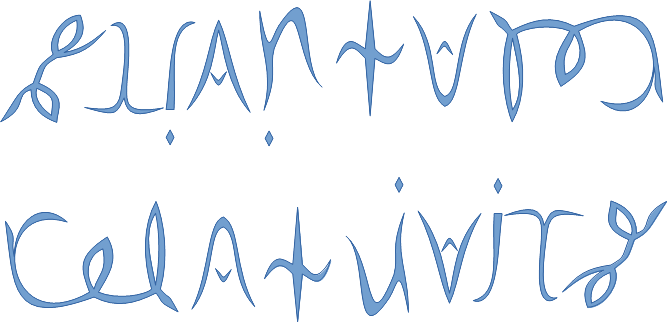See SRQM: QM from SR - The 4-Vector RoadMap (.html)
See SRQM: QM from SR - The 4-Vector RoadMap (.pdf)

SRQM Physics Diagramming Method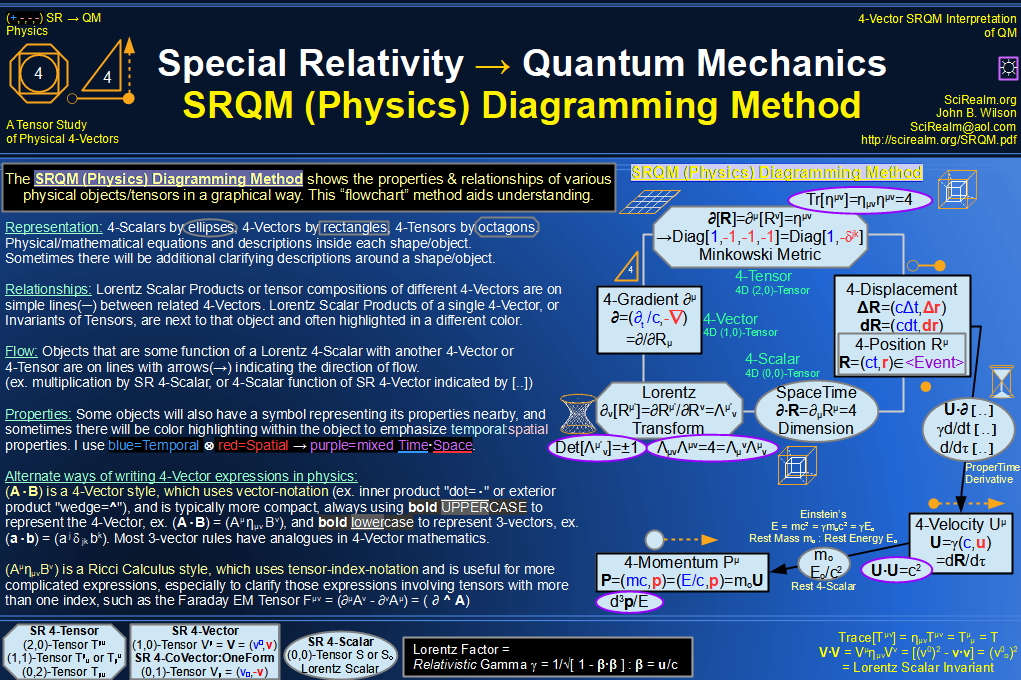SRQM 4-Vector : Four-Vector and Lorentz Scalar DiagramSRQM + EM 4-Vector : Four-Vector and Lorentz Scalar Diagram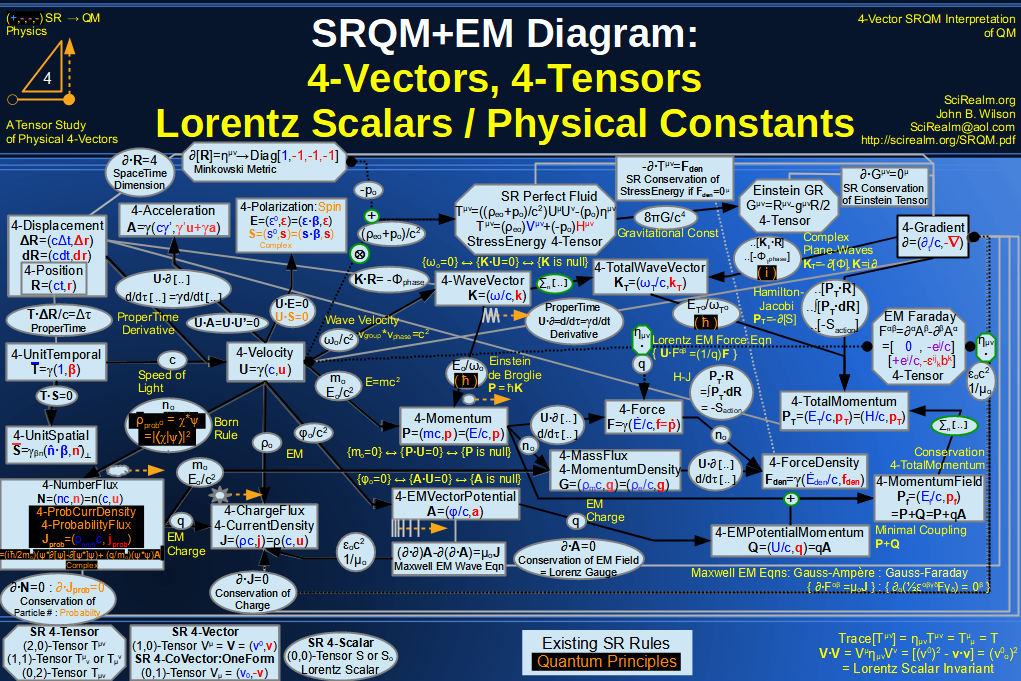SRQM + EM 4-Vector : Four-Vector and Lorentz Scalar Diagram With Tensor Invariants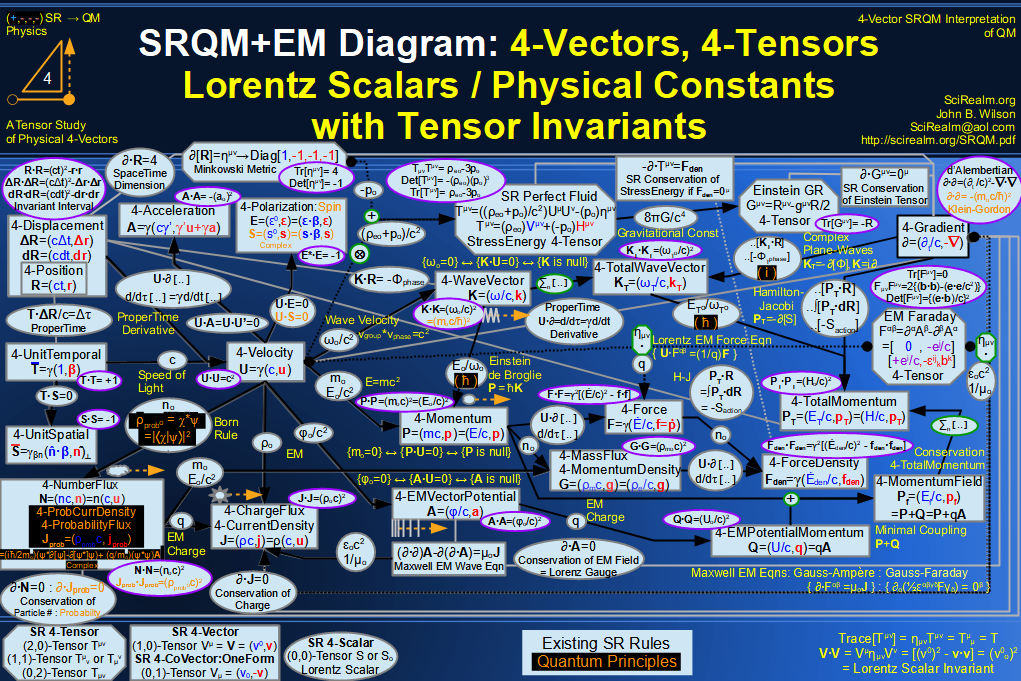SRQM 4-Vector : Four-Vector Stress-Energy & Projection Tensors Diagram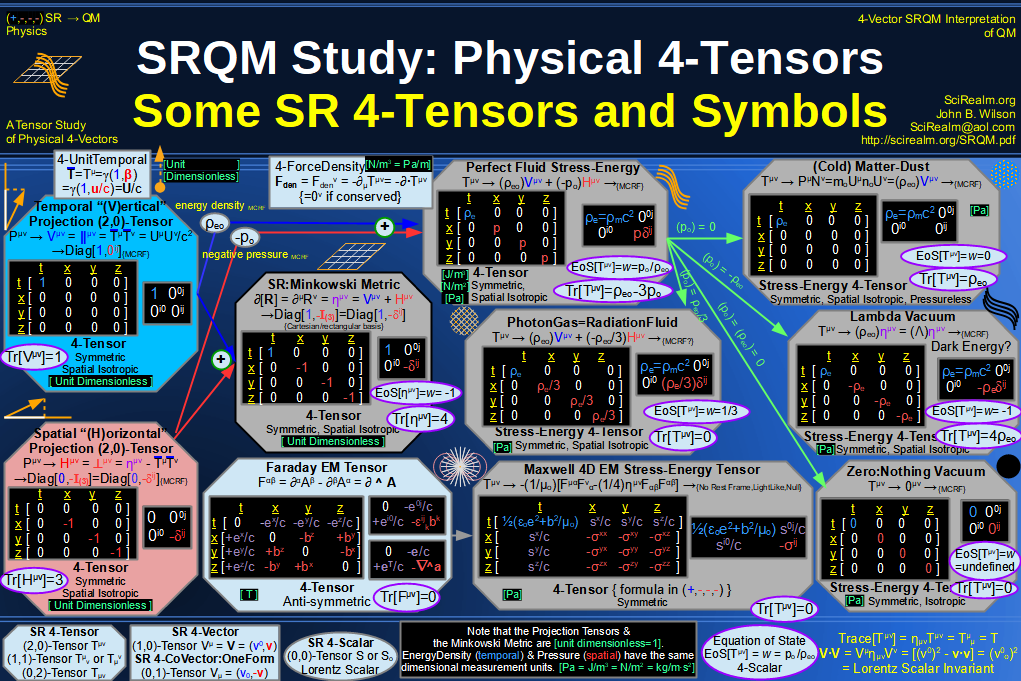SRQM 4-Vector : Four-Vector SR Quantum RoadMap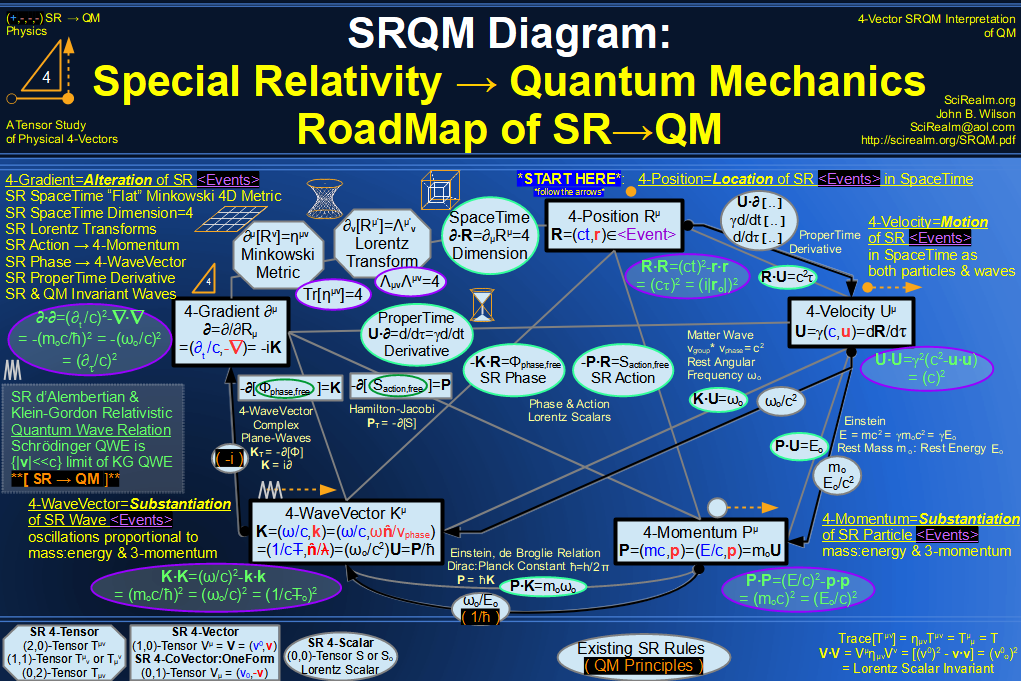SRQM + EM 4-Vector : Four-Vector SR Quantum RoadMap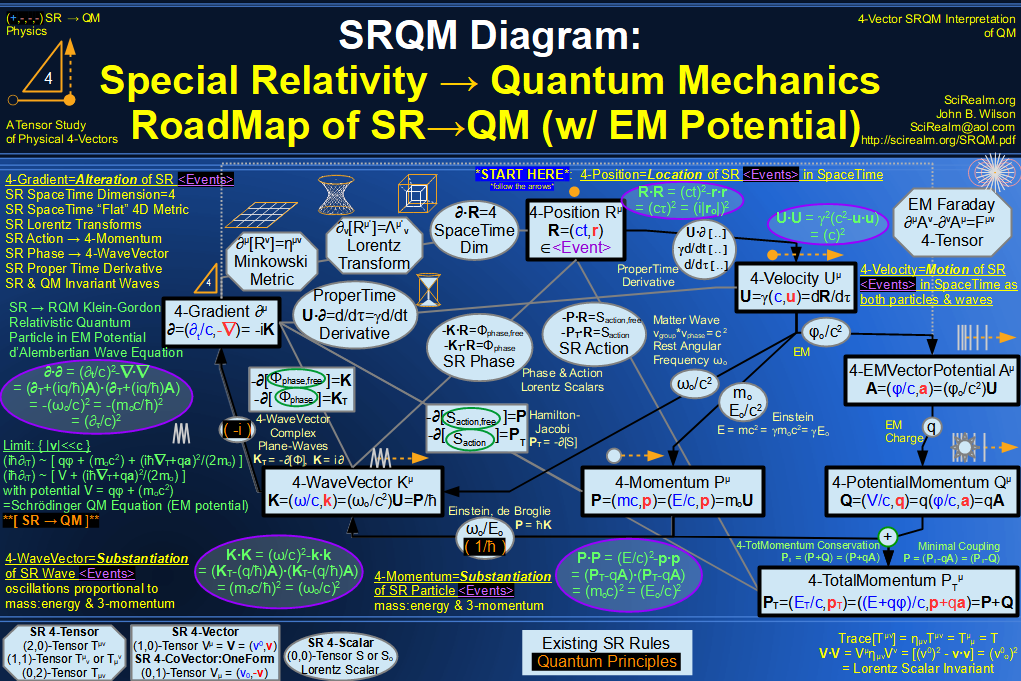SRQM + EM 4-Vector : Four-Vector SR Quantum RoadMap - Simple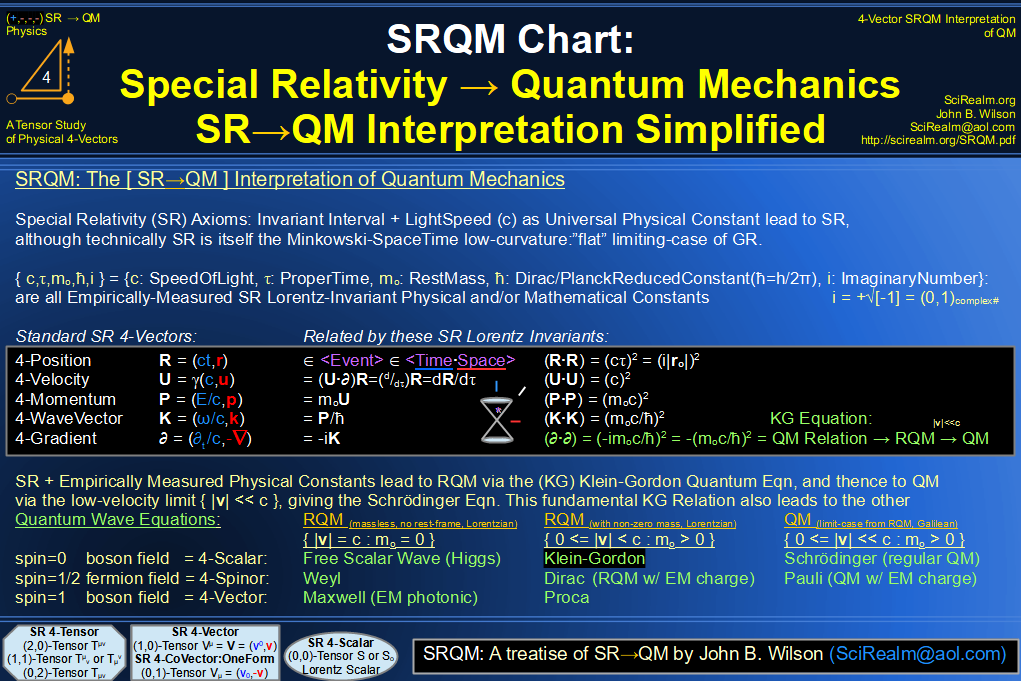SRQM 4-Vector : Four-Vector New Relativistic Quantum Paradigm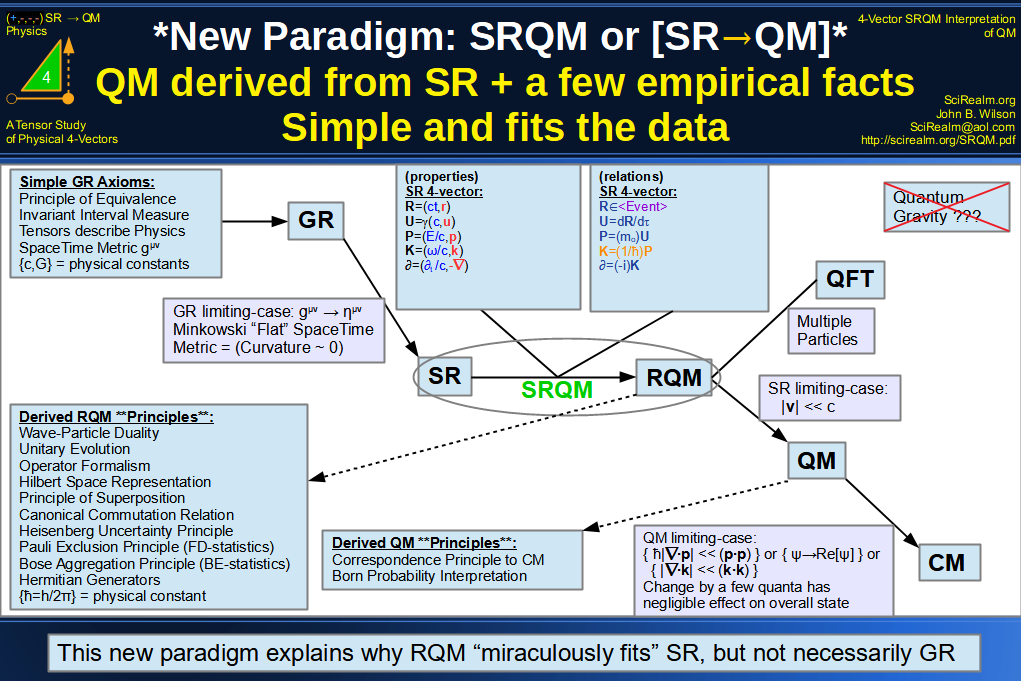SRQM + EM 4-Vector : Four-Vector New Relativistic Quantum Paradigm (with EM)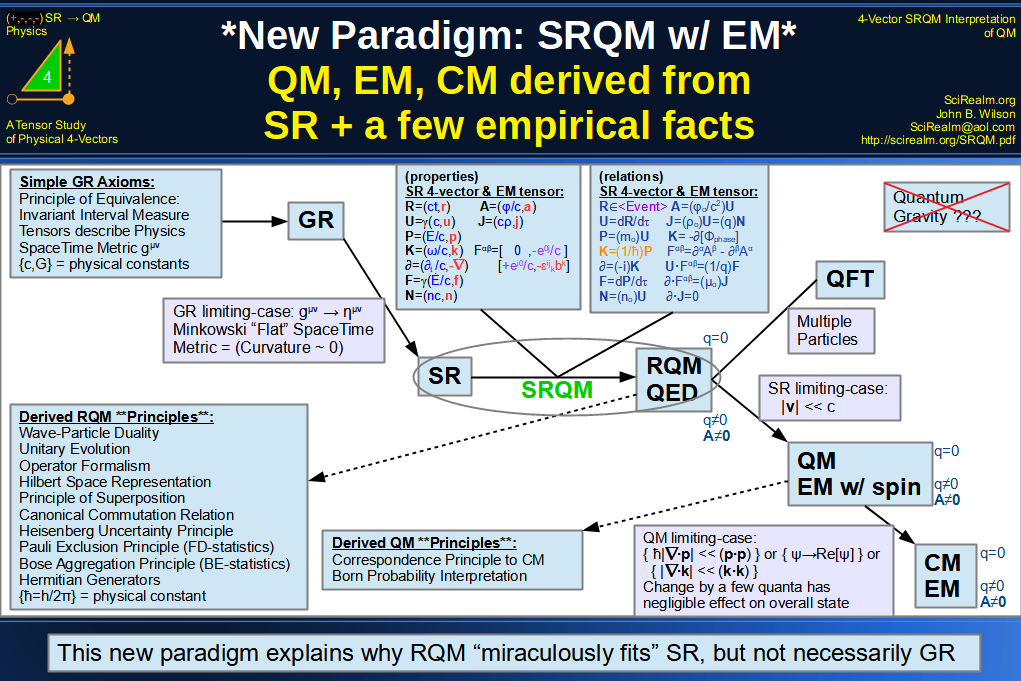SRQM 4-Vector : Four-Vector New Relativistic Quantum Paradigm - Venn Diagram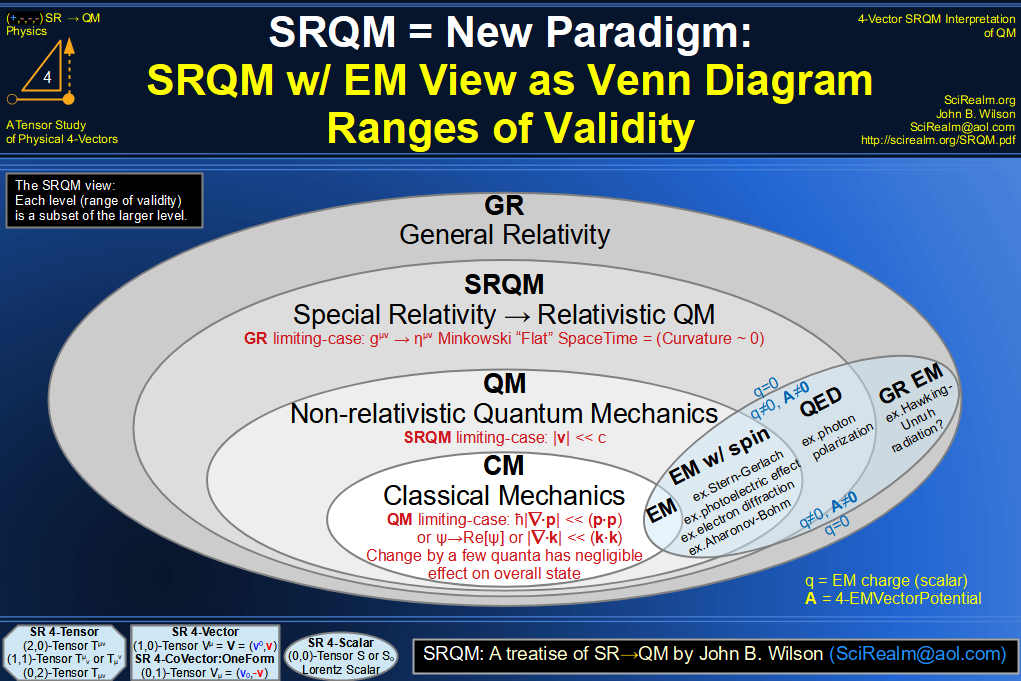SRQM 4-Vector : Four-Vector SpaceTime is 4D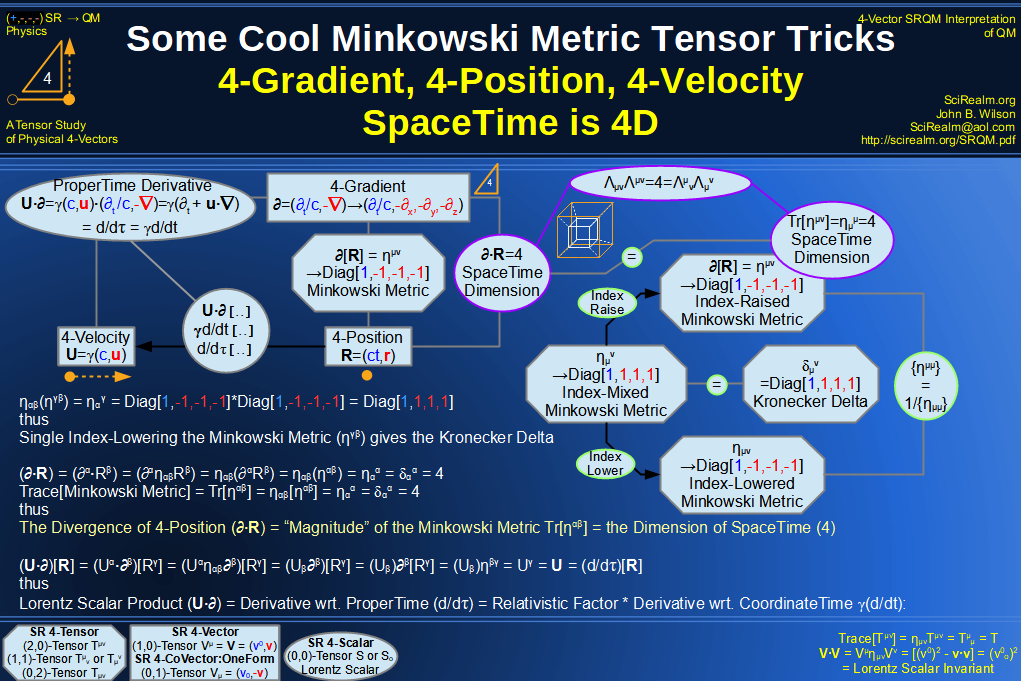SRQM 4-Vector : Four-Vector SpaceTime Orthogonality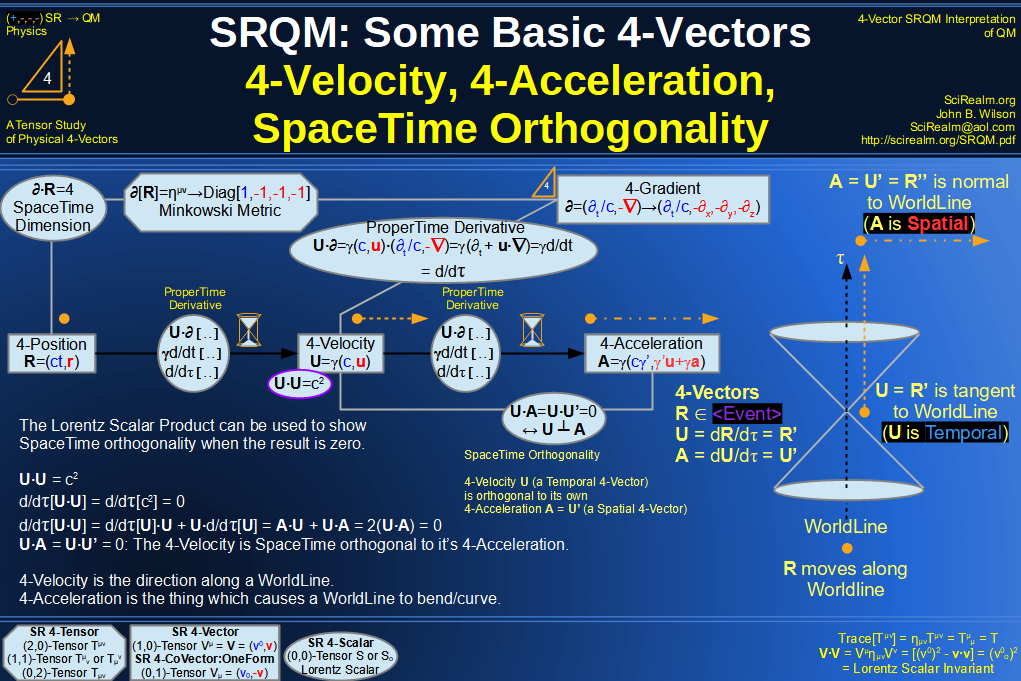SRQM 4-Vector : Four-Vector 4-Position, 4-Velocity, 4-Acceleration Diagram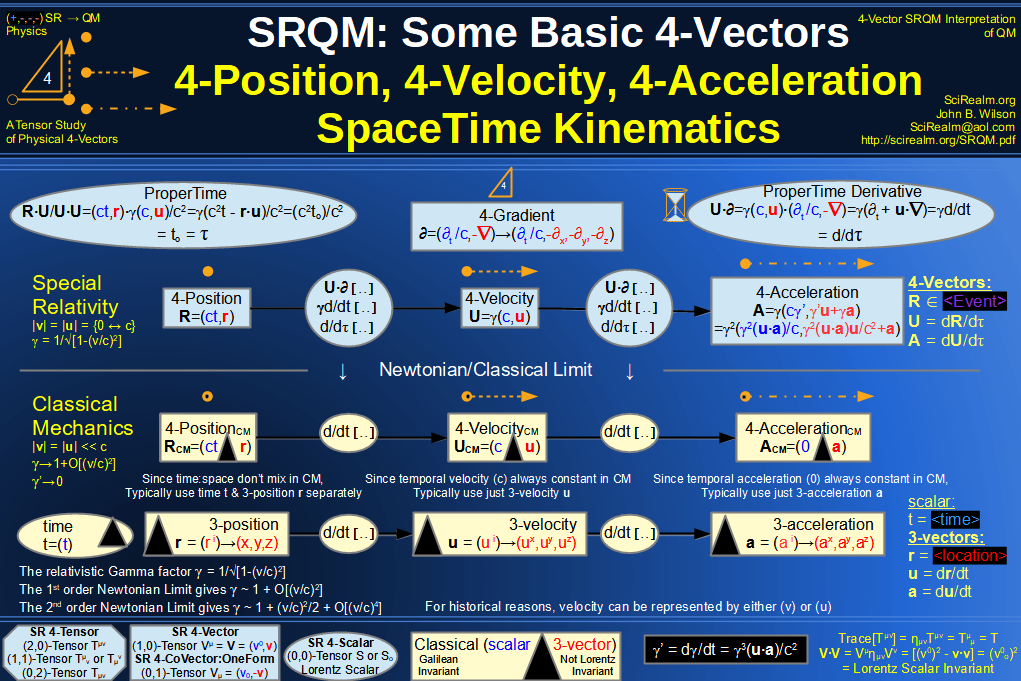SRQM 4-Vector : Four-Vector 4-Displacement, 4-Velocity, Relativity of Simultaneity Diagram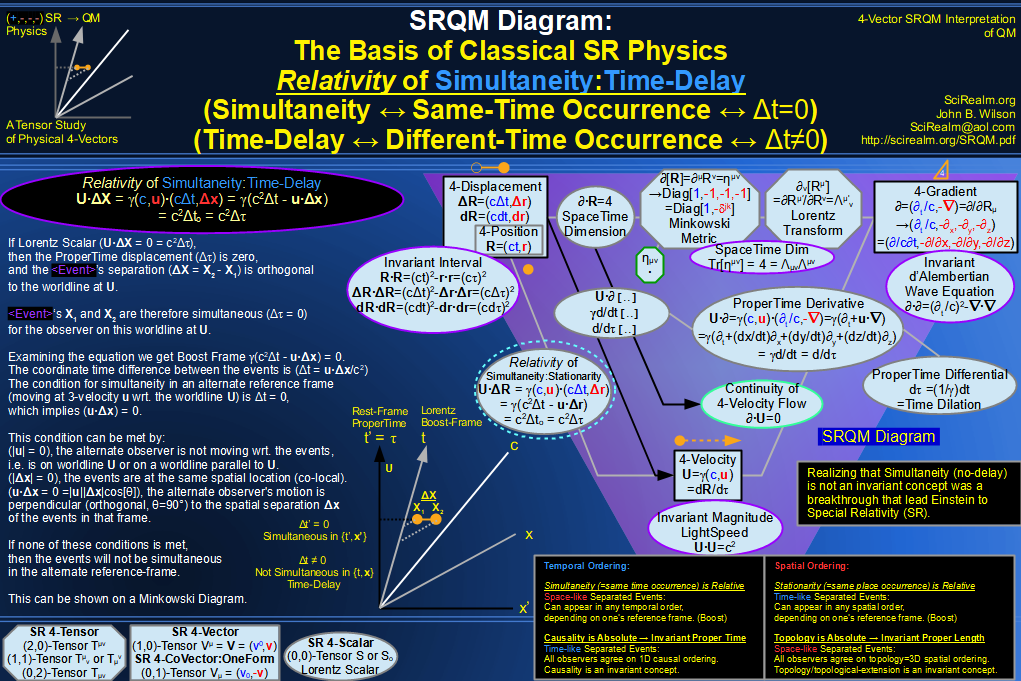SRQM 4-Vector : Four-Vector 4-Velocity, 4-Gradient, Time Dilation Diagram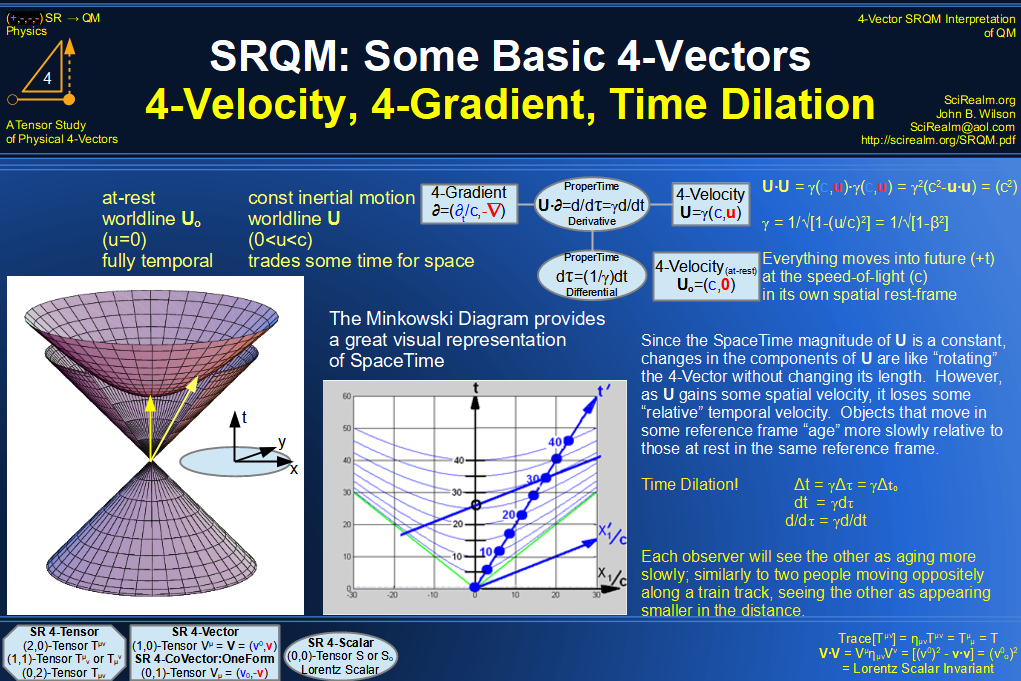SRQM 4-Vector : Four-Vector 4-Vector, 4-Velocity, 4-Momentum, E=mc^2 Diagram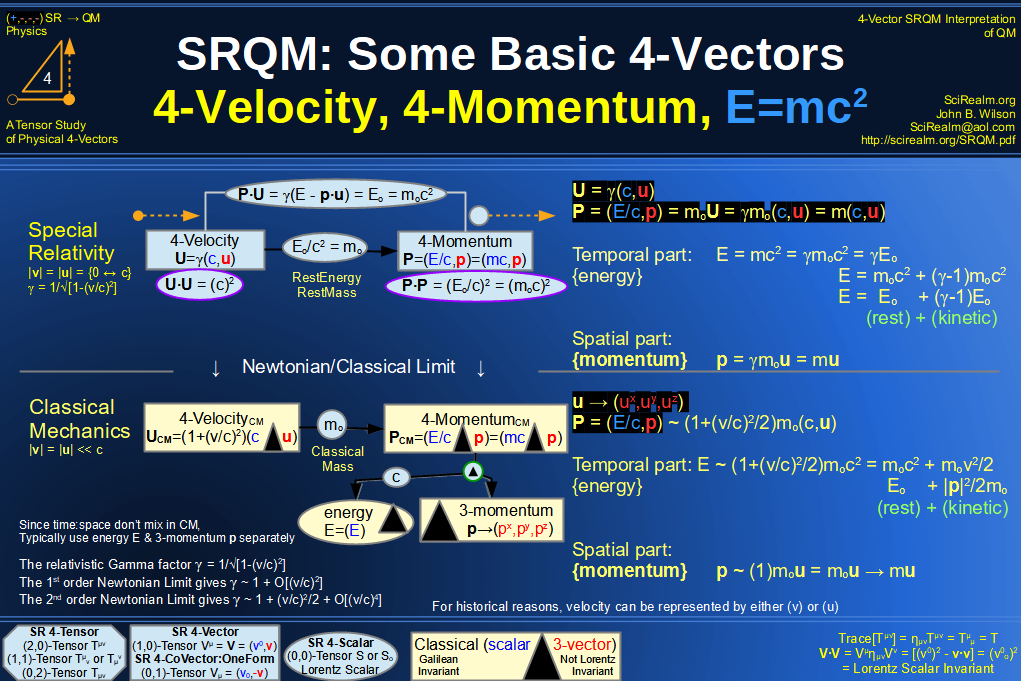SRQM 4-Vector : Four-Vector 4-Velocity, 4-WaveVector, Relativistic Doppler Effect Diagram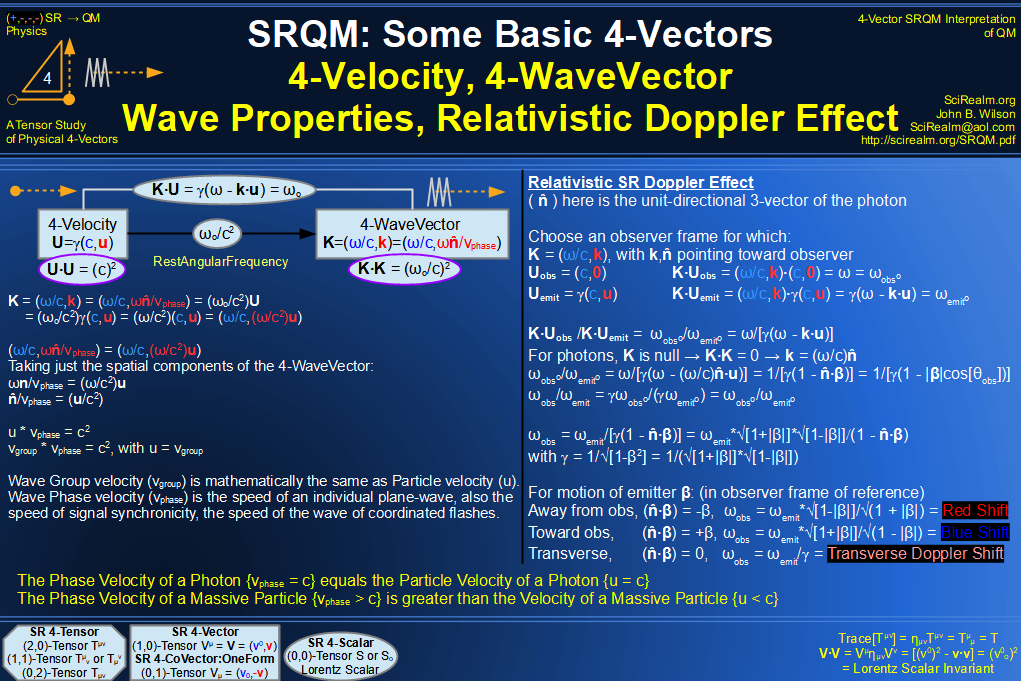SRQM 4-Vector : Four-Vector Wave-Particle Diagram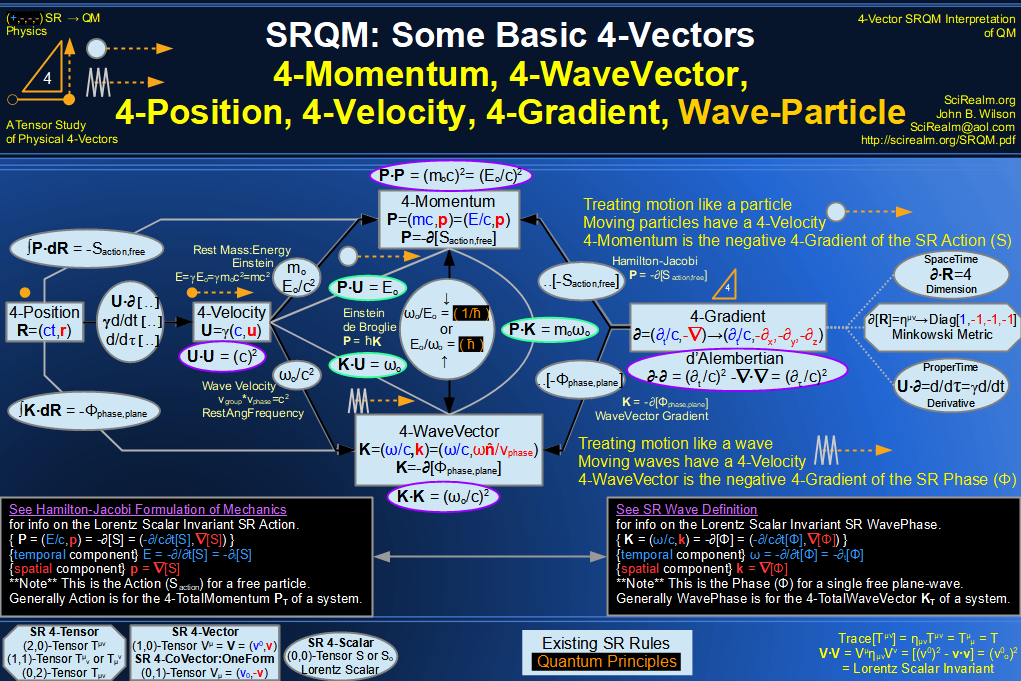SRQM 4-Vector : Four-Vector Compton Effect Diagram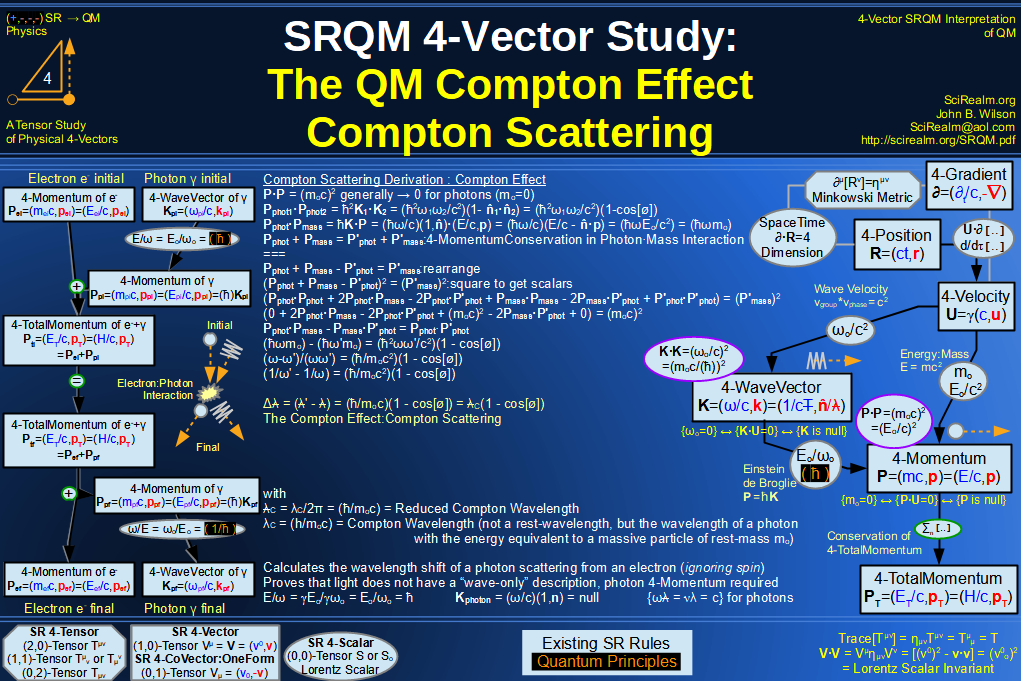SRQM 4-Vector : Four-Vector Aharonov-Bohm Effect Diagram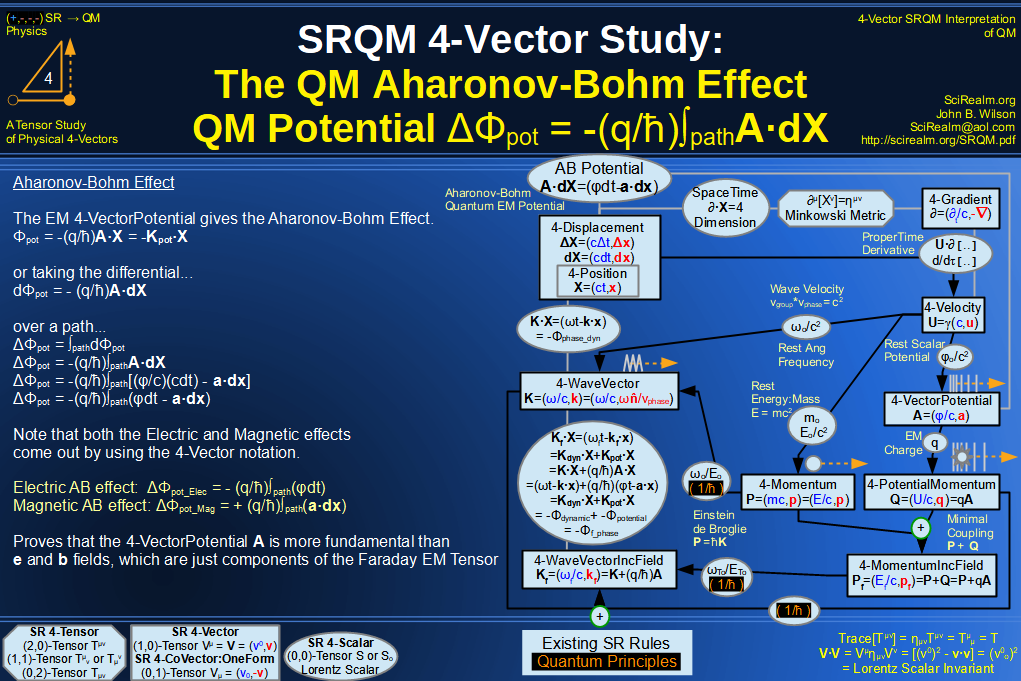SRQM 4-Vector : Four-Vector Josephson Junction Effect Diagram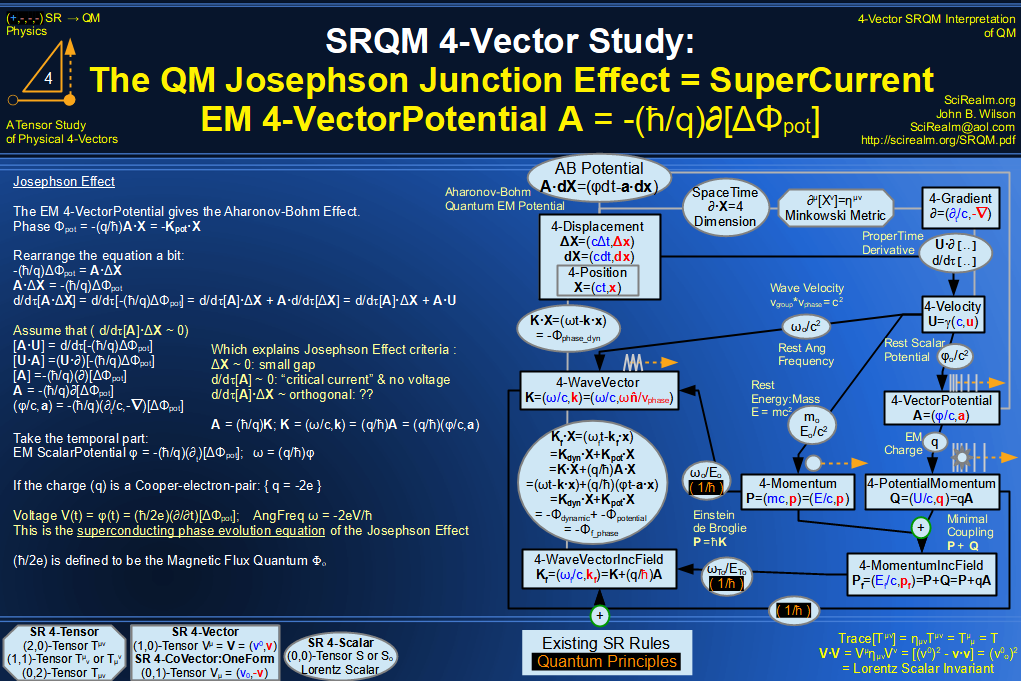SRQM 4-Vector : Four-Vector Hamilton-Jacobi vs Action, Josephson vs Aharonov-Bohm Diagram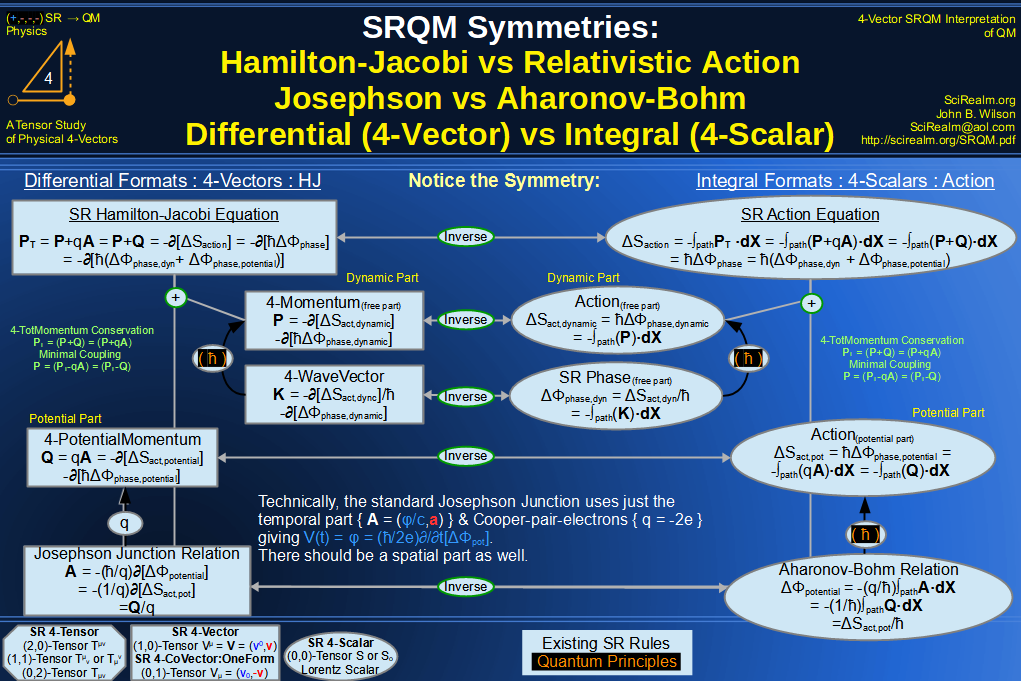SRQM 4-Vector : Four-Vector Motion of Lorentz Scalar Invariants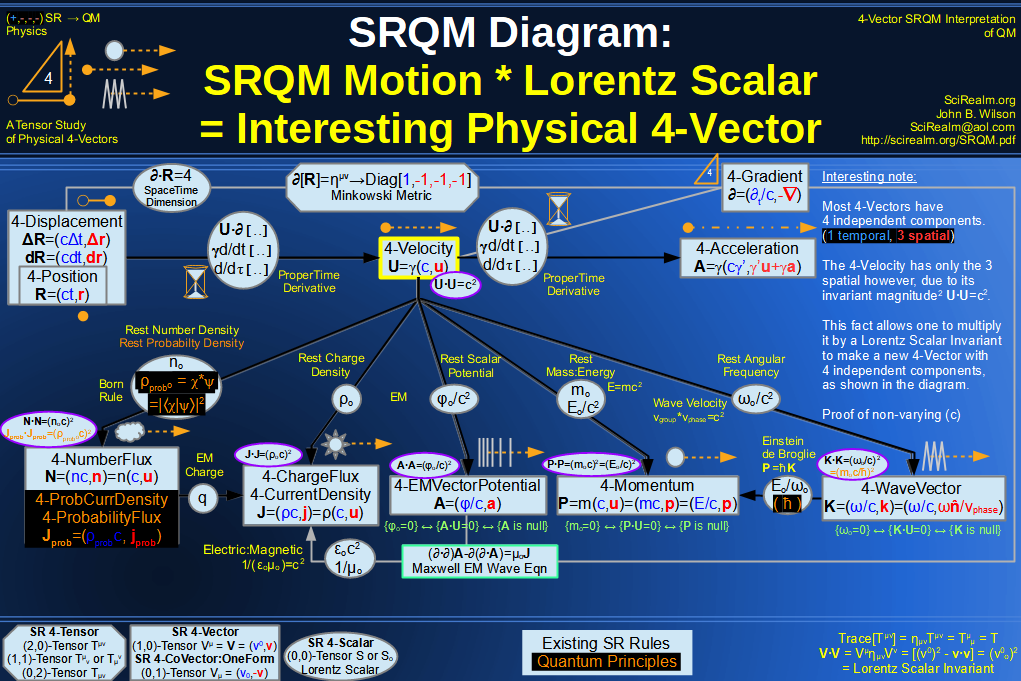SRQM 4-Vector : Four-Vector Motion of Lorentz Scalar Invariants, Conservation Laws & Continuity Equations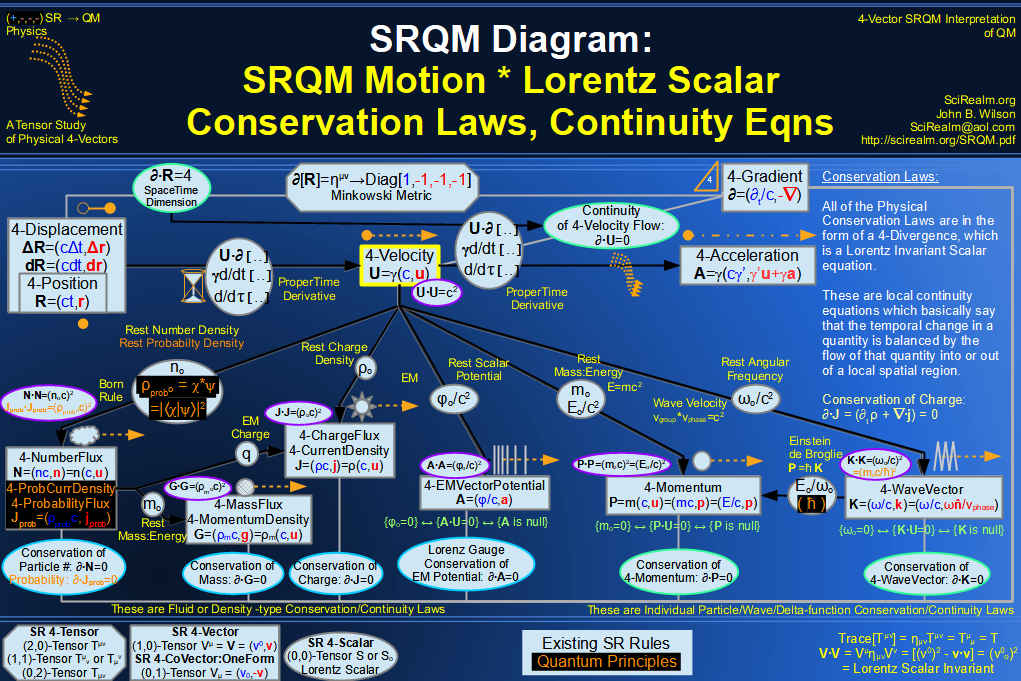SRQM 4-Vector : Four-Vector Speed of Light (c)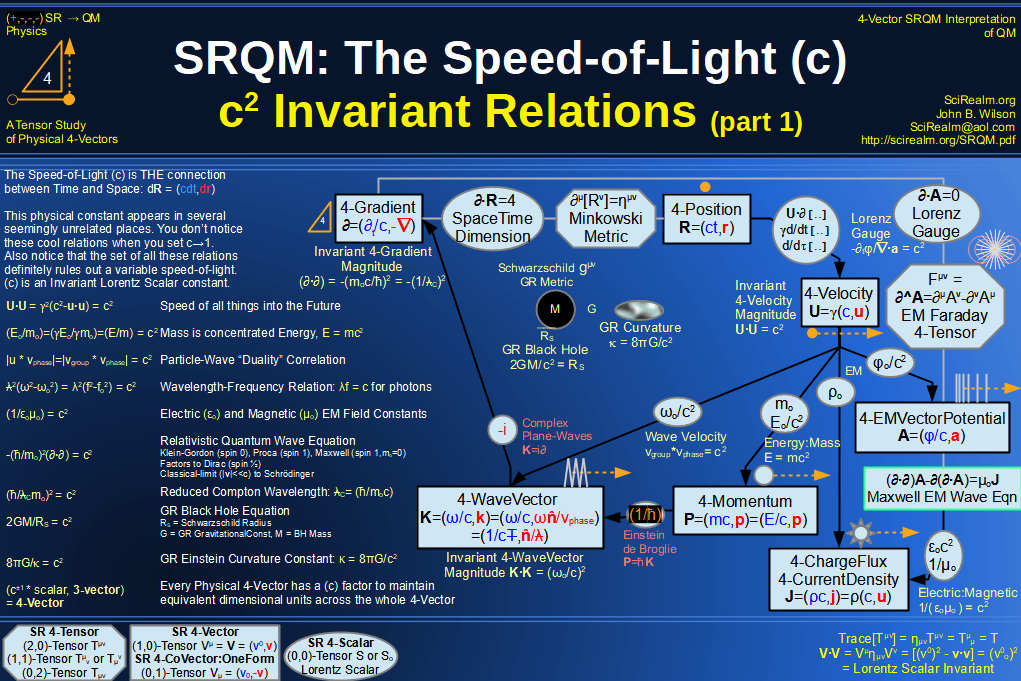SRQM 4-Vector : Four-Vector Minimal Coupling Conservation of 4-TotalMomentum)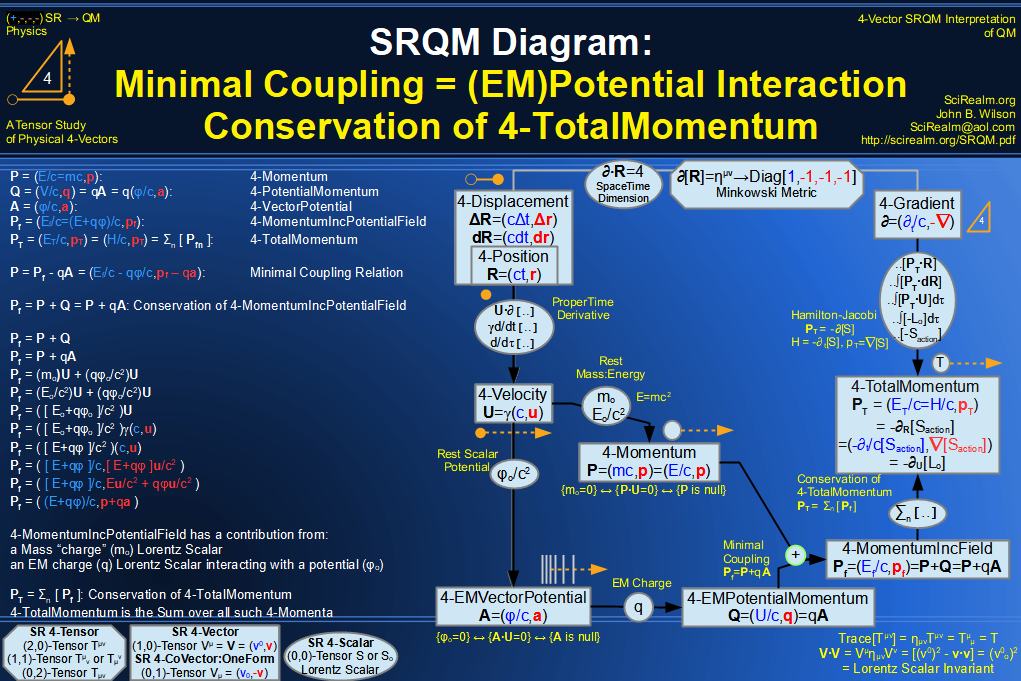SRQM 4-Vector : Four-Vector Relativistic Action (S) Diagram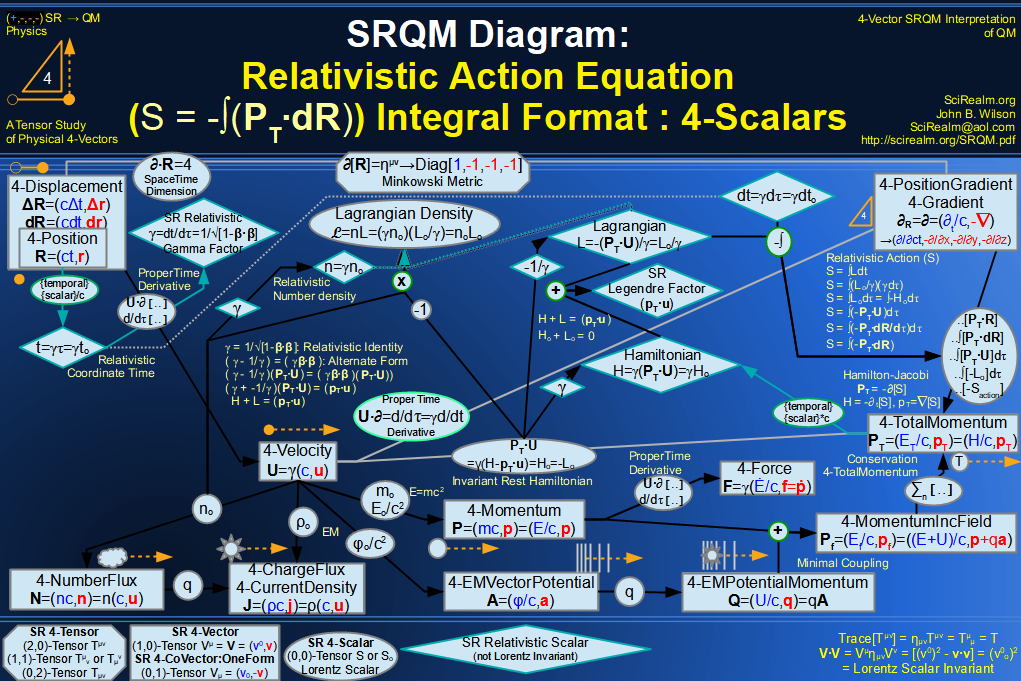SRQM 4-Vector : Four-Vector Relativistic Lagrangian Hamiltonian Diagram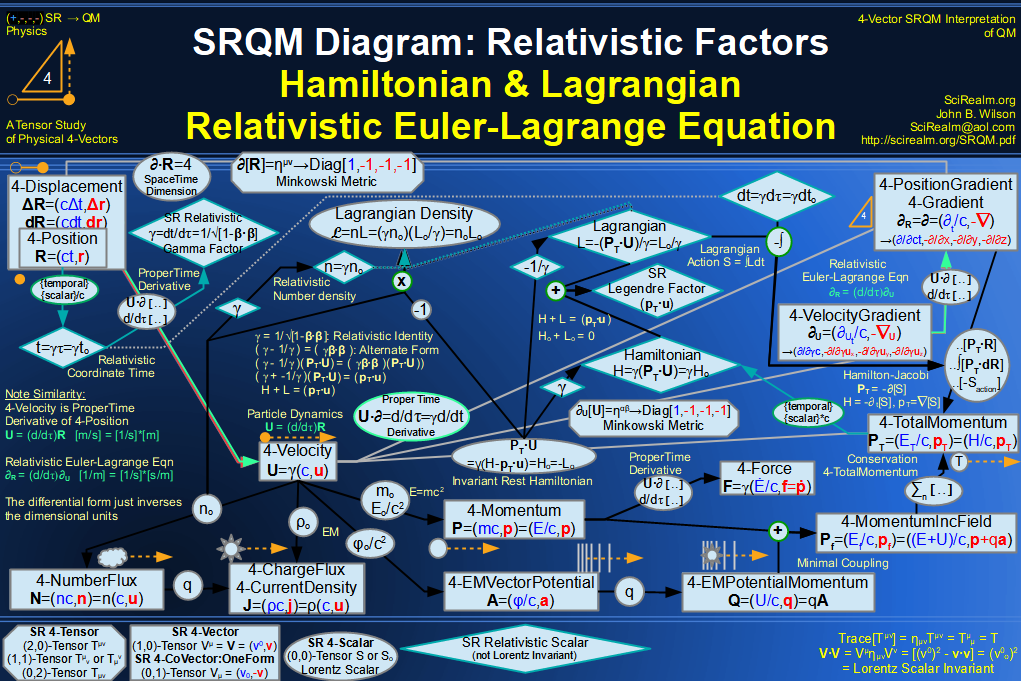SRQM 4-Vector : Four-Vector Relativistic Euler-Lagrange Equation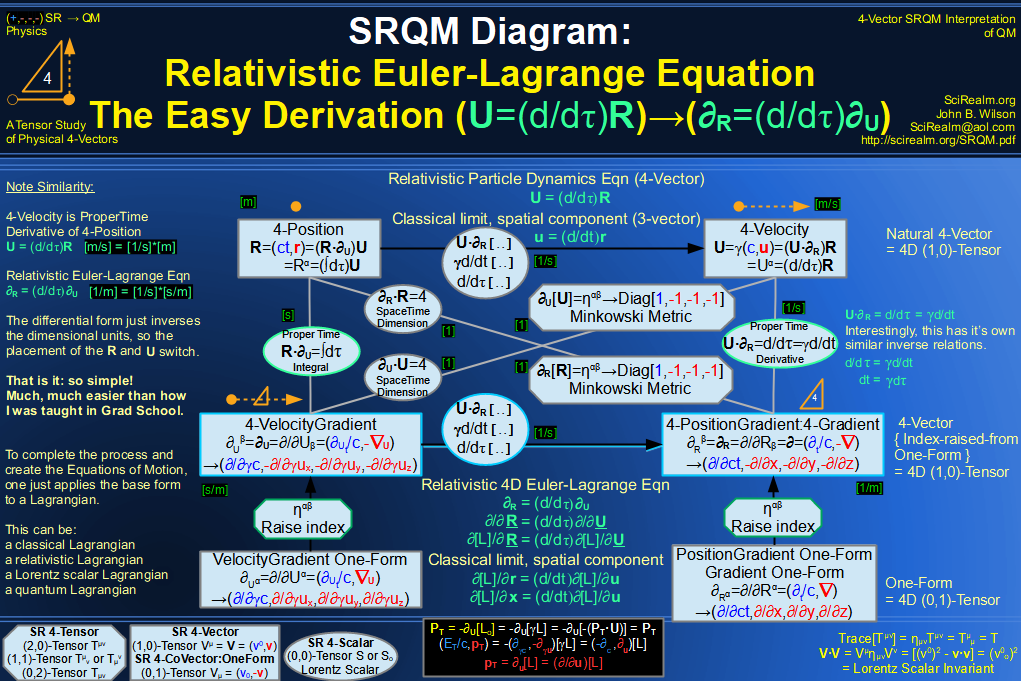SRQM 4-Vector : Four-Vector Relativistic EM Equations of Motion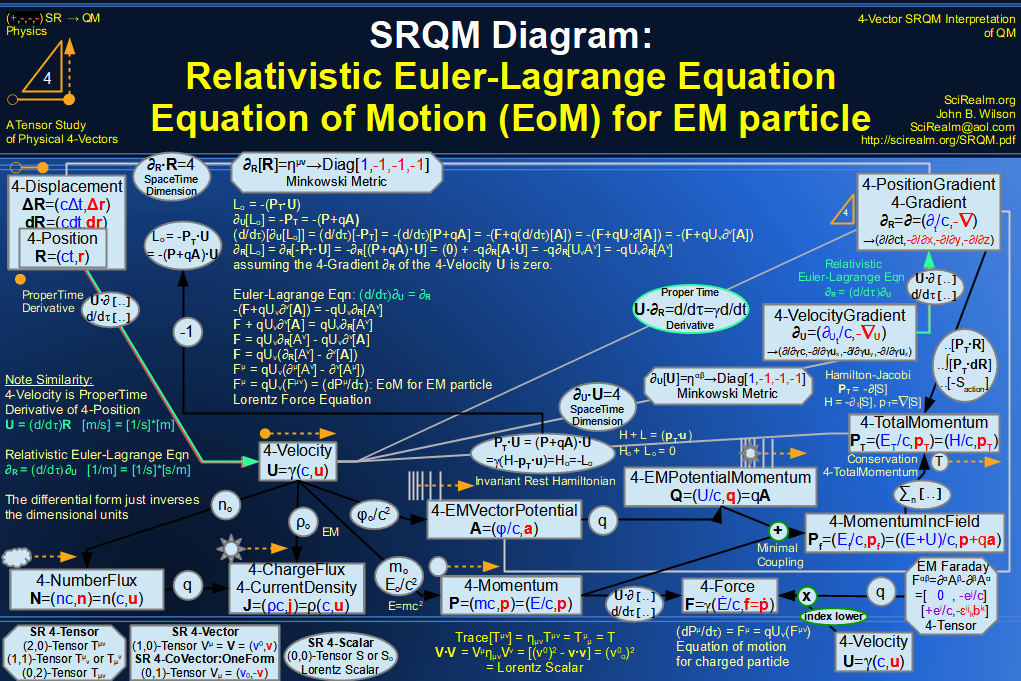SRQM 4-Vector : Four-Vector Einstein-de Broglie Relation hbar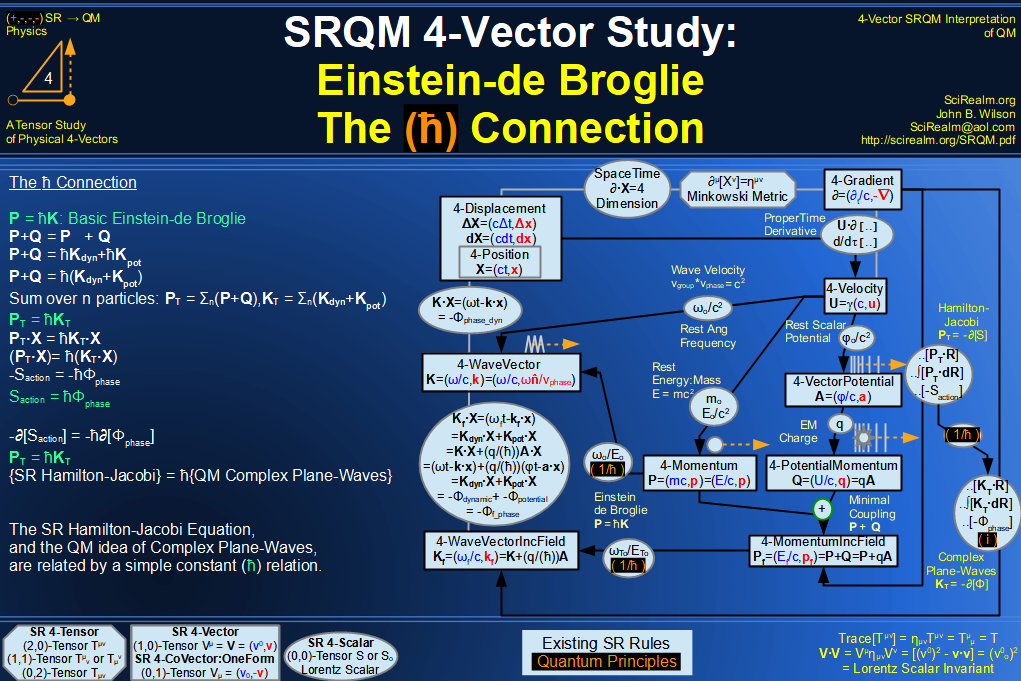SRQM 4-Vector : Four-Vector Quantum Canonical Commutation Relation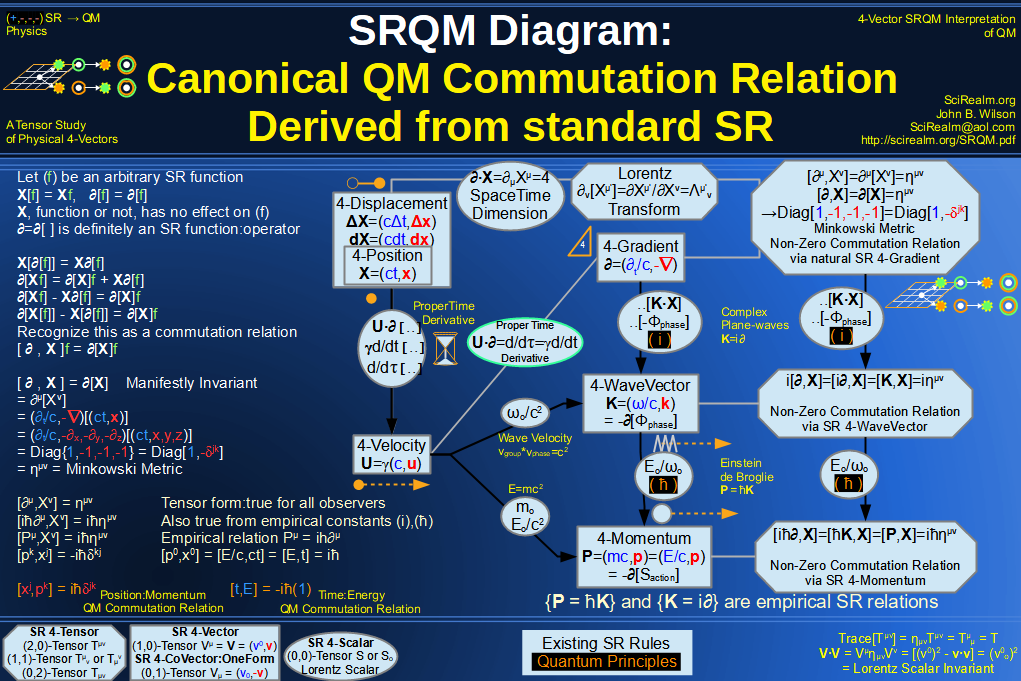SRQM 4-Vector : Four-Vector QM Schroedinger Relation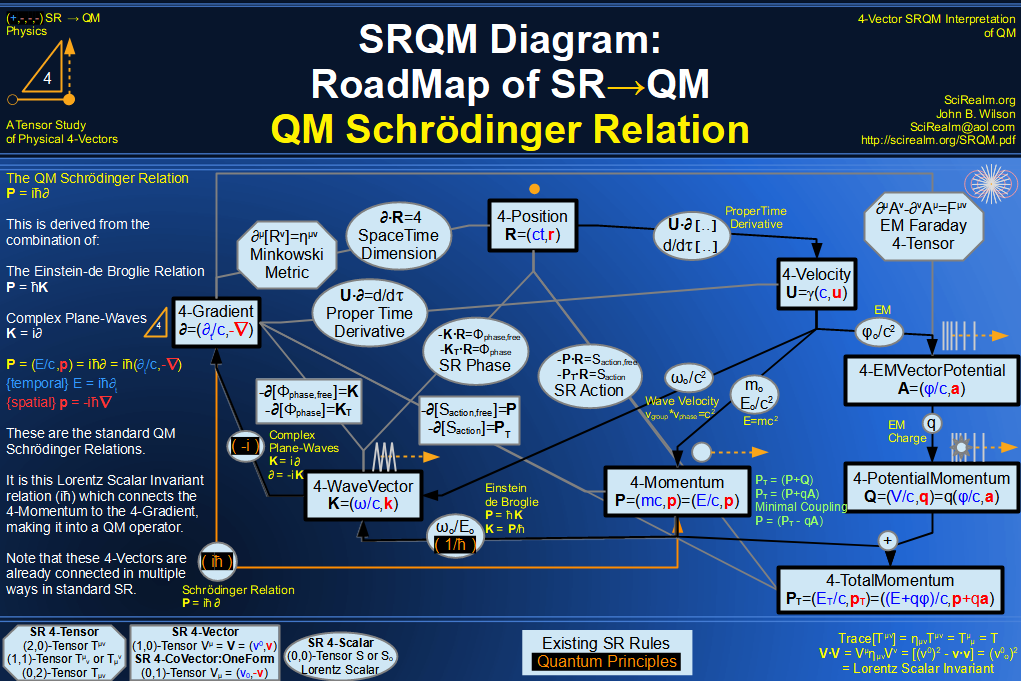SRQM 4-Vector : Four-Vector Quantum Probability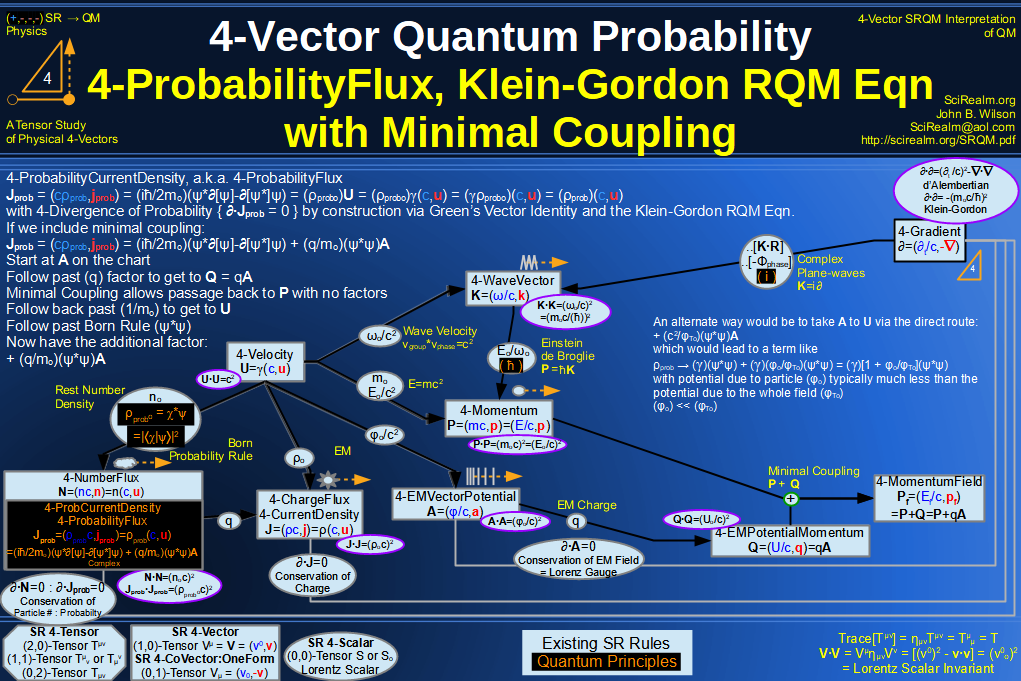SRQM 4-Vector : Four-Vector CPT Theorem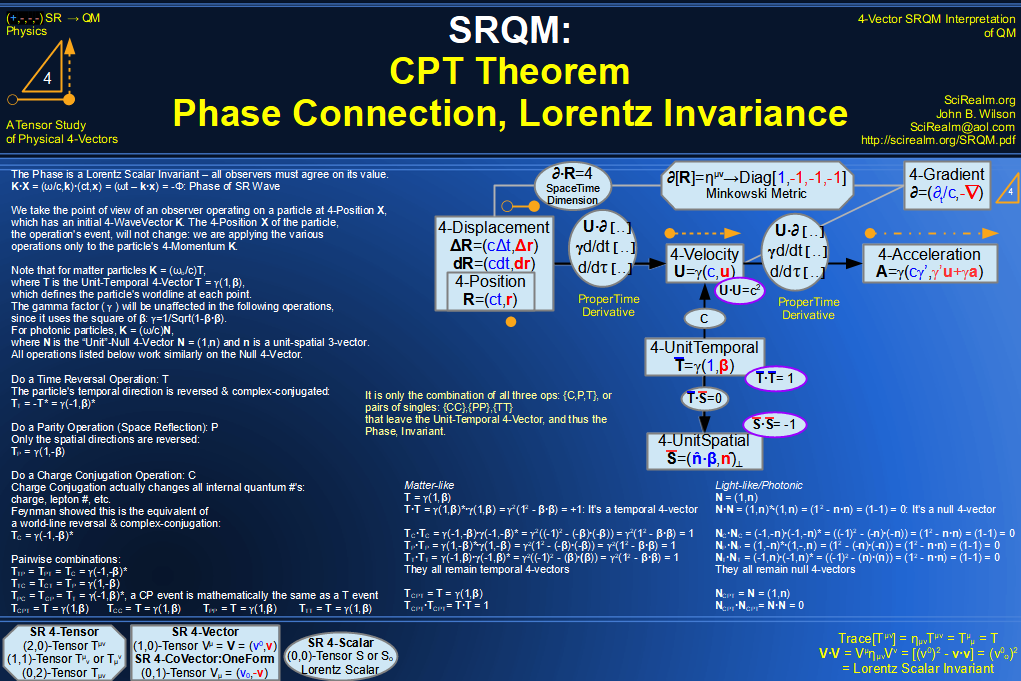SRQM 4-Vector : Four-Vector Lorentz Transforms Connection Map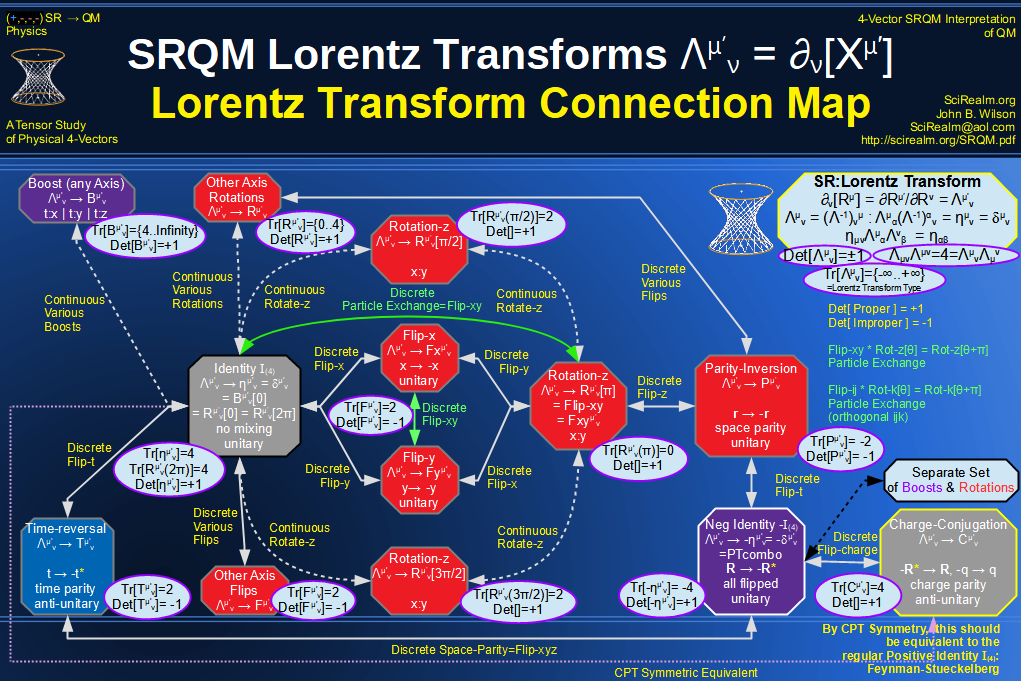SRQM 4-Vector : Four-Vector Lorentz Discrete Transforms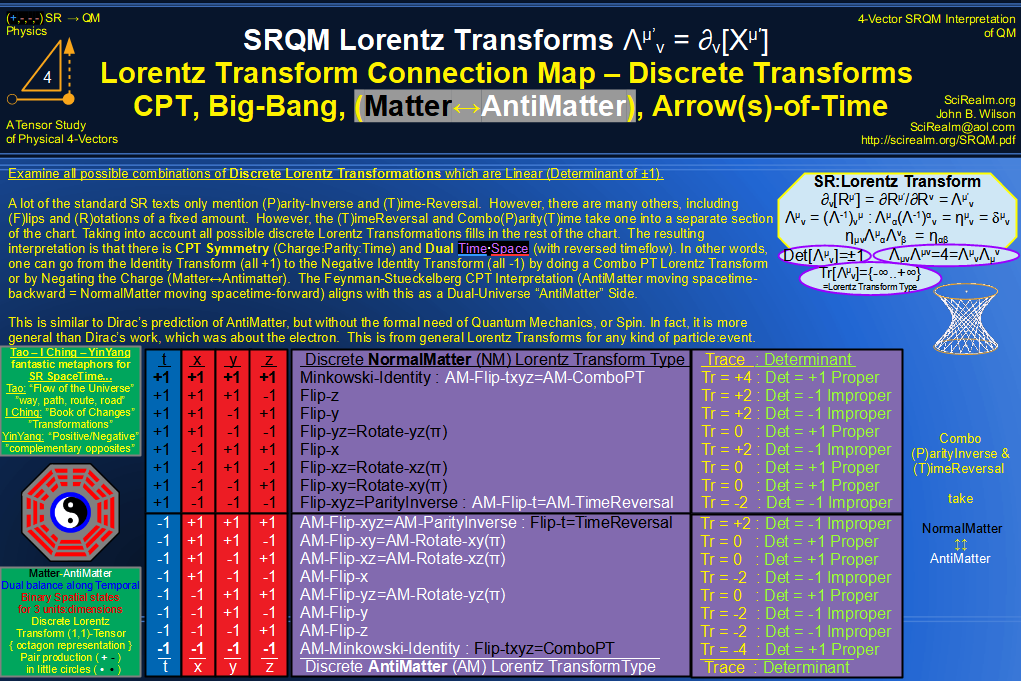SRQM 4-Vector : Four-Vector Lorentz Transforms - Trace Identification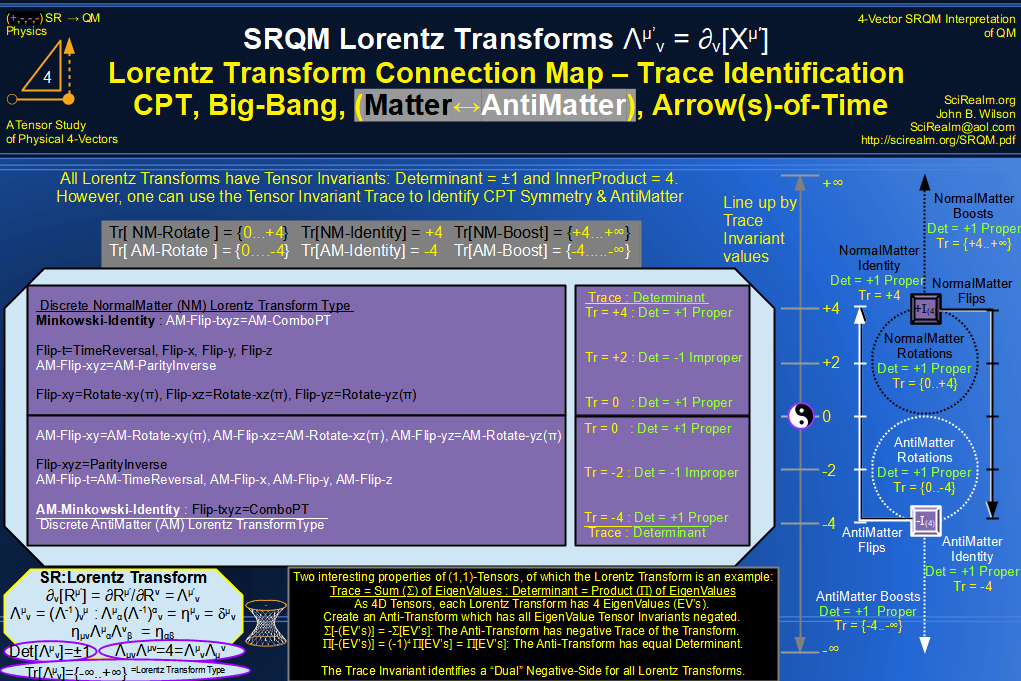SRQM 4-Vector : Four-Vector Lorentz Lorentz Transforms-Interpretations
CPT Symmetry, Baryon Asymmetry Problem Solution, Matter-Antimatter Symmetry Solution, Arrow-of-Time Problem Solution, Big-Bang!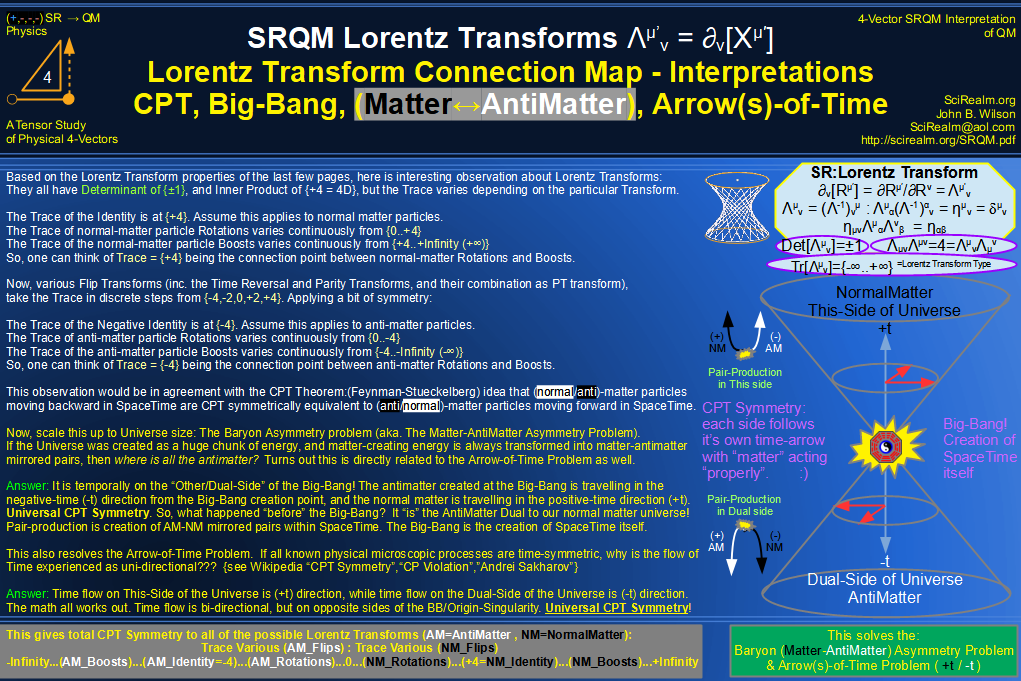See SRQM: QM from SR - The 4-Vector RoadMap (.html)
See SRQM: QM from SR - The 4-Vector RoadMap (.pdf)The Science Realm: John's Virtual Sci-Tech Universe John's Science & Math Stuff: | About John | Send email to John 4-Vectors | Ambigrams | Antipodes | Covert Ops Fragments | Cyrillic Projector | Forced Induction (Sums Of Powers Of Integers) | Fractals | Frontiers | JavaScript Graphics | Kid Science | Kryptos | Philosophy | Photography | Prime Sieve | QM from SR | QM from SR-Simple RoadMap | SR 4-Vector & Tensor Calculator | Quantum Phase | Quotes | RuneQuest Cipher Challenge | Scientific Calculator (complex capable) | Secret Codes & Ciphers | Science+Math | Sci-Pan Pantheist Poems | Stereograms | SuperMagicSqr4x4 | Turkish Grammar | Quantum Mechanics is derivable from Special Relativity See SRQM - QM from SR - Simple RoadMap (.html) See SRQM - QM from SR - Simple RoadMap (.pdf)